# Abstract

Regarding High Cycle Fatigue, a classic damage model is presented in combination with an automatic load advancing strategy that saves computational time when dealing with load histories of millions of cycles. Numerical examples are shown in order to demonstrate the capabilities of the advancing strategy and a validation of the model is done on small scale samples.

A new constitutive model is presented for Low Cycle Fatigue that uses the classic plasticity and damage theories and simultaneously integrates both processes in the softening regime. The capabilities of the model are shown in numerical examples.

Finally, the High Cycle Fatigue damage model is applied to the constituents of a composite material and the structural behaviour is obtained by means of the serial/parallel rule of mixtures. Validation of the constitutive formulation is done on pultruded glass fiber reinforced polymer profiles.

# Resumen

El presente trabajo propone una metodología innovadora para la modelización numérica de la rotura de materiales metálicos y compuestos sometidos a cargas cíclicas. El enfoque es fenomenológico y la formulación se calibra con resultados experimentales obtenidos en especímenes a pequeña escala y con experimentos a gran escala. Este trabajo abarca procesos de fatiga desde alto número de ciclos hasta muy bajo número de ciclos.

Una evaluación del estado del arte hasta el momento se ha llevado a cabo para los diferentes tipos de fatiga. A continuación, se propone una nueva ley constitutiva para la fatiga de muy bajo número de ciclos y se presenta la validación con resultados experimentales obtenidos en especímenes a escala pequeña. El modelo constitutivo se ha probado en dos aplicaciones industriales: una tubería de gran diámetro bajo condiciones de carga monótonas y una tubería doblada a un ángulo de 90 grados sometida a cargas cíclicas. Se ha enfatizado la capacidad del modelo de reproducir diferentes modos de rotura dependiendo de las condiciones de carga. El trabajo referente a este modelo se ha usado en el marco del proyecto europeo: “Fatiga de muy bajo número de ciclos del acero bajo grandes deformaciones cíclicas”.

Respecto a la fatiga de alto número de ciclos, se presenta un modelo clásico de daño combinado con una estrategia automatizada de avance en la carga por número de ciclos. La estrategia conduce a un ahorro en tiempo de computación cuando se aplican millones de ciclos de carga. Las capacidades y particularidades de la estrategia de avance en la carga se enseñan en una serie de ejemplos numéricos. El modelo se valida con resultados experimentales obtenidos en especímenes a pequeña escala.

Un nuevo modelo constitutivo se presenta para la fatiga de bajo número de ciclos que se basa en las teorías básicas de plasticidad y daño y que integra simultáneamente las ecuaciones de ambos procesos en el régimen de ablandamiento. Las capacidades del modelo se enseñan a través de ejemplos numéricos.

Finalmente, se estudia la aplicación del modelo de daño para fatiga de alto número de ciclos en los componentes de materiales compuestos. El comportamiento estructural del material compuesto se obtiene a través de la teoría de mezclas serie/paralelo. La formulación se valida con resultados experimentales obtenidos en perfiles de GFRP.

# Acknowledgements

I would like to thank all the people that in some way have helped me to complete my PhD.

I would like to express my gratitude to my PhD advisors, Sergio Oller, Xavier Martinez and Álex Barbat, for their faith in me and for their dedication during these years. You have contributed greatly in shaping my understanding and my knowledge both at a scientific and at a personal level. I consider myself immensely fortunate to have had the opportunity to learn from you.

I would like to thank the Spanish Minisitry of Education for the FPU Grant I have received during my PhD years and the European Union for the funding given to the "Ultra low cycle fatigue under high-strain loading conditions" project. Also, my PhD advisors for giving me the opportunity to participate in this project. The knowledge generated from the project constitutes an important part of this work.

I would like to express my gratitude to CIMNE at an institutional level for all the opportunities it has offered me in attending international conferences and for participating and assisting at many high-quality lectures held in its premises. I have found here a family away from home. Thank you Kike and Miguel for always being there when I had troubles with GiD and, together with Salva and Guillermo, for all the conversations we enjoyed daily at lunch. It has been very refreshing and thought provoking to share ideas and opinions with you and together we have made many great memories.

I would like to give a special thank you to Álex, Manuel and Stefano, my office colleagues, who have made my day to day entertaining during these years. Also, Fermin and Ester, my PLCd group mates. Thank you for all the help you have given me in these last months and for all the trips and outings we enjoyed together. Because you work so well, my work was made easier and has gained a lot.

To my dear parents, thank you for the way you raised me. I owe to you the person I am today. Pentru dragii mei parinti, va multumesc pentru felul in care m-ati crescut. Va datorez ceea ce sunt astazi.

Finally, I would like to thank my husband, Miguel Ángel Celigueta. Sharing these years with you has been one of the most intense learning experiences I have ever had. Thank you for your wisdom, your patience and your love.

Pentru bunicii mei! Mi-e tare dor de voi...

Para mis abuelos! Os echo tanto de menos...

"It is not because things are difficult that we do not dare, it is because we do not dare that they are difficult"

Seneca

"Both if you think you can do it and if you don't, you are in the right"

Henry T. Ford

# 1 Introduction, objectives and motivation

## 1.1 Antecedents

The present work aims at advancing an innovative computational methodology that simulates metal and composite material fracture under cyclic loading following a phenomenological approach, with calibration from both small scale and large scale testing.

Time varying cyclic loads produce failure of structural parts for values of stress lower than those obtained in static tests. This phenomenon is called fatigue and it is defined more generally in the ASTM E1823 standard  as:

the process of permanent, progressive and localized structural change which occurs to a material point subjected to strains and stresses of variable amplitudes which produces cracks which lead to total failure after a certain number of cycles.

In this definition it is possible to include all fatigue ranges, from “Ultra Low Cycle Fatigue” (ULCF), to “Low Cycle Fatigue” (LCF) and “High Cycle Fatigue” (HCF). Generally it is considered that failures in the range of 106 to 108 cycles belong to the HCF range, failures below 100 cycles belong to ULCF, and failures in between these limits are atributed to LCF.

One of the main drawbacks of most of the existing formulations to characterize ULCF, LCF and HCF is that they require regular cycles to predict material failure, or they couple the effects of non-regular cycles using the Miner rule, which requires knowing the performance of the structure under regular cycles. However, this regularity often does not exist. An example of an ULCF failure due to an irregular cyclic load is found in the failure of structures subjected to seismic loads, where the frequency varies along time and each cycle may have different amplitudes.

A Continuum Damage Mechanics (CDM) based fatigue model has been previously derived at CIMNE to address low- and high-cycle fatigue  and the present work is based on the thermodynamic framework adopted there. Given the extensive experience of the workgroup in constitutive modelling of composite materials, the application on long fiber composite materials of the formulation developed is also studied.

## 1.2 Motivation

This work addresses fatigue processes ranging from high cycle to ultra-low-cycle fatigue. While high cycle fatigue (HCF) in metals is a largely documented phenomena, less is known about its ultra-low-cycle counterpart, a phenomena caused by extreme loading conditions (e.g. earthquakes, hurricanes, support settlements) characterized by large scale yielding and large deformations which lead to fracture.

The issue of fracture is dealt with in a fundamental way in this work by examining the accuracy and validity of evolving approaches to characterize extreme loading-induced fracture in steel structures. Finite element modelling is extensively used to describe the stress and strain distribution and evolution during specimen loading. An experimental program of large scale tests pertaining to the European Project Ultra low cycle fatigue of steel under cyclic high-strain loading conditions is also used to validate and demonstrate the constitutive models proposed under monotonic and cyclic loadings.

Based on an evaluation of the current state of the art, three constitutive models are proposed in this work, that derive from a common thermodynamical and mechanical base. They make use of the basic theories of damage and plasticity in order to simulate the behaviour of materials subjected to fatigue due to complex loading scenarios. The nonlinear behaviour of the materials is reflected directly in the constitutive equation without any need of additional fracture criteria. The formulations have a wide range of applicability, both for monotonic and cyclic loading.

The effect of fatigue in composites is still an open issue not yet resolved. With the increasing use of composite materials comes an increasing need to understand their behaviour and design life. Many of the nowadays composite applications encompass conditions that include repetitive loading cycles, thus demanding the ability to understand and evaluate fatigue in composite structures.Following these considerations, one of the formulations shown in this monograph is also applied to composite materials with successful results, proving that the Continuum Damage Mechanics approach can be used for these materials.

## 1.3 Objectives

The main objective of this work is advancing an innovative computational methodology that simulates metal and composite material fracture under cyclic loading following a phenomenological approach, with calibration from both small scale and large scale testing.

The completion of this objective has been conditioned by the fulfillment of several specific objectives that may be summarized as follows:

1. Development of constitutive laws for the description of the evolution of plastic behaviour based on the observed micromechanisms and experimental testing under ULCF conditions;
2. Development of constitutive laws for the description of damage evolution based on the observed micromechanisms and experimental testing under HCF conditions;
3. Development of a constitutive model for the description of plasticity and damage evolution based on the observed micromechanisms and experimental testing under LCF conditions;
4. Development of an automatic load-advancing strategy that reduces and optimizes the computational time of a numerical simulation.
5. Development of constitutive laws for the description of damage evolution under cyclic loading in composites using the serial/parallel mixing theory.

## 1.4 Outline of the monograph

The document begins with a short introduction on the general topic of fatigue followed by a review of the state of the art on processes responsible for extreme loading conditions-induced fracture, and then applying the state of the art micromechanics models for explaining cyclic fracture and fatigue prediction, first for steel members, extending the question further to composite materials.

The limitations of the existing approaches are described, knowledge gaps identified and issues regarding the implementation of these approaches are critically assessed. There will be a particular emphasis placed on damage initiation and spread due to extreme cyclic loading, known as ultra-low-cycle fatigue (number of cycles to failure on the order of 102). A review of fatigue life prediction methods in composites is then also presented.

In the following chapter a constitutive model is proposed for Ultra Low Cycle Fatigue (ULCF) with two associated constitutive laws. Validation of the laws is done on small scale samples. Industrial applications are shown for a large diameter straight pipe under monotonic loading conditions and for a bent pipe under cyclic loading. Emphasis is made on the capacity of the model to represent different failure modes depending on the loading conditions.

Regarding High Cycle Fatigue (HCF), in chapter 4 a classic damage model is presented in combination with an automatic load advancing strategy that saves computational time when dealing with load histories of millions of cycles. Numerical examples are shown in order to demonstrate the capabilities of the advancing strategy and a validation of the model is done on small scale samples.

The following chapter refers to a plastic damage model valid for Low Cycle Fatigue (LCF) that uses the classic plasticity and damage theories and simultaneously integrates both processes in the softening regime. An energy distribution law between plasticity and damage is also proposed in the softening regime. The capabilities of the model are shown in numerical examples.

In chapter 6, the High Cycle Fatigue damage model is applied to the constituents of a composite material and the structural behaviour is obtained by means of the serial/parallel rule of mixtures. This formulation is based on the composition of the fatigue behaviour of each component. Validation of the constitutive formulation is done on pultruded glass fiber reinforced polymer profiles. Special emphasis is made on the comparison between the experimental and the numerical failure mode.

The final chapter refers to conclusions that can be drawn from the work presented and future work to be done in this research line. The publications derived from the monograph are also shown and the innovative contributions of this work are emphasized.

# 2 State of the art

Fatigue is known to be the main cause of failure on structural parts and elements in the aeronautics, naval and automotive industry as well as in some civil engineering structures.

Steel fatigue has been extensively studied at microstructural level with a clear emphasis on its chemical and physical structure and on the influence that the latter has on material behaviour and its failure. When looking at the phenomenon from the microscale, it can be seen that a large amount of the material internal energy is spent in a rearrangement of its internal structure to accommodate better the cyclical load, followed by the gliding of the interatomic planes phase. Metal grains suffer plastic slip and non-linear behaviour , and these irreversible processes are responsible for crack initiation under cyclic loading.A phenomenological extension of this behaviour can be applied to composites, at each of the composite components or at their interface.

The mechanical phenomenon known as fatigue consists in the loss of material strength, and consequent failure, due to the effect of cyclic loads. Fatigue is characterized, among other parameters, by the number of cycles, load amplitude and reversion index . Material failure is produced by an inelastic behaviour, micro-cracking and crack coalescence, which lead to the final collapse of structural parts.

While there is a general agreement that for failures in the range of 106 to 108 cycles the structure has failed in the High Cycle Fatigue range, there is not such agreement in defining the limits for LCF and ULCF. Authors such as Kanvinde and Deierlein  consider that LCF is found between 100 and 1000 cycles and that ULCF is in the range of 10 to 20 cycles; and other authors, such as Xue , put these limits in 104 for LCF and 100 for ULCF. However, despite these discrepancies, there is a general agreement that plastic behavior of the material plays an important role in the failure due to LCF or ULCF .

## 2.1 Methods used for the simulation of failure in steel structures

### 2.1.1 Strain-based design method

Given that ULCF is defined by cyclic loads which generate plastic effects, stress based design criteria for structures which are subjected to extreme loads do not yield good results. Therefore, advanced analysis and modelling of structures or components subjected to extreme loads is presently done using strain-based design criteria which are based on limit state design and displacement control load, in the sense that there are a specific subset of limit states where displacement controlled loads dominate the mechanical response of the structure.

Under the strain-based design framework, safety is established based on the variability associated with the strain demand given by design requirements on one hand, and strain capacity (which is intrinsic to the structural steel) on the other hand. To be specific, a limit state condition can be expressed as follows :

• factored (increased) maximum tensile strain demand less than equal to the factored (reduced) tensile strain capacity
• factored (increased) maximum compressive strain demand is less than equal to the (reduced) compressive strain capacity

The primary areas where strain based design is expected to be applicable, taking into account the current industrial application focus of the monograph, pipelines, are in design of reeled laying of offshore pipelines, in thermal design of arctic pipelines, in design of types of offshore pipe lay systems, in design and assessment of pipelines in areas with significant expected ground movement, and in HT/HP pipeline designs. Some pipelines may also have some applications of strain-based design where cyclic loadings cause occasional peak stresses above the pipe yield strength. Here, the cyclic lifetime assessment is improved by using strain ranges for the cycles, instead of stress ranges. However, the cyclic lifetime assessment needs further development and validation, mainly for LCF or ULCF load conditions.

Some important drawbacks can be pointed out to the current state-of-the-art strain-based design :

• The current use of strain-based design has many project-specific components. This limits the ability of a “cookbook” approach where each step can be laid out as part of common design sequence to apply to all areas of pipe strain-based design. This situation would indicate that taking the current state-of-the-art methods and creating a code or standard would be ineffective at covering the range of needs for future pipeline designs.
• Past design practices have asked designers to determine whether a particular loading was load-controlled or displacement-controlled without any other possible choices. Designers today need to recognize that there are a range of intermediate cases between full-load control and full displacement control. The behaviour of the pipe, particularly its buckling resistance, can change significantly depending upon the designer’s choice of the appropriate intermediate case for design. Guidance on local buckling compression resistance of pipelines appears to be well founded when using the critical strain. The additional strains that can be achieved under partly or fully displacement controlled loading should be more thoroughly studied to allow more specific guidance.
• The methods for assessing tensile failure resistance of pipelines by Engineering Critical Analysis (ECA) become fewer when the plastic strain exceeds 0.5% and fewer still as the strain increases to 2% or more. More validations trials are needed in the open literature to support the use of the few existing methods up to high axial strains.
• Further study is needed on the effects of prior strain history on the resistance of pipeline materials to different failure modes.
• Methods of assessing cycles of loading that include plastic strain are available. But the limited number of tests on which they are based may mean that these methods are conservative for many pipeline design situations to which they might be applied. Additional testing and analysis of cyclic behaviour of pipelines is needed to improve the methods currently available.
• Design of pipelines to resist ratcheting has become more important recently because of thermal cycle effects on high-temperature pipelines and flow lines. As for other types of cyclic loading, the current design methods are relatively conservative, but have been shifting to allow more cycles of plastic strain. Additional testing and assessment is needed in this area to improve the current methods.
• Although some workable strain-based design methodology and the supporting engineering processes and models have been achieved and validated, some improvements and enhancements are needed, especially as we move to high pressure, high strength pipe and large-diameter pipelines .

Under cyclic action, the initial loss of strength can be produced by plastic behaviour (or other non-linear phenomena) coupled with the loss of strength by fatigue, which also conduces to the high reduction of the residual strength, causing a decrease of the residual life under very low number of cycles. The capturing of this life reduction is possible by means of a new constitutive model based on the residual strength of the solids under fatigue loads coupled with other nonlinear models (ex: plasticity, ductile damage).

Plastic design is applied in two areas: for structures subjected to static loads and for structures subjected to loads varying with time. Extreme transient loading conditions involving widespread yielding may lead to monotonic ductile fractures or to fatigue failures after a very short number of load fluctuations (Nf in the order of 102 cycles). The latter failure mechanism is often called ULCF to distinguish it from the well-known low-cycle fatigue process (Nf in the order of 103 cycles or greater) since it involves distinct micromechanisms, not fully understood and characterized until now.

### 2.1.2 Elastoplastic fracture mechanics and Engineering Critical Analysis methods

Conventional elastoplastic fracture mechanics (EPFM), using parameters such as the J-Integral (also refered to as the Rice integral) or the Crack Opening Displacement, has been very often applied to assess the ultimate failure loads under plastic conditions. EPFM is the theory of ductile fracture, usually characterized by stable crack growth in the case of ductile metals. The fracture process is accompanied by the formation of a large plastic zone at the crack tip and material failure is determined by an energy criteria in conjunction with a yield criteria. However, this approach shows very important limitations, namely:

• it requires the assumption of an initial flaw (cannot handle flaw-free details);
• EPFM assumes small-scale (limited) yielding;
• EPFM based approaches do not explicitly include effects of reversed cyclic loading, thus cannot be conveniently applied to situations of ULCF.

Engineering Critical Analysis (ECA) is primarily used in strain-based design to set the allowable flaw size for inspection or to check that the material toughness is sufficient for a given flaw size. The methods are applied to both girth- and seam-welded areas based on the engineering understanding of brittle and ductile fracture and plastic collapse. ECA for strain-based design must use a rather high level of complexity. The assessment of flaws in areas of general plasticity was not the original domain of any of the standard assessment techniques and these have been extended to cover it by various modifications.

Damage mechanics is frequently proposed as an alternative approach to EPFM to assess the macroscopic crack initiation. This work proposes developments of the Continuum Damage Mechanics (CDM) based approach to predict the ULCF under conditions of generalized plasticity.

## 2.2 ULCF and LCF models

### 2.2.1 Coffin $-$ Manson law2.2.1 Coffin − {\displaystyle -} Manson law

According to the literature review made by Yao and Munse in , first attempts to characterize LCF and ULCF can be attributed to Kommers who, in 1912, conducted several tests on a cantilever specimen subjected to cyclic bending. After these tests he reached the conclusion that the magnitude of deflection plays an important role in low cycle fatigue. However, main efforts to characterize the parameters driving LCF and ULCF are not found until 1950s, when numerous experimental programs where carried out to calibrate the material constants for various metals. A large amount of work is documented from this period. The experimental data is usually plotted on a log ${\textstyle -}$ log scale with the abscissa representing the number of life cycles and the ordinate the plastic strain amplitude. This graph is known as the ${\textstyle \Delta \varepsilon ^{p}-N}$ curve. Following this approach, probably the most known, and most widely used, procedure to predict material failure under LCF and ULCF is the Coffin ${\textstyle -}$ Manson law ,  and :

 $\Delta \varepsilon _{p}\cdot N^{\alpha }=C$
(1)

${\textstyle \Delta \varepsilon _{p}}$ being the plastic strain range in the material, ${\textstyle N}$ the number of cycles that can be applied before ULCF and LCF failure, and ${\textstyle \alpha }$ and ${\textstyle C}$ material constants.

From this first equation proposed by Coffin and Manson, several authors have provided their own law in order to improve the accuracy on the predicted cycles before failure, especially in the ULCF regime. For instance, Xue  observed, from experimental results, that the law did not fit well in the range of very low life cycles, less than 100, so he proposed a new exponential damage rule that improved this accuracy. Kuroda  also provided a modification on the original Coffin ${\textstyle -}$ Manson law in order to predict the failure below 100 cycles. In this case the model is based on the accumulation of damage due to three different effects: tensile straining, cyclic straining and crack propagation.

The approach used by Tateishi et al.  to simulate LCF failure is also interesting. These authors use Miner’s rule to couple the effect of High Cycle Fatigue with the effect of Low Cycle Fatigue, by adding a ductile damage term. This last term depends on the yield strain of the material, the rupture strain and the strain that is applied in a given cycle.

Current ULCF and LCF models may be broadly organized into two categories: those that couple the fracture behaviour with constitutive behaviour and those that do not. In the former, the effects of material damage are considered through the constitutive model while in the latter, material damage is an independent variable whose value has no direct effect on the constitutive model. Rather, the material damage combined with a fracture condition can predict geometric changes (i.e., material separation) which, in turn, will affect the constitutive response.

Since the constitutive response of coupled models is explicitly tied to the damage/fracture model, coupled models can readily predict crack propagation rate and direction, whereas uncoupled models must implement a separate material separation criterion which often pre-determines the crack path. Despite this advantage, coupled models are generally more difficult to calibrate because of additional parameters which may have loose physical meaning. Micromechanical-based models have been given a major emphasis to predict ULCF fracture. Among these models, the more relevant ones are:

• the Cyclic Void Growth Model (CVGM) proposed by Kanvinde and Deierlein ;
• the Effective Damage Concept (EDC) developed by Ohata and Toyoda ;
• the Leblond Model (usually called LPD model)  and
• the Continuum Damage Mechanics model proposed by Bonora .

While the first two models are uncoupled models, the latter two consider coupling between damage and constitutive equations.

### 2.2.2 Cyclic Void Growth Model (CVGM)

Because the mechanism of ULCF is controlled by void growth and coalescence, the CVGM proposed by Kanvinde and Deierlein ,  and  extends upon the widely used void growth model (VGM), developed by Rice and Tracey , Hancock and Mackenzie  and others for monotonic loading. The CVGM is defined by two equations defining the fracture demands, imposed on a material by ULCF loads, and the fracture toughness of a material, under ULCF loads. The fracture condition (crack initiation) occurs when the fracture demand exceeds the fracture toughness.

In order to account for the effects of void growth and coalescence that drive the fracture of metallic materials, the authors propose a model that calculates the void growth and compares it with a critical value to detect material failure. This parameter is obtained experimentally. The initial formulation developed for monotonic cases (Void Growth Model – VGM ) is extended to cyclic loads by differentiating the void growth obtained in the tensile and compressive regions of the load cycle. Therefore, the void growth in the Cyclic Void Growth Model (CVGM) can be obtained as :

 $VGI_{cyclic}=\sum _{tensile\;cycles}C_{1}\cdot \int _{\varepsilon _{1}}^{\varepsilon _{2}}\exp \left(\left|-1.5{\frac {\sigma _{m}}{\sigma _{e}}}\right|\right)d\varepsilon _{p}-$ $-\sum _{compressive\;cycles}C_{2}\cdot \int _{\varepsilon _{1}}^{\varepsilon _{2}}\exp \left(\left|-1.5{\frac {\sigma _{m}}{\sigma _{e}}}\right|\right)d\varepsilon _{p}
(2)

Two assumptions are inherent to the fracture demands:

• Voids grow during tensile cycles, where tensile cycles are defined to occur whenever triaxiality is positive;
• Voids shrink during compressive cycles, similarly defined as whenever the triaxiality is negative. In the VGM, the critical void size varies only with material; however with the CVGM the critical void size varies both with material and with the extent of material damage, induced by the reversed plasticity of the cyclic loading.

### 2.2.3 Effective Damage Concept (EDC)

The Effective Damage Concept (EDC), like the CVGM, is valid for arbitrary ULCF loading and controlled by the growth and coalescence of voids. The CVGM and EDC are conceptually similar. Both are based on the mechanism of void growth and coalescence with accompanying material damage induced by reversed plasticity and both are based on extensions of the VGM. Despite these similarities, there are several important differences. The EDC explicitly depends on the back stress, a second order tensor that defines the center of a material’s yield surface in multiaxial stress space. The back stress quantifies the kinematic hardening of a material. The key assumption of the EDC is that material damage accumulates only when the back stress exceeds the maximum value obtained during prior load cycles.

In implementation, the EDC is summarized by two concepts:

• Applied equivalent plastic strain, during which the back stress does not exceed the previous maximum back stress, does not contribute to material damage. Rather, only the “effective” equivalent plastic strain, contributes to material damage. The “effective” equivalent plastic strain, for a given load cycle, is defined as the portion of the total equivalent plastic strain for which the back stress equals or exceeds all prior values;
• The initiation of ductile fracture occurs when an instantaneous combination of “effective” equivalent plastic strain and triaxiality equals the failure curve.

### 2.2.4 The Leblond model

The formulation of the Leblond model extends upon the GNT porous metal constitutive model. The proposed modification replaces the Cauchy stress tensor, in the equation of the yield surface, with the difference between the Cauchy stress tensor and the back stress tensor and, thus, accounts for the kinematic hardening associated with cyclic loads. The proposed modification advanced by Leblond allows the prediction of the constitutive response and point of ductile fracture initiation under ULCF loads. Ductile fracture initiation occurs when the void volume fraction exceeds a material dependent critical value and the load-carrying capacity of the material reduces to zero.

### 2.2.5 The Pirondi and Bonora model

Pirondi and Bonora , inspired on works of Lemaitre  and Chaboche  proposed a CDM model in which the constitutive behaviour under ULCF is coupled with the damage state.

The main features of the CDM model are:

• Material damage is a non-linear function of the equivalent plastic strain;
• The modulus of the elasticity depends on the damage, where increases in the material damage result in decreases in the modulus of elasticity;
• Damage accumulates and its effects are active only when the mean stress is positive (i.e., the elastic stiffness is reduced only when the mean stress is positive). As such, any equivalent plastic strain that is accumulated when the mean stress is negative does not contribute to the damage nor does it alter the constitutive equations. Ductile fracture initiation is predicted when the material loses its load-carrying capacity.

### 2.2.6 The EVICD model

An interesting approach to characterize low cycle fatigue accounting for non-regular cycles is the one proposed by Jiang et al., which defines an independent continuous cumulative damage function (EVICD) based on the accumulation of plastic strain energy. This formulation is based on the previous models of EVICD , and  and states that the total damage can be computed as:

 $D=\int dD\quad \quad with\quad \quad dD=\zeta \cdot dW^{p}$
(3)

Being ${\textstyle D}$ the fatigue damage, ${\textstyle W^{p}}$ the plastic strain energy density and ${\textstyle \zeta }$ a function determined experimentally based on the fatigue response of the material. With this approach the authors obtain an evolution of the fatigue damage parameter as the simulation evolves and the material failure is obtained when ${\textstyle D=1}$. In , the model is tested for fatigue ranges between 10${\textstyle {}^{3}}$ to 10${\textstyle {}^{7}}$ cycles, which corresponds to low and high cycle fatigue.

This formulation, as well as the formulation proposed by Kanvinde and Deierlein , are capable to account for regular and non-regular cycles, as both formulations are based on the addition of certain quantities while the material increases its plastic strain. However, they both have the drawback of being based on a failure criterion that is completely independent of the plastic model (uncoupled approaches): it is calculated as the simulation advances and, when it reaches a certain level, the criterion tells the code that the material has failed.

The simulation of LCF and ULCF has also been approached using non-linear constitutive laws. This is the case of Saanouni and Abdul-Latif  and , who propose the use of a representative volume element (RVE), and a non-linear law based on the slip theory, to account for the dislocation movement of metallic grains. Instead of a RVE, Naderi et al.  proposed simulating the progressive failure of a given structural element by applying random properties to the different finite elements in which it is discretized. The constitutive model used to characterize LCF failure is the one defined by Lemaitre and Chaboche in . The use of a stochastic approach is also the approach used by Warhadpande et al. , who applied random properties to a Voronoi cell. In most of these models the damage variable is also calculated independently of the non-linear constitutive law used to simulate the material performance.

## 2.3 HCF models

Regarding the high cycle fatigue (HCF) phenomenon, it is known that the type of fracture involved at macroscale level occurs with little or no plastic deformation. Therefore, HCF does not introduce macroscopic plastic strain, but it introduces porosity . These are the reasons that have led to describe this failure mode by means of damage models. These can be categorized into five groups: damage curve approach, crack growth- based approach, life curve modification approach, energy based damage theories and continuum damage mechanics (CDM) approaches . However, in spite of the great number of models proposed in the HCF field, there is not yet a universally accepted one.

In particular, the CDM approach is based on the original concepts of Kachanov ,  for treating creep damage problems. The posterior work of Chaboche , , Chaboche and Lemaitre , , Wang , Wang and Lou , Li et al.  and Oller et al.  established the CDM framework as a valid alternative to the fracture mechanics formulations in order to assess in a unified way both crack initiation and propagation. Furthermore, they enhanced the study of fatigue problems by recognizing that the theoretical structure of continuum mechanics, such as damage, is suitable for the study of nonlinear fatigue problems and that the mechanical effect known as fatigue produces a loss of material strength as a function of the number of cycles, load amplitude, reversion index, etc.

Regarding fatigue life prediction, many different approaches have been proposed such as the early methods of stress-life approach and strain-life approach . One of the most used models is based on the Palmgren-Miner linear damage law , . However, such models do not recognize the effects of prior history of loading, or the load sequence on the subsequent life. Strain-life models, on the other hand, account for the local plasticity effects at stress concentrations regions . Information is abundant in literature as there are many different crack initiation models , with a large number of empirical models proposed for the long crack growth prediction . Despite the abundant information existent on fatigue constitutive models, no attention is given to load advancing strategies utilized in numerical simulations, where one of the objectives of this work resides.

### 2.3.1 Continuum Damage Mechanics method

The CDM is an alternative to the Fracture Mechanics Approach, with important advantages, namely that it handles, in a unified way, both crack initiation and crack propagation.

Failure in metals and alloys is a multiscale phenomenon, in general. Macroscopic rupture is the result of the irreversible processes that occur at smaller material length-scales. Both pre-existing and load generated micro/mesoscale flaws may grow reducing the nominal material resistance. Consequently, the conditions under which failure can occur should be evaluated based on meso/micromechanics considerations. In metals, all failure modes can be ascribed only to five micro-mechanisms and their combinations: cleavage, ductile fracture, creep, fatigue, and corrosion. For all of them, the reduction of the material load carrying capability is always associated to the appearance of irreversible strain, which may be either highly localized in the microstructure, as for cleavage, or spread across the entire geometry volume, as for ductile rupture or creep.

In addition to global theories, such as fracture mechanics concepts, in the last two decades, micromechanical modelling has been proved to be a powerful approach to understand and predict failure in materials. The real advantage of micromechanics relies on the assumption that the model parameters are only material characteristics and do not depend on the geometry. Damage resulting from plastic deformation is mainly due to the formation of micro voids which initiate either as a result of fracturing or debonding from the ductile matrix, of inclusions such as carbides and sulphides. In pure metals, micro voids are nucleated at the grain triple points and along the grain boundary as a result of the incapacity of the microstructure to accommodate, in a congruent manner, the imposed displacement field. The growth of micro voids, under increasing strain level, progressively reduces the material capability to carry loads up to complete failure. A proper modelling of this micro mechanism at the mesoscale is the basis for the prediction of ductile failure in real life components and structures (i.e., the macroscale).

Damage models can be grouped in three main categories:

• Abrupt failure criteria
• Porous solid plasticity
• Continuum damage mechanics (CDM).

In abrupt failure criteria, failure is predicted to occur when one external variable, that is uncoupled from other internal variables, reaches its critical value (e.g. Rice and Tracy critical cavity growth criterion).

In porous solid plasticity, the effect of ductile damage (Gurson , Needleman and Tvergaard , hereafter GTN) is accounted for in the yield condition by a porosity term that progressively shrinks the yield surface. The GTN model, although extensively used is known to suffer from a number of limitations:

• A large number of material parameters which makes difficult to evaluate possible mutual influence;
• The material parameters are not physically based and cannot be directly measured for a material;
• A poor geometry transferability of damage parameters which often requires a posteriori adjustments;
• Damage softening introduces a length scale dependency, (mesh effect) .

In the last category, damage is assumed to be one of the internal constitutive variables that accounts for the effects on the material constitutive response induced by the irreversible processes that occurs in the material microstructure.

Starting from the early work of Kachanov , the CDM framework for ductile damage was later developed by Lemaitre  and Chaboche . In the last two decades, a number of CDM based formulations have been proposed. Also, these models show a number of limitations:

• The proposed choice for the damage dissipation potential is, in many cases, specific of the particular material;
• Damage evolution laws are often validated only with experimental data obtained under uniaxial stress. Therefore, the transferability of parameters to multiaxial stresses is not always demonstrated;
• Similarly to the porosity models, also the CDM formulations are affected by mesh size effect due to damage softening.

More recently CDM formulations have been proposed  which try to overcome the above limitations: the damage variable is uncoupled from plasticity, so avoiding mesh size influence, and the damage evolution law take into account for nonlinear accumulation effects. All the cited approaches are based on the void growth and coalescence descriptions which, as already stated, strongly depend on stress triaxiality. Anyway, in the final phase of the fracture process two different mechanisms may compete, the internal necking of ligaments between voids, which is mainly influenced by medium-high values of the stress triaxiality, and the shear failure which is evident at low stress triaxiality, as described by Bao and Wierzbicki .

Experimental evidences of a different fracture behaviour at similar triaxiality level, but obtained from different geometrical conditions were early found by Clausing . McClintock  also found that for many materials the equivalent plastic strain at failure is lower in torsion than in tension, even if the stress triaxiality in torsion is zero, which is not consistent with any of the hydrostatic pressure dependence (i.e. triaxiality) models described. A step forward with respect to the classical damage theories was proposed by Wierzbicki et al.  by introducing another normalized parameter - the deviatoric parameter, based on the third invariant of the stress tensor, beside the stress triaxiality, to capture the strain to fracture dependence from the stress state, thus covering both the hydrostatic and the shear type failure modes. The sensitivity of a material to the third invariant has as consequence the non-uniqueness of the relation between triaxiality and fracture strain, which is bound between two distinct limits (upper and lower). These evidences have also been confirmed in recent works by Barsoum  and Coppola .

In contrast to monotonic ductile failures and low/high-cycle fatigue, models are less well developed for ULCF. The fundamental physical processes responsible for this type of fracture cannot be modelled using traditional fracture mechanics and fatigue models. ULCF is often accompanied by large-scale yielding, which may invalidate stress intensity-based K- or J-type approaches. It is well known that Coffin-Manson approach used in Low-cycle fatigue tends to over-predict the cyclic life under extremely low cycle fatigue conditions . Like monotonic ductile fracture, ULCF is ultimately controlled by the growth and coalescence of microscopic voids. However, the reversed plasticity induced by the severe cyclic loading degrades the fracture resistance, or toughness, due to the accumulation of material damage.

This degradation mechanism is similar, in concept, to that of low cycle fatigue. Thus, ULCF is more accurately conceptualized as an interaction between ductile fracture and fatigue.

## 2.4 Fatigue in composite materials

The need to apply continuum damage mechanics (CDM) based models to composite materials has been widely recognized. Efforts to conduct fatigue analysis of large structures have been hindered by excess computational time and the inability to separate differences in constitutive behaviour exhibited by each constituent of the composite. Historically, only micromechanical models have been able to treat the constituent separately. Thus, handling component material failure at a large-scale structural level was nearly impossible.

In general, the lack of understanding regarding composite structural fatigue is a significant setback for many industries. Fatigue failures represent the greatest uncertainty with regard to the long term service lifetime of the major structural components of a wind turbine .

One of the failure modes that is addressed in this monograph is the life prediction of composites when they are subjected to cyclic loads. The effect of fatigue in composites is a controverted issue due to the fact that fatigue is a concept associated to the crystalline structure. From a phenomenological point of view, if we understand fatigue as damage accumulation due to an increase in the number of loading cycles, the concept can be extended to composite materials. However, the loss of strength that is typically caused by fatigue cannot be so easily addressed when dealing with composites.

There are three commonly used approaches when assessing life duration for a composite material :

• Strength reduction models
• Stiffness reduction models
• Effective damage models

Assuming that the strains and stresses at constituent level can be determined either by a micromodel or by simplifying theories (among which mixing theory can be considered), strength reduction models require a large number of experimental failure tests. Even so they are traditionally the most commonly used methods .

Stiffness reduction models have the advantage that, although a large number of experimental data is still needed, the testing can be done without fracturing the samples.

Effective damage models have been explained previously when assessing the problematic for metals. They are developed starting from the early work of Kachanov  under the CDM framework for ductile damage.

In a composite material, fatigue damage can take the form of any or all of the following: delamination, matrix cracking, matrix crazing, fiber/matrix debonding and void growth .

There are numerous studies, most of them experimental, that address this problem showing that the life prediction varies depending on the composite constituents (there is a difference between using glass or carbon fibers ); as well as it also varies depending on the composite configuration, such as fiber orientation , or in case of ply-drops . This variability proves that the life prediction of composites subjected to cyclic loads cannot be addressed just with an S/N curve but it requires a detailed simulation capable to take into account all dependencies.

To meet the needs of composite structural evaluation, an effective solution to accurately model composite fatigue should :

• Consider fatigue damage to a particular constituent material within the composite, not damage to the homogenized composite.
• Require a minimal input data set for characterization of fatigue behaviour.
• Apply to any composite laminate layup.

Early studies in predicting composite fatigue  relied on macroscopic composite strengths. This approach requires a minimal data set, but satisfies none of the other requirements.

Microstructural modeling of a composite can yield high-fidelity constituent stresses and strains but is too computationally intensive for large-scale structural analyses. The different micromechanical damage modes that appear in composites after fatigue loading are considered in this approach. A first but practically unrealistic attempt considering dominant crack propagation has been presented by Halpin . Reifsnider , , ,  and  developed a model based on critical and subcritical elements in the laminate, investigated by researchers like Song and Otani , trying to correlate critical strengths of the composite with various microdamage mechanisms, while the Charewicz and Daniel  approach in predicting residual strength uses an unidentified damage function. An interesting experimental study suggesting a possible correlation of damage mechanisms with load sequence effects and their impact on lifetime prediction was presented by Gamstedt and Sjögren .

The microstructural models claim to offer a long-term promise; to be applicable to a wide variety of materials, lay-ups and loadings with a minimal amount of experimentally obtained input. At present however, they are either in their infancy or have been applied to simple fatigue loading or else include micromechanical parameters which are difficult to obtain in structural engineering reality .

More complex macroscopic approaches, such as calculating residual strength or residual stiffness, have also been used, with the drawback that they require much more experimental data and still apply only to a particular laminate. The results suggest that some of the models are able to describe in several cases the phenomenon of strength degradation, while others fail to provide consistently a good prediction of this behaviour, i.e. for the various tested life fractions of the coupons under different loading conditions and for all the laminate cases studied.

For instance, the linear strength degradation model of Broutman and Sahu , modified to account for material non-linear degradation behaviour by introducing an additional parameter as shown in  and the deterministic model by Harris and co${\textstyle -}$workers , also enhanced with statistical features models, in most cases predict satisfactorily the residual strength of the materials considered. However, they require a considerable experimental effort for implementation and do not consistently produce safe predictions. From the point of view of the designer, the approach of the linear model of Broutman and Sahu  appears as a strong candidate for either glass/epoxy or carbon/epoxy laminates, at least when tensile fatigue is considered.

It is important to note that it seems that no residual strength model can be safely used universally. This is mainly due to the large scatter of the residual strength data and the simultaneous initiation and propagation of several damage mechanisms in the composite under fatigue loading.

Moreover, nearly all of these techniques require a large amount of experimental data to characterize the material. Most predictive theories pertain to a specific load history at a specific temperature and are not easily generalized to capture multiaxial load states or variable amplitude loading. In addition, any general solution must be able to be implemented into finite element codes, with computational efficiency.

The chosen approach for the present work is a strength reduction model at constituent constitutive level based on the formulation adopted for metals under cyclic loading condition. The stresses and strains of the composite constituents are calculated using the serial/parallel mixing theory (SP RoM) , which allows different constitutive behaviour for each of the composite components and can accurately model delamination effects which are expected to be a consequence of fatigue damage. This work fits in a research line previously explored by Mayugo in  where a methodology for the analysis of the fatigue degradation in composite laminates is presented. The basis for the stepwise load advancing strategy is also set there, as well as the use of a strength reduction function and generalized ${\textstyle S-N}$ curves, even though the expressions of the aforementioned functions are different from the ones used in this work.

## 2.5 Necessity of the present approach

Based on the advantages of CDM models exposed previously and on the previous experience in the work group (a CDM based fatigue model was derived at CIMNE to address low- and high-cycle fatigue ) this constitutive formulation has been chosen as the base of the developments shown in this monograph. This decision has been made recognizing that the theoretical structure of continuum mechanics, such as plasticity and damage is suitable for the study of nonlinear fatigue problems and that the mechanical effect known as fatigue produces a loss of material strength as a function of the number of cycles, reversion index, load amplitude, etc. This loss of strength induces the material to inelastic behaviour, which may be interpreted as micro-cracking followed by crack coalescence, leading to the final collapse of structural parts. Particularly, the proposed constitutive models establish the relationship between this residual strength and the yield surface, controlled by the standard internal variables plus a new internal variable of fatigue that incorporates the influence of the cyclic loads.

# 3 Constitutive modelling of Ultra Low Cycle Fatigue

## 3.1 Introduction

ULCF can occur in the metallic materials of modern steel devices that are designed to absorb seismic energy by sustaining large inelastic deformations under cyclic loads. Also, pipelines installed in seismic or permafrost regions must have sufficient strength against buckling or fracture caused by large ground deformation.

ULCF can be defined as a failure that occurs at a relatively small number on the repeated stress or strain cycles. The upper limit in ultra low cycle life has generally been selected arbitrarily by different researchers to lie in the range of 10${\textstyle {}^{3}}$ to 10${\textstyle {}^{4}}$ cycles. On the other hand, the lower limit of life is the static test which has been represented by various investigators as 1/4, 1/2, 3/4 or even one cycle (, ). For ductile metals under periodic plastic loading, materials often fail within a reduced number of life cycles. Within this regime, the failure mechanism is governed by the plastic and damage (or sometimes called ductile damage), which is characterized by micro structure deterioration such as micro void nucleation, growth and coalescence and micro crack initiation and propagation . So, this process is governed by void growth and coalescence-type mechanisms, which are associated, typically, with ductile fracture phenomenon driven by Bauschinger plasticity non-linear mechanical processes, depending of the plastic strain .

While previous studies (e.g., Kuwamura and K. Yamamoto ) have identified this issue, models and mechanisms to characterize ULCF are not well established. Prediction models for the cyclic life of materials are thus often based on the alternating plastic and damage strain amplitude. The most commonly used relationship between the alternating damage and plastic strain and the life cycles is the so-called uniaxial Manson–Coffin law (, ), based on small uniaxial strains formulation. This law is essentially a two parameter power law curve and can be plotted in a log–log scale as a straight line where the slope of the curve depicts the exponent of the power law relationship.

The ULCF mechanical processes cannot be modelled using traditional fracture mechanics and fatigue models. Primarily, ULCF is often accompanied by large inelastic strain (damage and/or plasticity), which may invalidate stress intensity-based ${\textstyle \Delta K}$ or ${\textstyle \Delta J}$ approaches . Second, the induced loading histories are extremely random with very few cycles, making them difficult to adapt to conventional cycle counting techniques such as rain flow analysis (, ) or strain life approaches. Finally, ${\textstyle \Delta K}$ or ${\textstyle \Delta J}$ methods, require an initial sharp crack or flaw, which is absent in many structural details. These limitations, coupled with the large strain advanced finite-element formulation methods, create the need for an improved understanding of the underlying ULCF process and the development of models to predict it.

Since 1950s, numerous experimental programs have been carried out to calibrate the material constants for different steels and a large amount of information is available . The experimental data is usually plotted on a log–log scale with the abscissa the number of life cycles and the coordinate the plastic strain amplitude, which is known as the ${\textstyle \Delta \varepsilon ^{p}-N}$curve. From the experimental results, it is observed that the Manson–Coffin law does not fit well in the range of very low life cycles, i.e. about less than 100 cycles . ULCF damage is bounded by monotonic ductile failure and low-cycle fatigue (LCF). Typically, models for ULCF are extensions of LCF models. However, it is recognized in the literature that LCF models are not fully adequate without any kind of correction.

In this context, the use of a plastic damage model to simulate ULCF is proposed, and a new isotropic hardening law is presented. The model is the well-known Barcelona plastic damage model, proposed by Lubliner et al. ,,  and . An innovative application is given to this formulation by considering it for the cyclic loading case and incorporating a Friederick-Armstrong kinematic hardening law that allows the description of phenomena like cyclic ratcheting (under stress control conditions) or cyclic stress relaxation (under strain control or elastically constrained conditions). A new isotropic hardening law is developed especially for steel materials, designed to reproduce their hardening and softening performance under monotonic and cyclic loading conditions. The complete nonlinear constitutive model is an extension of a given plasticity model to incorporate the damage effects due to cyclic action. It is an energetic based approach that accounts for the energy dissipated during the plastic action and compares it with a fracture energy that has to be calibrated by experiments. The model considers that complete failure is obtained when all the fracture energy of the material is dissipated. A first preliminary description of the procedure used by the proposed model has been presented in  and the results shown in this chapter have been published in  and .

This work proves that the proposed model it capable of simulating material failure due to ULCF by its own, without the need of any other damage variable computed independently of the plastic formulation. Besides, the proposed approach is not only capable of predicting material failure for regular and non-regular cyclic loads, but it is also capable of coupling cyclic loads with monotonic loads, which allows predicting that the structure will fail sooner if the monotonic load is applied after several hysteresis cycles, than if these cycles are not applied. This capability is obtained as a consequence of the fact that the material failure is predicted by the plastic non-linear constitutive equation itself. Another advantage of the formulation proposed is that it is capable of using any yield and potential surfaces to characterize the material, which increases its applicability to different steel alloys.

In Section 3.2 an outline of the constitutive model is made and in sections 3.3 and 3.4 of this chapter the new isotropic hardening law is presented in two different versions. In Section 3.4.3 the complete calibration procedure on small scale samples is presented step by step. Section 3.5 illustrates the results made on a 16-inch 90${\textstyle {}^{\circ }}$ elbow subjected to a variable in-plane displacement and internal pressure. In Section 3.6 results are shown for a large diameter straight pipe under monotonic combined loading: uniaxial displacement and internal pressure. Two different loading histories are taken into account that exhibit different failure modes. Finally, the advantages of the constitutive model proposed are emphasized in Section 3.7.

## 3.2 Plastic damage model

The inelastic theory of plasticity can simulate the material behaviour beyond the elastic range, taking into account the change in the strength of the material through the movement of the yield surface, isotropic and kinematic. It is assumed that each point of the solid follows a thermo-elasto-plastic constitutive law (hardening/softening) (,  and ) with the stress evolution depending on the free strain variable and plastic internal variables.

### 3.2.1 Plastic Model

Since this work is guided to mechanical problems with small elastic strains and large inelastic strains, the free energy additivity hypothesis is accepted ${\textstyle \psi \;=\;\psi ^{e}+\psi ^{p}}$ (, ). The elastic ${\textstyle \psi ^{e}}$ and plastic ${\textstyle \psi ^{p}}$ parts of the free energy are written, in the reference configuration for a given entropy ${\textstyle \eta }$ and temperature ${\textstyle \theta }$ field, as the elastic Green strains ${\textstyle E_{ij}^{e}=E_{ij}-E_{ij}^{p}-E_{ij}^{\theta }}$; the two last variables operate as free field variables (,  and ). The free energy is thus written as,

 $\psi =\psi ^{e}(E_{ij}^{e},\theta )+\psi ^{p}(\gamma ^{p},\theta )=\left\{{\frac {1}{2m}}\left[E_{ij}^{e}\cdot C_{ijkl}\cdot E_{kl}^{e}\right]+\psi ^{p}(\gamma ^{p},\theta )\right\}-\theta \cdot \eta$
(4)

Considering the second thermodynamic law (Clausius-Duhem inequality, ,  and ), the thermo mechanical dissipation can be obtained as :

 $\Xi ={\frac {S_{ij}{\dot {E}}_{ij}^{p}}{m}}-{\frac {\partial \psi }{\partial \gamma ^{p}}}{\dot {\gamma }}^{p}-{\frac {1}{\theta m}}q_{i}\nabla \theta \geq 0$
(5)

The accomplishment of this dissipation condition, equation 5, demands that the expression of the stress and the entropy should be defined as (Coleman method; see );

 $S_{ij}=m{\frac {\partial \psi ^{e}}{\partial E_{ij}^{e}}}=C_{ijkl}\cdot E_{kl}^{e};\quad \quad \eta =-{\frac {\partial \psi }{\partial \theta }}$
(6)

From the last expression is possible to obtain the general expression of the tangent constitutive tensor,

 $C_{ijkl}^{t}={\frac {\partial S_{ij}}{\partial E_{ij}^{e}}}=m{\frac {\partial ^{2}\psi ^{e}}{\partial E_{ij}^{e}{}\partial E_{kl}^{e}}}$
(7)

where ${\textstyle m}$ is the material density, ${\textstyle E_{ij}^{}}$, ${\textstyle E_{ij}^{e}}$, ${\textstyle E_{ij}^{p}}$ are the total, elastic and plastic strain tensors, respectively, ${\textstyle S_{ij}^{}}$ is the stress tensor for a single material point, ${\textstyle C_{ijkl}}$ and ${\textstyle C_{ijkl}^{t}}$ are the initial and tangent constitutive tensors, and ${\textstyle \gamma ^{p}}$ are the plastic internal variables.

### 3.2.2 Yield plastic functions

The yield function ${\textstyle F}$ accounts for the residual strength of the material, which depends on the current stress state, the temperature and the plastic internal variables. This ${\textstyle F}$ function and the plastic potential ${\textstyle G}$ have the following form, taking into account isotropic and kinematic plastic hardening (Bauschinger effect; ,  and ),

 ${\begin{array}{l}{F(S_{ij},\alpha ^{p},\theta )=f(S_{ij}-\alpha _{ij})-K(S_{ij},\kappa ^{p},\theta )\leq 0}\\{G(S_{ij},\alpha ^{p},\theta )=g(S_{ij}-\alpha _{ij})=cte}\end{array}}$
(8)

where ${\textstyle f(S_{ij}-\alpha _{ij})}$ and ${\textstyle g(S_{ij}-\alpha _{ij})}$ are the uniaxial equivalent stress functions depending of the current value of the stresses ${\textstyle S_{ij}}$, ${\textstyle \alpha _{ij}}$ the kinematic plastic hardening internal variable, ${\textstyle K(S_{ij},\kappa ^{p},\theta )}$ is the plastic strength threshold, ${\textstyle \kappa ^{p}}$ is the plastic isotropic hardening internal variable, and ${\textstyle \theta }$ is the temperature at current time ${\textstyle t}$ (, and ).

The evolution law for the plastic strain obtained from the evolution of the plastic potential as,

 ${\dot {E}}_{ij}^{p}={\dot {\lambda }}{\frac {\partial G^{p}}{\partial S_{ij}}}$
(9)

Being ${\textstyle {\dot {\lambda }}}$ the plastic consistency parameter. We will talk of associated plasticity when the plastic potential is the same as the plastic yield function.

### 3.2.3 Kinematic Hardening

Kinematic hardening accounts for a translation of the yield function and allows the representation of the Bauschinger effect in the case of cyclic loading. A two dimensional representation of this movement in the plane ${\textstyle S_{1}-S_{2}}$ is shown in figure 1: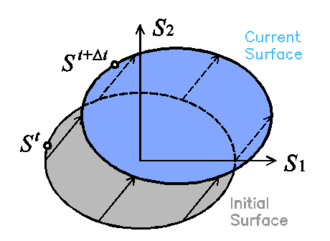Figure 1: Translation of the yield surface result of kinematic hardening

This translation is driven by the kinematic hardening internal variable ${\textstyle \alpha _{ij}}$ which, in a general case, varies proportionally to the plastic strain of the material point (, ). There are several laws that define the evolution of this parameter. Current work uses a non-linear kinematic hardening law, which can be written as:

 ${\dot {\alpha }}_{ij}=c_{k}{\dot {E}}_{ij}^{P}-d_{k}\alpha _{ij}{\dot {p}}$
(10)

Where ${\textstyle c_{k}}$ and ${\textstyle d_{k}}$ are material constants, ${\textstyle E_{ij}^{p}}$ is the plastic strain, and ${\textstyle {\dot {p}}}$ is the increment of accumulative plastic strain, which can be computed as: ${\textstyle {\dot {p}}={\sqrt {2/3\cdot {\dot {E}}_{ij}^{p}:{\dot {E}}_{kl}^{p}}}}$. Note that the 2/3 is valid in case of using Von-Mises as the actual yield surface. In other cases, this value should be modified.

### 3.2.4 Isotropic Hardening

Isotropic hardening provides an expansion or a contraction of the yield surface. The expansion corresponds to hardening and the contraction to a softening behaviour. In figure 2 a two dimensional representation of this effect in the plane ${\textstyle S_{1}-S_{2}}$ is depicted: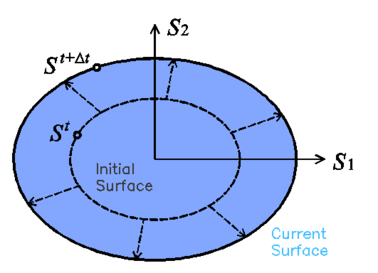Figure 2: Expansion of the yield surface result of isotropic hardening

The evolution of isotropic hardening is controlled by the evolution of the plastic hardening function ${\textstyle K}$, which is often defined by an internal variable ${\textstyle \kappa ^{p}}$. The rate equation for these two functions may be defined, respectively:

 ${\begin{array}{l}{{\dot {K}}={\dot {\lambda }}\cdot H_{k}=h_{k}\cdot {\dot {\kappa }}^{p}}\\{{\dot {\kappa }}^{p}={\dot {\lambda }}\cdot H_{k}={\dot {\lambda }}\cdot \left[h_{k}:{\frac {\partial G}{\partial S}}\right]=h_{k}\cdot {\dot {E}}^{p}}\end{array}}$
(11)

where ${\textstyle k}$ denotes scalar and ${\textstyle {k}}$ states for a tensor function. Depending on the functions defined to characterize these two parameters different solid performances can be obtained. Two new functions valid for characterizing metallic materials are proposed in this work and described in sections 3.3 and 3.4 of this document.

### 3.2.5 Stress-strain relation and consistency factor

Once the material has exceeded its yield threshold, the stress-strain relation is defined by the tangent stiffness matrix. The expression of this matrix, as well as the expression of the plastic consistency parameter can be obtained from the plastic yield criterion and the Prager consistency condition :

 $\left.{\begin{array}{l}{\begin{array}{l}{F(S_{ij},\alpha ^{p},\theta )=f(S_{ij}-\alpha _{ij})-K(S_{ij},\kappa ^{p},\theta )=0}\\{}\end{array}}\\{{\dot {F}}={\frac {\partial F}{\partial S}}{\dot {S}}+{\frac {\partial F}{\partial \alpha }}{\dot {\alpha }}+{\frac {\partial F}{\partial K}}{\dot {K}}=0}\end{array}}\quad \right\}\;{\frac {\partial F}{\partial S}}{\dot {S}}+{\frac {\partial F}{\partial \alpha }}{\dot {\alpha }}-{\dot {K}}=0$
(12)

Using previous expressions, it is possible to rewrite equation 12 as:

 ${\frac {\partial F}{\partial S}}:C:\left({\dot {E}}-{\dot {E}}^{p}\right)+{\frac {\partial F}{\partial \alpha }}:\left(c_{k}{\dot {E}}_{ij}^{P}-d_{k}\alpha _{ij}{\dot {p}}\right)-h_{k}\left(h_{k}:{\dot {E}}^{p}\right)=0$
(13)

From this expression it is possible to obtain the consistency factor using equation 9.

 ${\frac {\partial F}{\partial S}}:C:{\dot {E}}-{\frac {\partial F}{\partial \alpha }}:\left(d_{k}\alpha _{ij}{\dot {p}}\right)-{\dot {\lambda }}\cdot \left[{\frac {\partial F}{\partial S}}:C:{\frac {\partial G}{\partial S}}-c_{k}{\frac {\partial F}{\partial \alpha }}:{\frac {\partial G}{\partial S}}+h_{k}\cdot h_{k}:{\frac {\partial G}{\partial S}}\right]=0$
(14)

Therefore:

 ${\dot {\lambda }}={\frac {{\frac {\partial F}{\partial S}}:C:{\dot {E}}-{\frac {\partial F}{\partial \alpha }}:\left(d_{k}\alpha _{ij}{\dot {p}}\right)}{{\frac {\partial F}{\partial S}}:C:{\frac {\partial G}{\partial S}}-c_{k}{\frac {\partial F}{\partial \alpha }}:{\frac {\partial G}{\partial S}}+h_{k}\cdot h_{k}:{\frac {\partial G}{\partial S}}}}$
(15)

The tangent stiffness tensor relates the total strain rate to the stress rate:

 ${\dot {S}}=C^{T}:{\dot {E}}$
(16)

Finally, the expression of the tangent stiffness matrix can be obtained from the consistency factor:

 $C^{t}=C-{\frac {\left[C:{\frac {\partial G}{\partial S}}\right]\otimes \left[{\frac {\partial F}{\partial S}}:C\right]}{{\frac {\partial F}{\partial S}}:C:{\frac {\partial G}{\partial S}}-c_{k}{\frac {\partial F}{\partial \alpha }}:{\frac {\partial G}{\partial S}}+h_{k}\cdot h_{k}:{\frac {\partial G}{\partial S}}}}$
(17)

It has to be noted that expression 17 has been obtained disregarding the non-linear term of kinematic hardening. Despite having a first approximation of the analytical expression that provides the tangent stiffness tensor, in many occasions the calculation of the partial derivatives of the yield and potential functions is not straightforward. In those cases, a numerical derivation can be performed. This procedure, although expensive, provides an accurate approximation that improves the global convergence of the problem. An efficient procedure to conduct this numerical derivation, as well as the advantages obtained with it, are shown in . A detailed description of the integration procedure of the constitutive equations can be found in references  and .

## 3.3 New isotropic hardening law

Equation 11 allow the incorporation of different hardening laws to describe the material performance. In the Barcelona model defined in , the laws defined are driven by the fracture energy of the material. This work presents a new law, specially developed for steel materials, that has been designed to reproduce their hardening and softening performance under monotonic and cyclic loading conditions. This law also depends on the fracture energy of the material.

### 3.3.1 Fracture Energy

Classical fracture mechanics defines the fracture energy of a material as the energy that has to be dissipated to open a fracture in a unitary area of the material. This energy is defined as:

 $G_{f}={\frac {W_{f}}{A_{f}}}$
(18)

where ${\textstyle W_{f}}$ is the energy dissipated by the fracture at the end of the process, and ${\textstyle A_{f}}$ is the area of the surface fractured. The total fracture energy dissipated, ${\textstyle W_{f}}$, in the fracture process can be used to define a fracture energy by unit volume, ${\textstyle g_{f}}$, required in a continuum mechanics formulation:

 $W_{f}=G_{f}\cdot A_{f}\equiv \int _{V_{f}}g_{f}dV$
(19)

This last equation allows establishing the relation between the fracture energy defined as a material property, ${\textstyle G_{f}}$, and the maximum energy per unit volume:

 $g_{f}={\frac {W_{f}}{V_{f}}}={\frac {W_{f}}{A_{f}\cdot l_{f}}}={\frac {G_{f}}{l_{f}}}$
(20)

Thus, the fracture energy per unit volume is obtained as the fracture energy of the material divided by the fracture length. This fracture length corresponds to the distance, perpendicular to the fracture area, in which this fracture propagates.

In a real section, this length tends to be infinitesimal. However, in a finite element simulation, in which continuum mechanics is applied to a discrete medium, this length corresponds to the smallest value in which the structure is discretized: the length represented by a gauss point.

Therefore, in order to have a finite element formulation consistent and mesh independent, it is necessary to define the hardening law in function of the fracture energy per unit volume (, , ). This value is obtained from the fracture energy of the material, ${\textstyle G_{f}}$, and the size of the finite element in which the structure is discretized.

### 3.3.2 Hardening Function and Hardening Internal Variable

The hardening function defines the stress of the material when it is in the non-linear range. There are many possible definitions that can be used for this function fulfilling equation 11. Among them, the use of a function that describes the evolution of an equivalent uniaxial stress state, like the one shown in figure 3, is proposed here.

This equivalent stress state shown in figure 3 has been defined to match the uniaxial stress evolution described by most metallic materials. After reaching the yield stress, the curve is divided in two different regions. The first region is defined by curve fitting from a given set of equivalent stress-equivalent strain points. The curve used to fit the points is a polynomial of any given order defined using the least squares method. The data given to define this region is expected to provide an increasing function, in order to obtain a good performance of the formulation when conducting a cyclic analysis.Figure 3: Evolution of the equivalent stress

The second region is defined with an exponential function to simulate softening. The function starts with a null slope that becomes negative as the equivalent plastic strains increase. The exact geometry of this last region depends on the fracture energy of the material. The adjustment of this exponential softening to experimental results is usually very difficult, as the stress drop is very fast and experimental tests cannot capture it. Therefore, its definition will be done just to obtain a more or less steep slope. The selection of an exponential function instead of a straight line or a polynomial is made because the exponential function provides a response that facilitates the numerical convergence.

It has to be noted that the initial plateau that is usually found in monotonic stress-strain graphs of carbon steels is not represented in the stress evolution proposed in this model and, therefore, it is not shown in figure 3. This is because the definition of this region will lead to inaccurate results when performing cyclic simulations of the material.

The hardening internal variable, ${\textstyle \kappa ^{p}}$, accounts for the evolution of the plastic hardening function, ${\textstyle K}$. In current formulation ${\textstyle \kappa ^{p}}$ is defined as a normalized scalar parameter that takes into account the amount of volumetric fracture energy dissipated by the material in the actual strain-stress state. This is:

 $\kappa ^{p}={\frac {1}{g_{f}}}\int _{t=0}^{t}S:{\dot {E}}^{p}dt$
(21)

Figure 4 shows shaded in green the volumetric fracture energy required by a uniaxial material, for a given plastic strain ${\textstyle E^{p}}$. The hardening internal variable defined in 21 is calculated normalizing this fracture energy by the total fracture energy of the system, ${\textstyle g_{f}}$, which corresponds to the total area below the curve ${\textstyle S^{eq}(E^{p})}$, shaded with grey lines.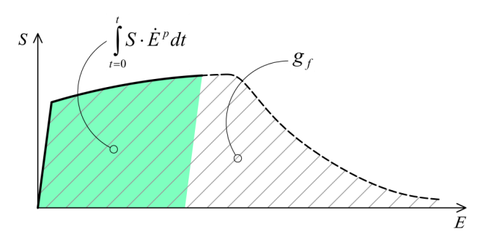Figure 4: Representation of the volumetric fracture energy of a metallic material

Using the definition of the hardening internal variable defined in equation 21, it is possible to define the expression of the hardening function as:

 $K=S^{eq}(\kappa ^{p})$
(22)

It can be easily proven that the hardening function and internal variable defined in equations 21 and 22 fulfill the rate equations 11. The ${\textstyle h_{k}}$ and ${\textstyle h_{k}}$ functions defined in expression 11 become:

 ${\begin{array}{l}{h_{k}={\frac {\partial S^{eq}}{\partial \kappa ^{p}}}}\\{h_{k}={\frac {S}{g_{t}}}}\end{array}}$
(23)

### 3.3.3 Expressions of the hardening function

In this section the exact numerical expressions used to define the new hardening law presented in this work are provided. This law is characterized with two different functions, each one defining the evolution of the equivalent stress in each region in which the equivalent stress performance is divided (see figure 3).

## Region 1: Curve fitting with polynomial

The first region is characterized with a polynomial defined by curve fitting from a given experimental data. Among the different available methods that can be used to define this polynomial, here the use of the least squares method is proposed due to its simplicity, computational cost, and good performance provided. The resulting relation between the stress and plastic strain in this region is:

 $S^{eq}(E^{p})=a_{0}+\sum _{i=1}^{N}a_{i}\cdot \left(E^{p}\right)^{i}$
(24)

with ${\textstyle N}$ the order of the polynomial.

The volume fracture energy that is dissipated in this region can be obtained calculating the area below the ${\textstyle S^{eq}-E^{p}}$ graph. This provides the following value:

 $g_{t1}=\sum _{i=1}^{N+1}{\frac {a_{i}}{i}}\left(\left(E_{2}^{p}\right)^{i}-\left(E_{1}^{p}\right)^{i}\right)$
(25)

being ${\textstyle E_{1}^{p}}$ and ${\textstyle E_{2}^{p}}$ the initial and final plastic strain values, respectively, that delimit the polynomial function region.

Although the equivalent plastic stress should depend on the plastic internal variable ${\textstyle \kappa ^{p}}$, in a cyclic simulation with isotropic hardening this approach will produce hysteresis loops with increasing stress amplitude (for a fixed strain amplitude). For this reason, current formulation calculates the equivalent plastic stress using the value of the equivalent plastic strain, which is obtained as:

 $E_{eq}^{p}={\frac {S:E^{p}}{f(S)}}$
(26)

with ${\textstyle f(S)}$ defined by the yield surface used to simulate the material, as it is shown in equation 8.

Finally, the derivative of the hardening function can be calculated with the following expression:

 ${\frac {dS^{eq}}{d\kappa ^{p}}}={\frac {dS^{eq}}{dE^{p}}}\cdot {\frac {dE^{p}}{d\kappa ^{p}}}=g_{t}\cdot {\frac {\sum _{i=1}^{N}(i-1)\cdot a_{i-1}\left(E^{p}\right)^{i-2}}{\sum _{i=1}^{N}a_{i-1}\left(E^{p}\right)^{i-1}}}$
(27)

Expression 27 is valid for values of ${\textstyle \kappa ^{p}}$ that are comprehended between ${\textstyle \kappa _{1}^{p}=0}$ and ${\textstyle \kappa _{2}^{p}={g_{t1}{\mathord {\left/{}\right.}}g_{t}}}$. The value of the upper limit of the internal variable shows that it is necessary to define a value for the volumetric fracture energy of the material larger than ${\textstyle g_{t1}}$. If the value defined is lower, the material will not be able to reach its ultimate stress as this will imply having a fracture internal variable larger than ${\textstyle 1.0}$.

## Region 2: Exponential softening

When the plastic internal variable reaches the volumetric plastic energy available for the first region, ${\textstyle \kappa ^{p}=\kappa _{2}^{p}}$, exponential softening starts in region two. The function that defines this new region is defined with the following parameters:

1. The initial equivalent stress value is defined by the equivalent stress reached in the first region (${\textstyle S_{2}^{eq}}$). This value can be the one defined in the material characterization or can be a lower value if there has been some plastic energy dissipation in a cyclic process. In this last case, the stress value has to be obtained from previous region.
2. The initial slope of the function is zero.
3. The volumetric fracture energy dissipated in this region is the remaining energy in the material:
4.  $g_{t2}=g_{t}-g_{t1}$

With these considerations in mind, the resultant equation that relates the equivalent stress with the plastic strain is:

 $S^{eq}(E^{p})=S_{2}^{eq}\cdot \left[2\cdot e^{-b\cdot \left(E^{p}-E_{2}^{p}\right)}-e^{-2b\cdot \left(E^{p}-E_{2}^{p}\right)}\right]$
(28)

where ${\textstyle b={\frac {3\cdot S_{2}^{eq}}{2\cdot g_{t2}}}}$

The expression of the equivalent stress as a function of the hardening variable is obtained combining equations 28 and 21, resulting:

 $S^{eq}(\kappa ^{p})=S_{2}^{eq}\cdot \chi \cdot \left(2-\chi \right)$
(29)

being, ${\textstyle \chi ={\sqrt {{\frac {(\kappa ^{p}-\kappa _{2}^{p})\cdot 2b\cdot g_{t}}{S_{2}^{eq}}}+1}}}$

And the derivative of the hardening function is:

 ${\frac {dS^{eq}}{d\kappa ^{p}}}=2b\cdot g_{t}\cdot \left({\frac {1}{\chi }}-1\right)$
(30)

### 3.3.4 Validation of the proposed formulation

In the following, the results obtained from several simulations conducted to validate the formulation previously presented are included. This validation has been done comparing the numerical results with some of the experimental results obtained in the framework of the Ultra Low Cycle Fatigue Project .

#### 3.3.4.1 Description of the experimental tests

Monotonic and cyclic tests were performed in a close-loop servo-hydraulic machine, INSTRON 8801, rated to 100 kN. The tests were performed at room-temperature in air. The fatigue tests were conducted under constant strain amplitudes and with a frequency adjusted to result an average strain rate of 0.008s${\textstyle {}^{-1}}$. The longitudinal strain was measured using a clip gauge with limit displacements of ${\textstyle \pm }$2.5 mm with a gauge length of 12.5 mm (INSTRON 2620-602). This extensometer was also used in two monotonic tensile tests allowing the registration of the longitudinal strains until approximately 17%

All tested specimens were machined according the longitudinal direction of 6 inch pipes made of X52 steel. The dimensions of the specimens are in accordance with the ASTM E606 standard, as illustrated in figure 5. The shown specimen corresponds to the SP series. The side faces of the specimens were milled and finished in order to remove the circumferential pipe curvature as well as surface imperfections. In order to achieve larger strain values in the specimen some of them where machined in order to reduce their section in their middle. This is the case of the OH specimen, shown in figure 6, in which the geometry is modified with an oval hole in its center. The experimental results obtained for this specimen have been also used to validate the model performance.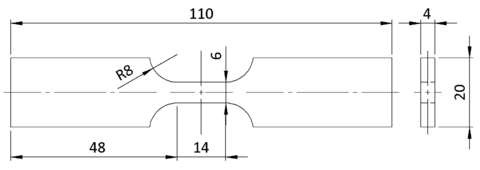Figure 5: Dimensions, in millimeters, of the SP specimen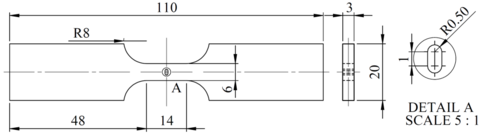Figure 6: Dimensions, in millimeters, of the OH specimen

#### 3.3.4.2 Description of the numerical models

Two different numerical models have been defined, one for each experimental specimen. Figure 7 shows the meshes of both models. The SP model is made with 1608 quadratic hexahedral elements and 8839 nodes. It has three elements along its thickness and 563 elements in the face shown in figure 7 (YZ). The OH model has 3080 quadratic hexahedral elements and 15460 nodes. It has five elements along the thickness and 616 elements in the YZ face. This second model requires nearly double the number of elements because the element size has to be significantly smaller around the hole for its correct simulation.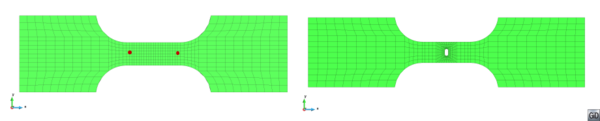Figure 7: Mesh defined for the SP and OH numerical models. The SP model has marked, with red dots, the points used to calculate the equivalent strain applied.

Regarding boundary conditions, the left border of the model has the displacement fixed to zero in all its directions, while the right border is moved with an imposed displacement in the longitudinal direction of the sample. The reaction force is obtained as a result of the numerical analysis.

All samples analyzed are defined with the same plastic material, defined with an associated plasticity and Von-Mises as yield law. The material properties are obtained with the calibration process that is described in the following section. All the material properties required by the model are displayed in table 1. A parameter of this table that may seem surprising is the value of the yield stress, which is lower than the one expected for an X52 steel. This value is required because, if the yield stress is defined with a higher value, the kinematic hardening parameters lead to stress values too high in the hysteresis cycles. As the purpose of the model is the simulation of ULCF, which requires large plastic strain values, the requirement of defining a lower yield stress is not considered a major drawback.

 Young Modulus 180 GPa Poisson Ratio 0.3 Yield Stress (${\textstyle \sigma _{Y}^{eq}}$) 240 MPa Plastic Strain threshold (${\textstyle E_{2}^{p}}$) 13 % ${\textstyle c_{1}}$ kinematic hardening param. 60 GPa ${\textstyle d_{1}}$ kinematic hardening param. 280 Fracture Energy (${\textstyle G_{f}}$) 1.9 MN * m/m${\textstyle {}^{2}}$

The hardening region is defined with a polynomial of order five which is computed with the least squares method using the available experimental data. The constants of this polynomial, following the notation shown in equation 24, are shown in table 2. In order to define the polynomial constants, it has been necessary to take into account that the effects of the kinematic and the isotropic hardening laws are coupled. This implies that the definition of the first region of isotropic hardening cannot be obtained from the experimental curve straightforward, as this curve does not take into account the displacement of the yield surface due to the kinematic hardening law.

 ${\textstyle a_{0}}$ 2.4e+08 ${\textstyle a_{1}}$ 8.0084e+07 ${\textstyle a_{2}}$ -1.1143e+08 ${\textstyle a_{3}}$ 8.74e+07 ${\textstyle a_{4}}$ -3.0507e+07 ${\textstyle a_{5}}$ 3.9073e+06

#### 3.3.4.3 Calibration of the numerical model

The material data previously exposed has been obtained by model calibration. This is, adjusting the different parameters required by the model to obtain a good fitting with one of the experimental results available. For the current case, the experimental results considered are those of the specimen loaded with an imposed strain range of 2.75%

The results used to conduct the material calibration are the equivalent stress-equivalent strain graphs obtained from the experimental test. The stress is computed as the total force applied to the specimen divided by the area of the cross section. The strains are computed dividing the measured displacement of the clipped gauge by the length of the gauge. In the numerical model these two parameters were calculated following the same procedure, using the red nodes depicted in figure 7.

The initial material parameters used in the calibration process are ones commonly found in literature and the polynomial constants correspond to the ones obtained by curve fitting of the experimental results, ignoring the effect of kinematic hardening. Based on the strain-stress graph obtained with these first values, the different parameters shown in table 1 and table 2 have been modified until obtaining a numerical result that fits the experimental results.

Figure 8 shows the stress-strain graph provided by the two experimental samples tested and by the numerical model, which uses the material parameters obtained from the calibration process and described in table 1 and in table 2. As it can be seen, the agreement in the cyclic behaviour of the numerical and experimental samples is rather good. This agreement is not achieved in the first loading cycle, as the model developed is not prepared to reproduce the initial plateau defined by the material. However, this disagreement is not considered relevant, as the model has been developed thinking of the cyclic behaviour and the evolution of plastic response for larger plastic strain values, found beyond this initial plateau.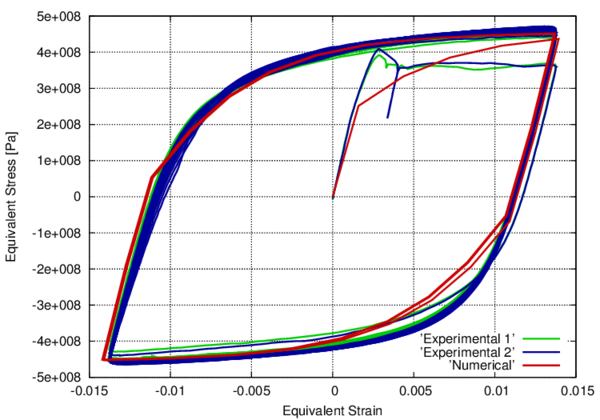Figure 8: Stress-strain graphs for the SP sample with an applied deformation range of 2.75%
The fitting of the stress-strain graph allows defining all material parameters except the fracture energy of the material and the equivalent plastic strain value at which softening is expected to start (${\textstyle E_{2}^{p}}$). These two parameters have been defined to match the number of cycles that can be applied to the specimen before it fails. In the experimental campaign, the first specimen failed after 150 cycles, and the second specimen failed after 103 cycles. With these results, an initial value of ${\textstyle E_{2}^{p}=13\%}$, as the maximum hardening strain has been defined. Afterwards, the parameter of the material fracture energy, ${\textstyle G_{f}}$, is modified until finding a value that predicts the failure of the numerical test after 128 cycles. These values are shown in table 1 and used in the numerical simulations. Material failure is found when some gauss points reach a plastic damage value close to one. At this stage the numerical analysis cannot reach convergence and the specimen can be considered to be completely broken. In current simulation, the convergence is lost for a ${\textstyle \kappa ^{p}}$ value close to 0.9, as it is shown in figure 9, where the value of plastic damage is represented in the last tensile and compressive stages.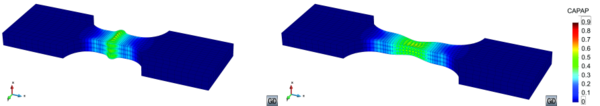Figure 9: Values of $\kappa ^{p}$ for the SP sample with an applied deformation range of 2.75%, when applying the maximum tensile and compression strain before ULCF failure. Deformation is magnified by 20.

The mechanical performance of the numerical specimen along the simulation is shown in figure 10, where the stress-strain loops for different cycles are represented. This picture shows that the effect of material softening in the specimen consists of a reduction of the equivalent stress obtained as the number of cycles applied to the specimen increase. The figure shows that softening has already started in cycle 60, although the difference between the first loop and loop 60 is very small. In further cycles the effect of softening becomes more visible, until material failure in cycle 128, where the reduction of equivalent stress is close to a 20% Figure 10 also shows that the strain amplitude increases with the number of cycles applied to the specimen. This increase is obtained because equivalent strain is calculated as the relative displacement of two given nodes (red dots in figure 7), divided by the original length between both nodes, and does not take into account that it is modified due to the plastic deformations existing in the whole specimen.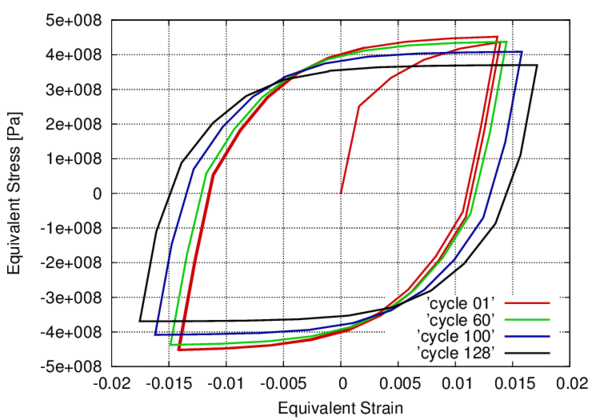Figure 10: Evolution of the stress strain graph for different cycles of the SP numerical analysis

The results shown for the calibration test prove that the constitutive law proposed can be adjusted to any given stress-strain law by adjusting properly the material parameters. The calibration model has also been used to show the numerical and mechanical performance of the formulation developed. The prediction capacity of the model will be shown in further simulations, in which the material parameters are fixed and are used to analyze different specimens and load cases.

#### 3.3.4.4 Validation of the developed theory

After having calibrated the material parameters, the rest of experimental tests available have been simulated in order to compare the ULCF failure prediction made by the numerical model with the results obtained from the experimental campaign. The constitutive model proposed in this work will succeed if it is capable of representing accurately the equivalent stress-strain graphs for the different deformation ranges tested experimentally and, even more important, if it is capable of predicting the number of cycles that can be applied to the specimen before its failure.

Figure 11 shows the stress-strain graph corresponding to the experimental and numerical sample with an applied equivalent strain of 4% In order to achieve this strain value, and to avoid the buckling of the specimen, the experimental test is conducted only on the positive strain region and with an antibuckling device. As can be seen, the experimental and the numerical results are in good agreement of the cyclic region of the curve. So, with this example it is proved that the model is capable of reproducing large strain values and non-symmetric cyclic patterns.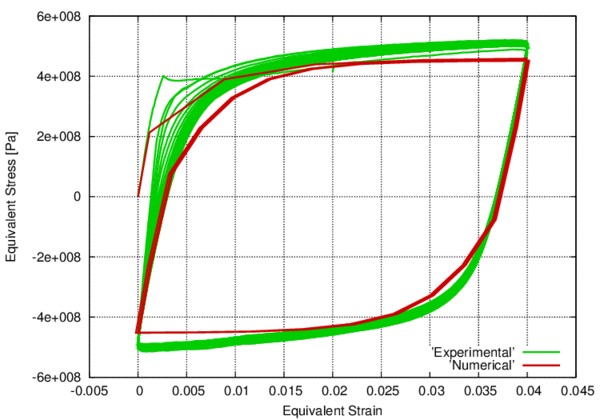Figure 11: Stress-strain graphs for the SP sample with an applied deformation range of 4.00%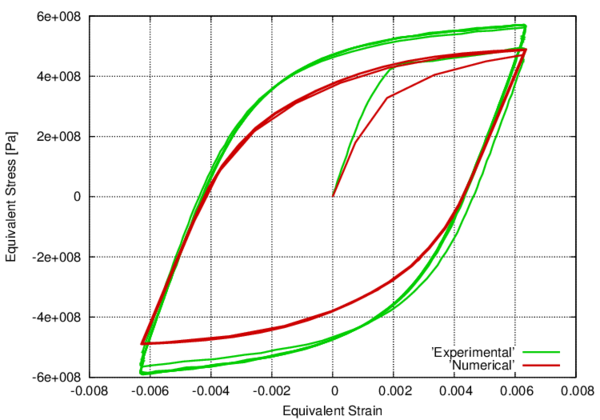Figure 12: Stress-strain graphs for the OH sample with an applied deformation range of 1.20%

Finally, figure 12 compares the stress-strain experimental-numerical results obtained for one of the OH samples. The stress value for the OH samples is computed with the same procedure used for the SP samples, dividing the total force applied by the area of the cross section where the notch is found; and the strain value is obtained dividing with the gauge length described in section 3.3.4.1.

In this case, the numerical result prediction falls a bit shorter in terms of equivalent stress when compared to the experimental test. However, a closer look to the experimental curve shows that in the first loading branch the experimental and numerical tests match perfectly, and it is in further cycles that the experimental test provides larger equivalent stress values. This difference between cycles in the experimental test may be due to some sort of hardening around the notch, which cannot be captured by the proposed model. Despite this difference, results are considered similar enough to validate the material parameters defined in the numerical model calibration.

Once having proved that the developed formulation is capable of reproducing quite accurately the mechanical response obtained with the experimental samples, the following step is to verify if the formulation is capable of predicting the failure of the specimens due to ULCF. This validation is performed counting the number of cycles that can be applied to the numerical model before its failure. The numerical model is considered to have failed when the convergence of the analysis is lost. This occurs when some gauss points reach a ${\textstyle \kappa ^{p}}$ value close to 1,0. The number of cycles applied to the numerical model are compared with the cycles obtained in the experimental campaign.

Figure 13 shows the results obtained for the SP samples. Results with reversion strain factor of –1 and of 0 are plotted together because the reversion factor does not play a significant role in the material response to ULCF. This figure shows that the number of cycles to failure predicted by the numerical simulation are in very good agreement with the number of cycles obtained in the experimental campaign. The only value that is not contained between the experimental results is the one corresponding to an applied strain range of 3.5% However, the value provided by the numerical simulation looks more coherent than the one obtained with the experimental test, as the number of cycles obtained in the experimental test is larger than the one obtained for an applied strain range of 2.75%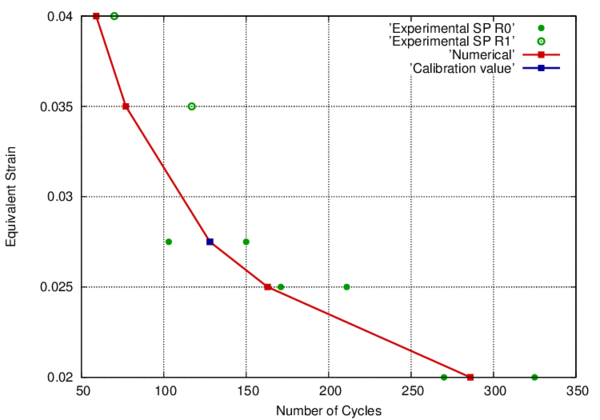Figure 13: ULCF failure prediction for SP samples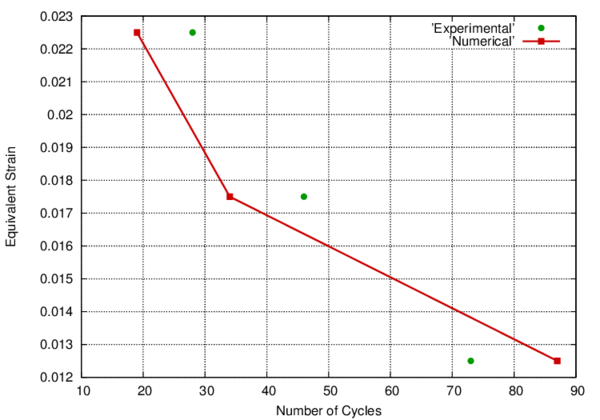Figure 14: ULCF failure prediction for OH samples

The results obtained for the OH samples are shown in figure 14. For these samples the experimental test was conducted in just one specimen for each strain value, therefore there is no possibility to know the scatter expected in the experimental tests. However, the number of cycles predicted by the formulation is, for all strains, in the same order of magnitude than the experimental results obtained. Therefore, it can be concluded that the formulation is, again, capable of predicting accurately the ULCF failure of the OH specimens.

It is important to remark that the material properties used for all numerical simulations are exactly the same. Therefore, the variation in the prediction of the number of cycles that can be applied to any of the specimens considered is the result of the energy dissipated in each case. The agreement obtained in all cases, independently of the reversion factor or the stress concentrations due to the existing hole (OH sample) allows considering the approach used to characterize ULCF failure an excellent option. Moreover the formulation allows conducting simulations in which the cycles can be non-regular, with varying amplitude and frequency, in which there can be sustained monotonic loads between cycles or, in general, in which the load applied is not a regular one. This capability is not offered by any other formulation available.

## 3.4 Extension of the new isotropic hardening law for large problems

### 3.4.1 Hardening Function and Hardening Internal Variable

The constitutive law presented in section 3.3 has been modified in order to ensure faster convergence in the numerical model and therefore make feasible the application of the model on large problems.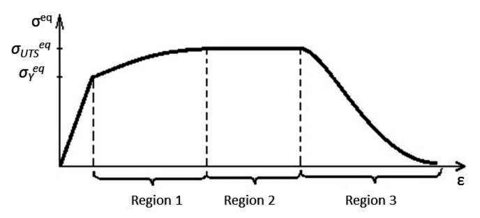Figure 15: Evolution of the equivalent plastic stress

The equivalent stress state shown in figure 15 is different from the one presented in section 3.3 in the sense that the region obtained thru curve fitting is divided into two: a smaller region that is still dependent on curve fitting of experimental points and a linear region, with slope and extension defined by user. That helps ensure a fast integration of the stresses in the constitutive model by converging to the same numerical tolerance in less iterations. The first region is, therefore, defined by curve fitting from a given set of equivalent stress-equivalent strain points. The curve used to fit the points is a polynomial of any user given order, defined using the least squares method. The data given to define this region is expected to provide an increasing function, in order to obtain a good performance of the formulation when performing cyclic analysis.

The second region, as mentioned above, is defined as a linear curve. This region is incorporated to facilitate the convergence of the problem. If region 1 and 2 were to be simulated with just one polynomial, the difference in slope between the beginning of the curve and the end of it would make it very difficult to ensure that the slope of the polynomial is always positive so that the solution does not converge to a local minimum. Generally speaking, in the case of ULCF and LCF nearly 80% or more of the internal energy of the material is spent between regions 1 and 2.

The third region is defined with the same exponential function to simulate softening as in section 3.3. The function starts with a null slope that becomes negative as the equivalent plastic strains increase. The exact geometry of this last region depends on the fracture energy of the material (figure 16).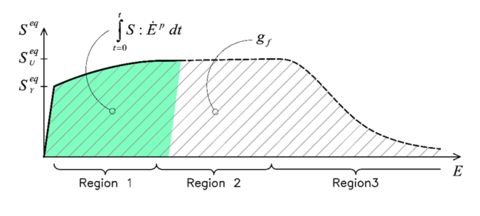Figure 16: Representation of the volumetric fracture energy of a metallic material

### 3.4.2 Expressions of the hardening function

In this section, the exact numerical expressions used to define the new hardening law are discussed. This law is an evolved version of the one presented in section 3.3, as said before. The region described by curve fitting in the above reference has been divided into two different regions (Region 1 and Region 2 in figure 15). This has been done in order to optimize the constitutive law for converging to the same tolerance in fewer iterations and making feasible the large scale simulations presented in sections 3.5 and 3.6 of this chapter. The length of the curve fitting region has been limited to a user defined value and past this value of the plastic strain a linear curve has been defined with a user defined slope.

## Region 1: Curve fitting with polynomial

The first region is characterized with a polynomial defined by curved fitting from a given experimental data. The exact mathematical definition for this region can be found in 3.3.3 .

Expression 27 is valid for values of ${\textstyle \kappa ^{p}}$ that are comprehended between ${\textstyle 0}$ and ${\textstyle \kappa _{1}^{p}={g_{t1}{\mathord {\left/{}\right.}}g_{t}}}$.

## Region 2: Linear curve

When the equivalent plastic strain, as calculated with equation 26, equals the value of equivalent plastic strain at which region 2 is to begin,${\textstyle E_{eq}^{p}=E_{1}^{p}}$, where ${\textstyle E_{1}^{p}}$is user defined, the threshold function is obtained taking into account the following considerations:

1. The initial equivalent stress value is defined by the equivalent stress reached at the end of the first region (${\textstyle S_{1}^{eq}(E_{1}^{p})=a_{0}+\sum _{i=1}^{N}a_{i}\cdot \left(E_{1}^{p}\right)^{i}}$).
2. The slope of the function is user defined: ${\textstyle u={\frac {S_{2}^{eq}-S_{1}^{eq}}{E_{2}^{p}-E_{1}^{p}}}}$.
3. The volumetric fracture energy dissipated in this region is ${\textstyle g_{t2}=(S_{1}^{eq}+S_{2}^{eq})\cdot (E_{2}^{p}-E_{1}^{p})\cdot 0.5}$.

With these considerations in mind, the resulting equation that relates the equivalent stress with the plastic strain is:

 $S^{eq}(E^{p})=S_{1}^{eq}+u\cdot (E_{}^{p}-E_{1}^{p})$
(31)

The expression of the equivalent stress as a function of the hardening variable is obtained combining equation 31 and 21:

 $S^{eq}(\kappa ^{p})={\sqrt {\left(S_{1}^{eq}\right)^{2}+2\cdot u\cdot g_{t}\cdot (\kappa ^{p}-\kappa _{1}^{p})}}$
(32)

Expression 32 is valid for values of ${\textstyle \kappa ^{p}}$ that are comprehended between ${\textstyle \kappa _{1}^{p}={g_{t1}{\mathord {\left/{}\right.}}g_{t}}}$ and ${\textstyle \kappa _{2}^{p}={(g_{t1}+g_{t2}){\mathord {\left/{}\right.}}g_{t}}}$. The value of the upper limit of the internal variable shows that it is necessary define a value for the volumetric fracture energy of the material larger than ${\textstyle g_{t1}+g_{t2}}$. If the value defined is lower, the material will not be able to reach its ultimate stress as this will imply having a fracture internal variable larger than ${\textstyle 1.0}$.

## Region 3: Exponential softening

When the plastic internal variable reaches the volumetric plastic energy available in the first two regions: ${\textstyle \kappa ^{p}=\kappa _{2}^{p}}$. At this point, isotropic hardening is defined by region three. Its function is obtained with the following parameters:

1. The initial equivalent stress value is defined by the equivalent stress reached at the end of the second region (${\textstyle S_{2}^{eq}}$).
2. The initial slope of the function is zero.
3. The volumetric fracture energy dissipated in this region is the remaining energy in the material: ${\textstyle g_{t3}=g_{t}-g_{t1}-g_{t2}}$

With these considerations in mind, the governing equations are the same as in 3.3. The constitutive model described in Sections 3.2, 3.3 and 3.4 has been implemented in the in-house code PLCd . The code was programmed to allow OpenMP parallelization, which greatly reduced the computational cost of the large scale FE simulations, and makes use of the load advancing strategy proposed in  and  and shown later on in this work in chapter 4.

### 3.4.3 Material calibration

The material characteristics for the numerical simulations will be obtained by conducting a calibration analysis on small scale specimens. The hardening - softening law presented in section 3.4 requires of the following material parameters:

1. ${\textstyle \varepsilon }$${\textstyle {}_{p}}$${\textstyle \sigma }$${\textstyle {}_{p}}$ points obtained from uniaxial monotonic tensile tests necessary for curve fitting. They are important for a correct representation of the tendency of the monotonic curve.
2. Kinematic coefficients in accordance with the type of hardening chosen. They are important for the exact adjustment of the monotonic curve and for an accurate description of the hysteresis loop.
3. Equivalent plastic deformation, ${\textstyle E_{1}^{p}}$ , at which the linear region starts. This parameter is important both in the monotonic curve and in the overall cyclic behaviour as it ensures a stable behaviour throughout the fatigue life.
4. Equivalent plastic deformation, ${\textstyle E_{2}^{p}}$ , at which softening starts.
5. Fracture energy G${\textstyle {}_{f}}$ required in the monotonic curve for adjusting the slope of the softening behaviour and in the cyclic behaviour for correctly calibrating the fatigue life of the specimen. When the entire energy is spent the specimen is considered completely fractured.

For the industrial applications shown in this work two different materials have been calibrated, X52 and X60, for the second law, presented in 3.4. The step by step calibration process is presented for the X60 material. Another calibration process has been shown in section 3.3 for the X52 steel using the first version of the constitutive law.

In order to exemplify the calibration process, a smooth X60 specimen was chosen from the experimental program ran by Pereira et al. . This experimental program includes monotonic tests and cyclic tests in the LCF and in the ULCF regime. LCF tests have been conducted both with a reversion factor of -1 and 0, while all the ULCF tests have a reversion factor equal to 0. The geometric characteristics of the specimen are shown in figure 17. This material will be posteriorly used for the large scale simulation of the bent pipe under cyclic loading shown in section 3.5.

The specimen was meshed into 3456 quadratic hexahedral elements with 20 nodes each and 27 integration points, adding to a total of 17165 mesh nodes.

The procedure for the correct calibration of the material starts from the determination of the elastic modulus and of the elastic limit. These two parameters are determined statistically from the force – displacement recordings of both the monotonic and the cyclic tests by monitoring where the linear relation is lost between them. For this simulation an elastic modulus of ${\textstyle E=1.95\times 10^{11}N/m^{2}}$and an elastic limit of ${\textstyle \sigma _{y}=3.80\times 10^{8}N/m^{2}}$were chosen.

 [[ Figure 17: Geometry of the specimen used for calibration

The ${\textstyle \varepsilon }$${\textstyle {}_{p}}$${\textstyle \sigma }$${\textstyle {}_{}}$set of points chosen for this simulation are presented in figure 18 as compared to the stress-strain curve of the small specimen chosen. At this point in the calibration procedure the series of chosen points have to follow the general tendency of the monotonic curve without reaching the same level of stress. The density of the points is recommended to be constant and quite high so that the polynomial interpolation can be effective. For this simulation the points were interpolated by a 5${\textstyle {}^{th}}$ order polynomial function.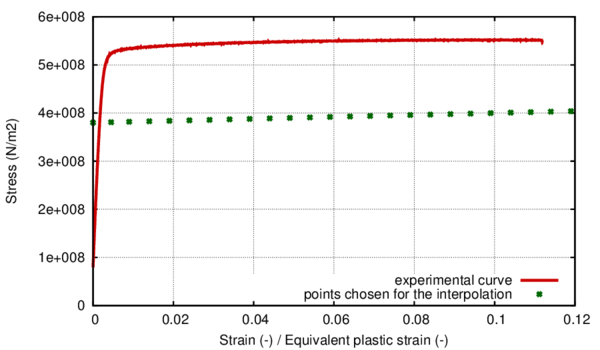Figure 18: Comparison between the stress strain curve for the uniaxial monotonic tensile test and the points chosen for the numerical model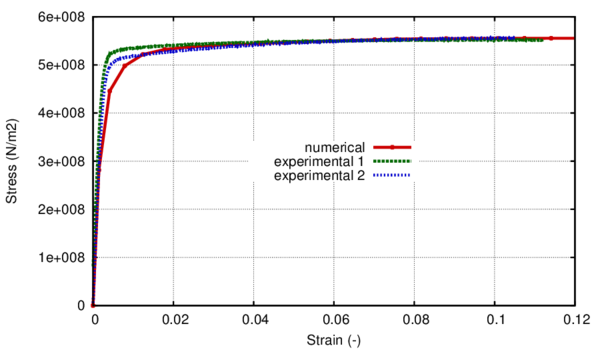Figure 19: Monotonic stress- strain curve. Numerical vs. experimental.

The Armstrong- Frederick kinematic hardening function was used for this simulation. The kinematic coefficients chosen were ${\textstyle k_{1}=6\times 10^{10}}$ and ${\textstyle k_{2}=400}$. In figure 19 the effect of the kinematic hardening on the monotonic curve can be observed. In order to obtain this behaviour a value of 0.1 was used for the ${\textstyle E_{1}^{p}}$ parameter.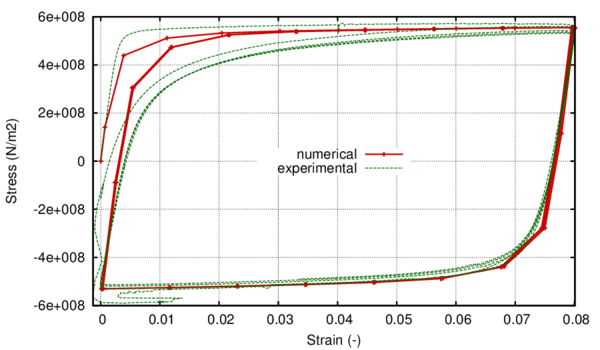Figure 20: Stress-strain hysteresis loop for $\Delta$$\varepsilon$=8% Numerical vs. experimental.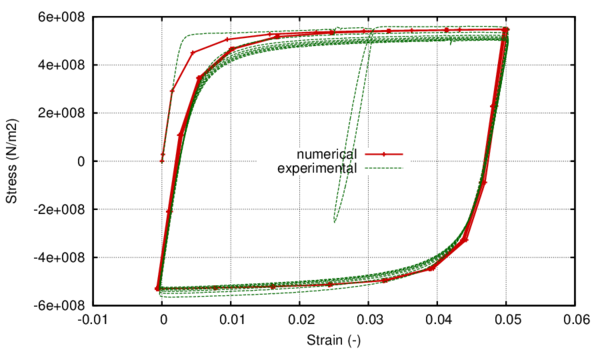Figure 21: Stress-strain hysteresis loop for $\Delta$$\varepsilon$=5% Numerical vs. experimental.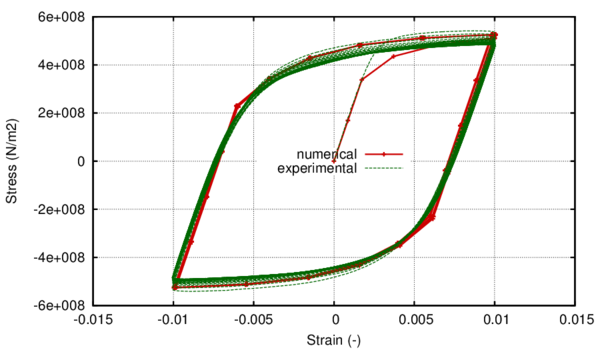Figure 22: Stress-strain hysteresis loop for $\Delta$$\varepsilon$=2% Numerical vs. experimental.

It can be seen that taking into account the kinematic hardening causes the resulting stress-strain curve to elevate until it reaches the experimental monotonic one. Also, the exact shape of the transition zone from linear to nonlinear is determined by the kinematic coefficients.

In figure 21 the numerical hysteresis loop is compared to the experimental one for the ${\textstyle \Delta }$${\textstyle \varepsilon }$=5% case. In choosing the kinematic coefficients a compromise must be made between the accuracy of the monotonic behaviour and of the cyclical one.

Once the kinematic coefficients have been established the next step in the calibration of the material is establishing the equivalent plastic deformation at which softening begins, ${\textstyle E_{2}^{p}}$, and the facture energy.

An experimental result has been chosen for the calibration, the ${\textstyle \Delta }$${\textstyle \varepsilon }$=5% case, that had an experimental fatigue life of 100 cycles. With a value of 13 for the ${\textstyle E_{2}^{p}}$ and a fracture energy of 2.7 x 10${\textstyle {}^{6}}$Nm/m${\textstyle {}^{2}}$ a total fatigue life of 100.35 cycles has been obtained from the numerical simulation. With these values, softening started in the 86${\textstyle {}^{th}}$ cycle, close to the end of the experimental life and a very low amount of energy was left for the softening branch so that it could be spent in a reduced number of cycles.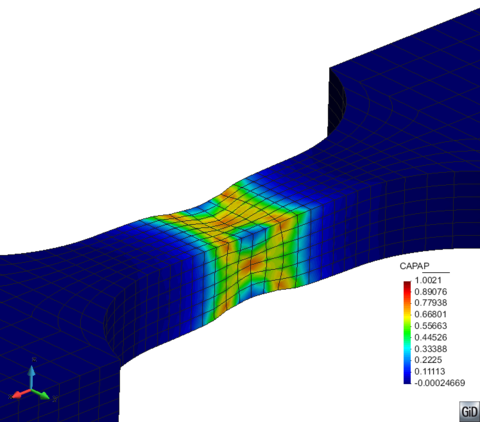Figure 23: Distribution of the normalized plastic dissipation at total fracture

In figure 23 the distribution of the plastic internal variable ${\textstyle \kappa ^{p}}$ can be seen on the deformed shape of the specimen in the last step of the analysis for the calibration case. The null value for the plastic internal variable represents an elastic state in the material, while ${\textstyle \kappa ^{p}=1}$ means the entire fracture energy of the material has been dissipated at that material point. It can be seen that the lateral necking is in accordance to standard metal fracture under uniaxial cyclic loading.

After the adjustment of the ${\textstyle \Delta }$${\textstyle \varepsilon }$=5% case, simulations were ran with the exact same material parameters and with strain amplitudes of 8%(figure 20) and 2% (figure 22). It can be seen that the considered material parameters offer a good aproximation of the hysteresis loop for these strain amplitudes also. The results in terms of life prediction can be seen in figure 24.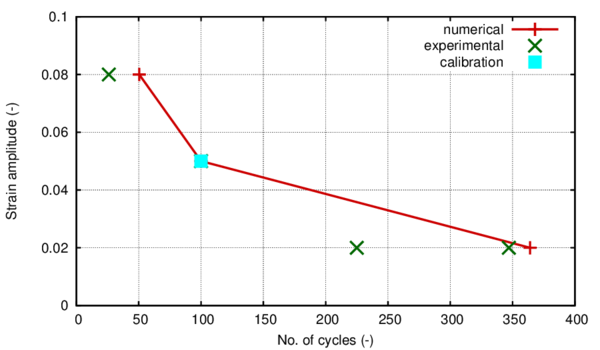Figure 24: Comparison between numerical and experimental fatigue life for different strain amplitudes when calibrating with $\Delta$$\varepsilon$=5%

## 3.5 Large scale validation on a bent pipe under variable cyclic loading

This section presents the results of finite element simulations made on a bent pipe subjected to an in-plane variable cyclic displacement combined with internal pressure. Special emphasis is put on the capacity of the model to illustrate different failure modes depending on the internal pressure applied on the pipe. The results of the numerical analyses will be compared to experimental ones.

### 3.5.1 Geometry of the model

Following the validation of the constitutive model made on small scale specimens, the model is to be applied to large scale numerical simulations of a bent pipe. The geometry of the model, boundary conditions and the sequence of loading are in accordance to the experiment made by Schaffrath et al. .

The specimens consist of a bended middle section (elbow pipe) and a straight pipe section at each end of the elbow. The fillet radius of the elbow pipe is three times the pipe diameter (R=3 x D). For the length of both straight pipe sections a value of five times the diameter (L=5 x D) was used, whereby the influence of the load introduction can be neglected.

Figure 25 shows the general dimensions of the specimen as a function of the diameter of the pipe, while in figure 26 the geometry of the specimen after manufacturing can be observed. For the numerical simulation, a specimen made of X60 steel has been chosen from the experimental program, with a diameter of 406.4 mm and a wall thickness of 9.5 mm. The pipe has an elbow angle of 90${\textstyle {}^{\circ }}$.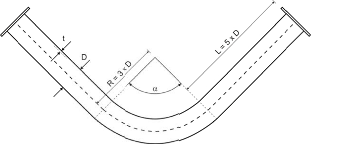Figure 25: Overview of the general dimensions of the specimen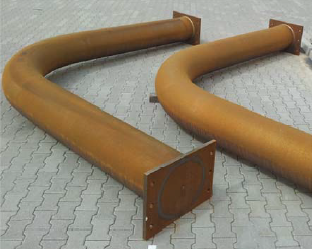Figure 26: Specimen SP1-SP2 (X60, 90${}^{\circ }$)

Figure 27 shows the model used for the numerical simulation as generated with the pre-postprocessor GiD. Following, in figure 28 a view of the mesh is shown. For this simulation quadratic hexahedral elements were used, each with 20 nodes and 27 integration points. The mesh consisted of 42853 elements and 213415 nodes. Three elements were considered in the pipe thickness.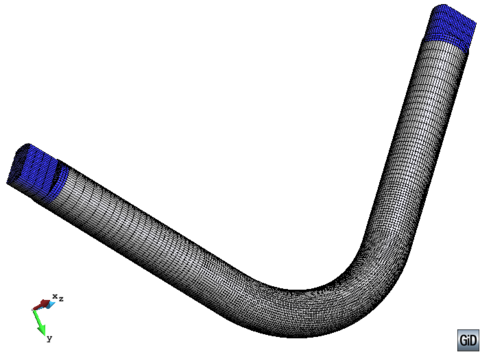Figure 28: Mesh of hexahedral quadratic elements

The loading history is based on the actual load history of the experimental test done by Schaffrath et al. . The loading scheme was decided by the authors in accordance with the ECCS procedure ECCS-Nr. 45-1986 Recommended Testing Procedure for Assessing the Behavior of Structural Steel Elements under Cyclic Loads . For practical reasons it was decided to neglect the mostly small difference between the compressive and tensile yield strain by choosing an average value ${\textstyle e_{y}=(e_{y}^{+}+e_{y}^{-})/2}$ as the reference amplitude. In table 3 the experimental loading sequence is described as a function of ${\textstyle e_{y}}$.The value adopted for this parameter was set by  at ${\textstyle \pm }$82mm.

 Step Amplitude Number of cycles 1 0.25 ${\textstyle e_{y}}$ 1 2 0.50 ${\textstyle e_{y}}$ 1 3 0.75 ${\textstyle e_{y}}$ 1 4 1.00 ${\textstyle e_{y}}$ 1 5 1.50 ${\textstyle e_{y}}$ 3 6 2.00 ${\textstyle e_{y}}$ 3 7 2.50 ${\textstyle e_{y}}$ 3 8 3.00 ${\textstyle e_{y}}$ 3 9 3.50 ${\textstyle e_{y}}$ 3 10 4.00 ${\textstyle e_{y}}$ 3 11 4.40 ${\textstyle e_{y}}$ 27

The entire loading sequence is comprised of 49 cycles with increasing amplitude, 44 of which have amplitudes in the plastic range. The reversion factor of the applied displacement is -1 (figure 29). The pipe is also submitted to internal pressure. First, the pipe is loaded until a level of internal pressure equal to 20 bars. Afterwards, it is submitted to the varying cyclic displacement presented in table 3. The experimental test has shown that the internal pressure also oscillates when the cyclic displacement is applied.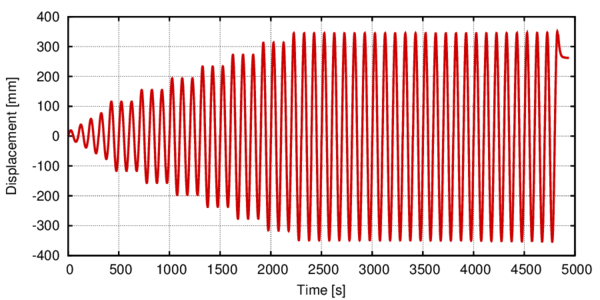Figure 29: Evolution of the applied displacement in the experiment
The boundary conditions of the model were chosen in accordance with the setting of the experiment. One end of the model has its displacement blocked in the x, y and z direction while in the other end the cyclic displacement is applied in the z in-plane direction, as shown in figure 30.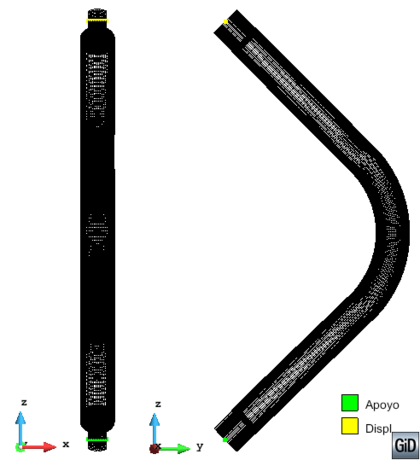Figure 30: Boundary condition for the large scale model

In the numerical simulation the loads have been applied in two stages. First, the internal pressure was applied and, in this stage, one end of the pipe was clamped and on the other end the pipe was only allowed in-plane gliding. The variable displacement was applied in the second stage on the deformed geometry obtained from applying the internal pressure. The movement was restrained in the two directions perpendicular to the in-plane one.

### 3.5.3 Material characteristics

The exact calibration procedure for the X60 material can be seen in Section 3.4.3. In table 4 and table 5 a summary of the material properties as resulting from the calibration can be seen. A polynomial of the 5${\textstyle {}^{th}}$ degree was chosen for the curve fitting zone of the hardening function.

 Coefficient no. 1 ${\textstyle a_{0}}$ 380000000,00 Coefficient no. 2 ${\textstyle a_{1}}$ 326947332,25 Coefficient no. 3 ${\textstyle a_{2}}$ -861244568,93 Coefficient no. 4 ${\textstyle a_{3}}$ 1103673406,83 Coefficient no. 5 ${\textstyle a_{4}}$ -657861660,66 Coefficient no. 6 ${\textstyle a_{5}}$ 147925577,48

 Young Modulus 1.95 10${\textstyle {}^{5}}$ MPa Poisson Modulus 0.30 Elastic Stress (${\textstyle \sigma _{Y}^{eq}}$) 380 MPa Plastic Strain Limit for region 1(${\textstyle E_{1}^{p}}$) 10 % Plastic Strain Softening (${\textstyle E_{2}^{p}}$) 1300 % ${\textstyle c_{1}}$ kinematic hardening 6.0 10${\textstyle {}^{4}}$ MPa ${\textstyle d_{1}}$ kinematic hardening 400 Fracture Energy 2.7 MN * m/m${\textstyle {}^{2}}$

### 3.5.4 Results and discussion

In figure 31 the comparison between the experimental force- displacement curve and the numerical one can be seen. The numerical curve is in very good agreement with the experimental one taking into consideration that the material calibration was done on small scale specimens with different experimental results.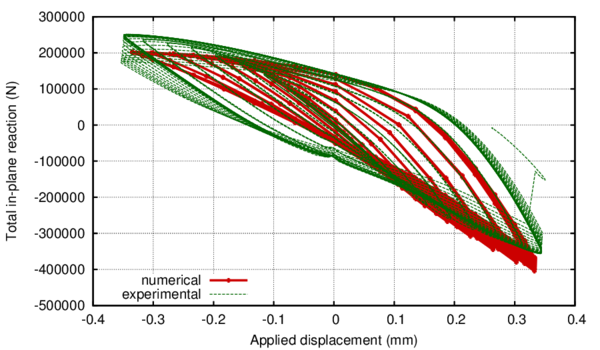Figure 31: Force-displacement curve. Experimental vs. numerical

It can be seen how in compression the constitutive equation tends to underestimate the maximum force level, while in traction the opposite tendency is present. Furthermore, this tendency is more obvious as the displacement increases. This is due to the existence of oscillations of the internal pressure applied in the experiment, caused by applying the cyclic displacement. This oscillation of the internal pressure has not been taken into account in the numerical simulation.

Regarding the fatigue life, the simulation lasted a total of 41.75 plastic cycles as compared to the experimental life of 44 complete cycles. This result also shows a good agreement between the experiment and the numerical simulation.

Figure 32 illustrates the deformed shape of the geometry in the last step of the analysis and presents the distribution of the plastic internal variable of the model. The deformed shape is represented with a scale factor of 2 in order to better reflect the general tendency. Only the central zone of the elbow is shown, as this is the zone where nonlinear effects appear. It can be seen that the failure mode resulting from the numerical simulation is by cross-sectional ovalization with a crack opening in the longitudinal direction of the elbow, at its flank.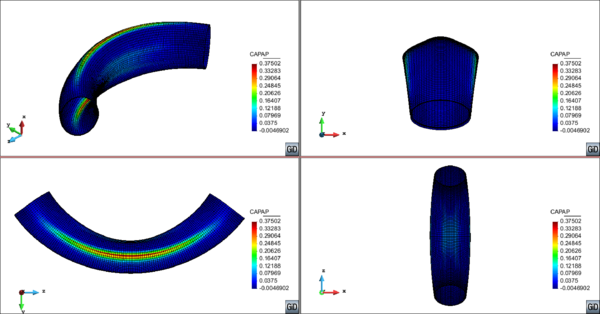Figure 32: Distribution of the plastic internal variable of the model on the deformed shape (x2)

In figure 33 the total strain distribution can be seen in the last step of the analysis in the three model axes. The distribution is also plotted on the deformed shape of the model, where the cross-sectional ovalization is clearly visible.

 Figure 33: Distribution of the total strain in the three model axes on the deformed shape of the model (x2)

It can be seen that strain accumulation occurs in all three directions in the critical area where the normalized dissipation parameter, ${\textstyle \kappa ^{p}}$, accumulates.

The comparison of the failure mode obtained with the numerical simulation with the failure mode obtained in the experimental test (figure 34) shows that the model has been able to capture the number of cycles to failure but has not been able to capture the failure mode shown in the experiment.

Under extreme loading conditions, such as the high repeated incursions in the nonlinear zone that the imposed displacement in this case causes, elbows exhibit two different failure modes. These are either significant cross-sectional ovalization or local buckling, as reported by the experimental work described in Sobel and Newman , , Dhalla  and Greenstreet , Tan et al. , Shalaby and Younan  and Suzuki and Nasu  for monotonic bending moments and Yahiaoui et al. , Slagis and Fujiwaka et al.  for cyclic loading, and from the work of Karamanos et al. , , Pappa et al. , Varelis et al. , .

An important conclusion can be drawn from the work above mentioned. The first failure mode can be generally found when the elbow internal pressure is relatively low compared to the yield pressure, as is the case in the numerical simulation presented above.

The second failure mode, occurring due to local buckling is habitual in the cases where internal pressure is significantly higher. This is the failure mode yielded by the experiment made by Schaffrath et al.  as it can be seen in figure 34.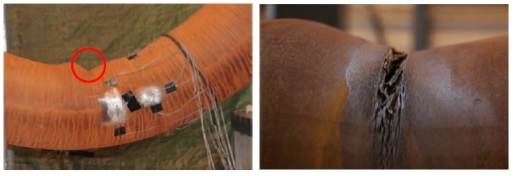Figure 34: Experimental failure (Schaffrath et al. )

For the case considered, the internal pressure applied to the elbow is 20 bars, which leads to a stress value, according to Barlow's formula: $\sigma ={pD{\mathord {\left/{}\right.}}2t}=42.77Mpa$. This is less than 10% of the yield strength for an X60 steel and, consequently, the pressure applied is less than 10% of the internal yield pressure. This puts us in the first yield mode, according to previous results found in literature. The failure due to local buckling obtained for a low value of the internal pressure by Schaffrath et al.  can, however, be a consequence of residual stresses generated in the bending process of the pipe combined with local defects that favored the formation of the buckle in the area shown by the experiment and with the effects generated by the oscillation of the internal pressure.

Summarizing, the number of cycles the simulation lasted and the force displacement curve are in good accordance between the numerical model and the experiment and the numerical failure mode is different from the experimental one but justifiable given the low internal pressure imposed in the model.

### 3.5.5 Considerations regarding the failure mode

In order to assess the capability of the constitutive model to represent both failure modes, a different numerical simulation was done where the internal pressure applied was increased to 220 bars, in order to approximately reach the yield stress. Afterwards, the elbow was subjected to a monotonically increasing in-plane closing displacement.

The model used for this simulation is shown in figure 35. Given the fact that this problem is highly nonlinear and the failure mode expected is achieved thru a local instability (local buckle), in order to achieve convergence when applying the displacement, an initial buckle was imposed on the model. This ensures that, when the internal pressure is sufficiently high, the plastic strain accumulation is directed toward this zone thus enabling model convergence.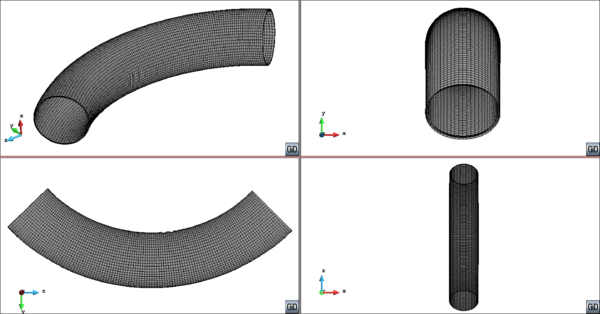Figure 35: Geometry of the model with the initially imposed buckle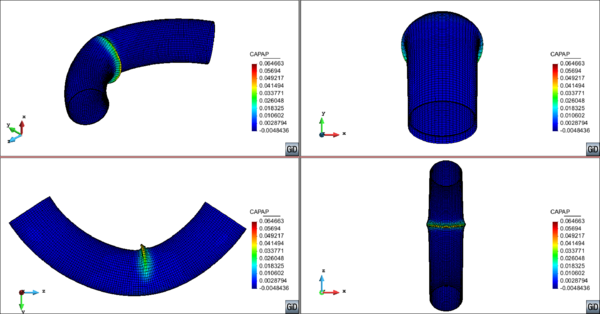Figure 36: Distribution of the plastic internal variable of the model on the deformed shape

The final applied displacement up until which the problem converged was 2.69m, nearly 65% of the total in-plane geometry opening. In figure 36 the distribution of the plastic internal variable is shown in the last converged analysis step on the deformed shape of the model with a scale factor of 1, and, as expected, it exhibits a concentration in the imposed buckle zone.

The purpose of this second simulation was to assess the capability of the numerical formulation to illustrate both failure modes in accordance to the level of internal pressure applied.

From the above numerical simulations it is clear that the failure mode obtained with the formulation is highly dependent on the level of internal pressure applied. Taking this into account, a series of six monotonic simulations have been run varying the internal pressure applied initially and applying afterward an in-plane closing displacement. The maximum dissipation zone was assessed when the applied displacement reached the maximum one imposed in the cyclic large scale initial experiment (see table 3).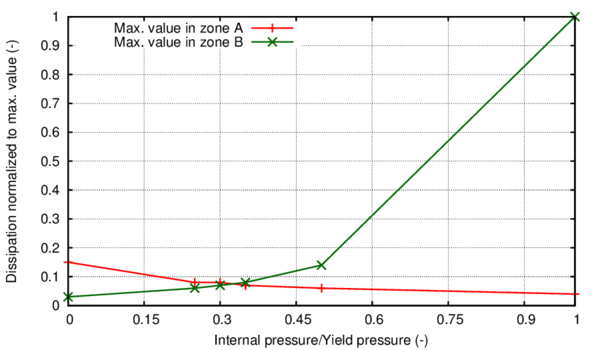Figure 37: Evolution of the maximum dissipation in the two areas of interest

In figure 37 the evolution of the maximum dissipation in the geometry is presented in the two areas that are specific to each failure mode: elbow flanks for the ovalization mode (zone A in table 6 and figure 37) and internal elbow curvature for the local buckling (zone B in table 6 and figure 37).

From both table 6 and figure 37 it can be seen that the switch from the ovalization failure mode to the local buckling occurs between 30 and 35% of the yield internal pressure, since for the 30% case the maximum dissipation is recorded in the elbow flanks and for the 35% case it is present in the buckled area.

## 3.6 Large diameter straight pipe loaded monotonically

In order to analyze the capabilities of the constitutive model presented in previous sections, large scale numerical simulations of a straight pipe OD 168.3 x 4.78mm, X52 grade, will be conducted. The geometry of the model, boundary conditions and sequence of loading are established by the experiment made by Coppola et al. . The specimen drawing can be seen in figure 38.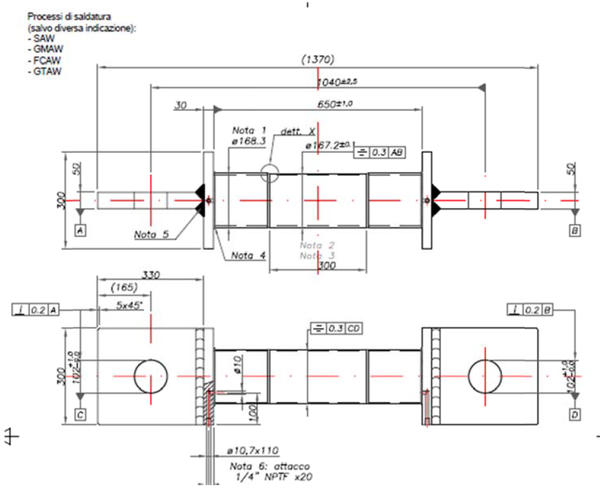Figure 38: Specimen drawing for X52 full scale testing

### 3.6.1 Geometry of the model

The specimen consists of a straight pipe, with three differentiated sections. The central one is mechanized with a reduced thickness, as seen in figure 39 and table 7.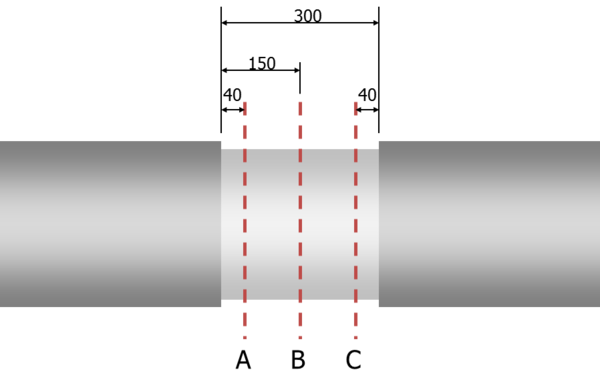Figure 39: Sections of interest in the mechanization of the straight pipe

 Wall Thickness [mm] Outer Diameter [mm] OD 0${}^{\circ }$ 164,2 163,88 164,04 Ref. sec 1: A sec 2: B sec 3: C OD 45${}^{\circ }$ 168,3 168,5 168,42 0${}^{\circ }$ 4,24 4,18 4,36 OD 90${}^{\circ }$ 170,57 170,65 170,34 45${}^{\circ }$ 3,85 4,19 3,99 OD 135${}^{\circ }$ 166,89 166,64 166,04 90${}^{\circ }$ 4,01 3,91 3,77 135${}^{\circ }$ 4,24 4,05 4,10 WT Avg [mm] 4,14 4,14 4,08 180${}^{\circ }$ 4,16 4,17 4,16 WT Min [mm] 3,85 3,91 3,77 225${}^{\circ }$ 4,15 4,19 4,13 OD Avg [mm] 167,49 167,42 167,21 270${}^{\circ }$ 4,02 4,06 4,00 ID Avg [mm] 159,22 159,14 159,04 315${}^{\circ }$ 4,41 4,37 4,16 Aw [mm${}^{2}$, WT Avg, OD Avg] 2122,06 2123,62 2092,82 Ki (WT Avg, OD Avg) 19,765 19,732 19,985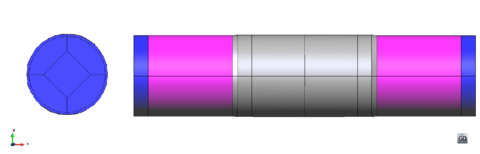Figure 40: Model geometry in GiD

In the numerical model the variation in thickness throughout the central zone has been accounted for, as well as the thickness variation throughout the same cross-section. A view of the model geometry in the pre-postprocessor GiD can be seen in figure 40. Following, in figure 41 a view of the mesh is shown. For this simulation quadratic hexahedral elements were used, each with 20 nodes and 27 integration points. The mesh consisted of 8162 elements and 45865 nodes.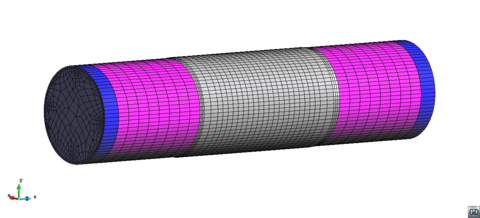Figure 41: Mesh of hexahedral quadratic elements

Regarding boundary conditions, shown in figure 42, one capped end of the pipe has its displacement restricted in all directions, while on the other capped end either force or displacement is applied as required by the loading history. When applying internal pressure, one end remains fixed while on the other one the displacement in the longitudinal axis of the pipe is allowed (z-z axis in figure 41) and restrained in the other two directions. The material of the mechanized part is an X52 steel. The outer parts have an elastic material, with the same Young modulus as X52. The pipe caps have been defined as a rigid material in agreement with the setup of the experiment (see figure 38).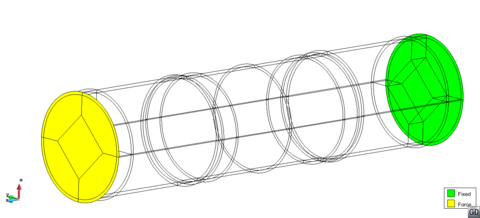Figure 42: Boundary conditions for the straight pipe

For this simulation two load combinations were made. In the first case (SPEC1) a traction force was applied on one of the caps in the longitudinal pipe axis until a level of 400KN. Afterwards, an internal pressure was applied and gradually increased up to burst. Pipe failure occurred at 270 bars with an associated total axial load of 940KN.

In the second case, the test has been done with internal pressure followed by tension. The internal pressure in the first step is 200 bars. Afterwards, the load was increased up to failure which occurred at 884KN mechanical load (1284KN total axial load). Pressure in the second step was maintained constant at 200 bars. In figure 43 the two loading scenarios are presented.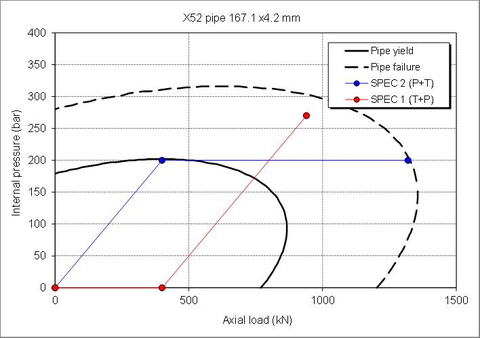Figure 43: Loading scenarios for the straight pipe

### 3.6.3 Material characteristics

The material parameters for the X52 steel have been obtained by undergoing the calibration process described in section 3.4.3 of this document. A polynomial of the 5${\textstyle {}^{th}}$ degree was chosen for the curve fitting zone of the hardening function. The polynomial coefficients as given by the least squares method are shown in table 8.

The remaining material parameters are shown in table 9.These material parameters were obtained by conducting a calibration process on small scale smooth sample specimens. The geometry of the samples and some calibration results for the cyclic case are presented in section 3.3.

 Coefficient no. 1 ${\textstyle a_{0}}$ 240000000,00 Coefficient no. 2 ${\textstyle a_{1}}$ 596993435,99 Coefficient no. 3 ${\textstyle a_{2}}$ -1019807849,94 Coefficient no. 4 ${\textstyle a_{3}}$ 776714259,60 Coefficient no. 5 ${\textstyle a_{4}}$ -265797084,38 Coefficient no. 6 ${\textstyle a_{5}}$ 33562252,72

 Young Modulus 1.8 10${\textstyle {}^{5}}$ MPa Poisson Modulus 0.30 Elastic Stress (${\textstyle \sigma _{Y}^{eq}}$) 240 MPa Plastic Strain Limit for region 1(${\textstyle E_{1}^{p}}$) 15 % Plastic Strain Softening (${\textstyle E_{2}^{p}}$) 50 % ${\textstyle c_{1}}$ kinematic hardening 6.0 10${\textstyle {}^{4}}$ MPa ${\textstyle d_{1}}$ kinematic hardening 280 Fracture Energy 0.4 MN * m/m${\textstyle {}^{2}}$

In figure 44, the monotonic stress strain curve can be seen as obtained with the material parameters presented before. The comparison with the experimental monotonic curves for the X52 steel as obtained from Pereira et al.  can also be seen.

Figure 45 exhibits the comparison between the numerical hysteresis loop shape and the experimental one. Although the straight pipe is loaded monotonically, when conducting the calibration analysis for the material, the shape of the hysteresis was one of the factors taken into account as the model is prepared to conduct monotonic and cyclic tests. The calibration process for a monotonic analysis follows the same guidelines as that of a cyclic analysis. The main difference resides in the plastic strain chosen as threshold for the softening behaviour and in the fracture energy assigned to the material.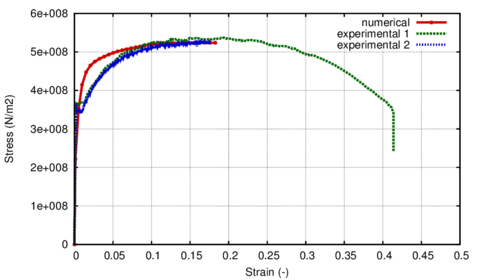Figure 44: Stress-strain curves for the monotonic case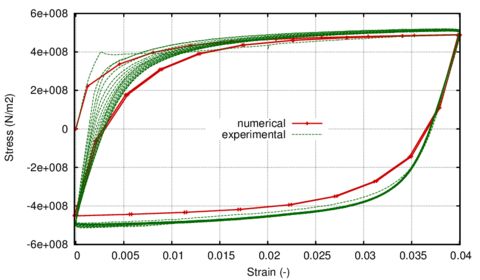Figure 45: Stress-strain hysteresis loop for $\Delta$$\varepsilon$=4% Numerical vs. experimental.

## Case no. 1 - Tension followed by pressure

In figure 46, the comparison between the experimental and the numerical force-pressure measurements can be seen. The total reaction recorded by the numerical simulation reached a level of 948KN in the last converged increment, corresponding to an applied internal pressure of 278.1bars.

For this increment, the deformed shape of the specimen is shown in figure 47, where the distribution of the hardening internal variable ${\textstyle \kappa ^{p}}$ can also be seen. This variable is of relevance in showing the level of dissipated energy at material point level and, in this sense, gives a measure of the level of degradation suffered. Consequently, for ${\textstyle \kappa ^{p}=0}$ the material is in an elastic state, while for ${\textstyle \kappa ^{p}=1}$ the material has reached total failure at that material point.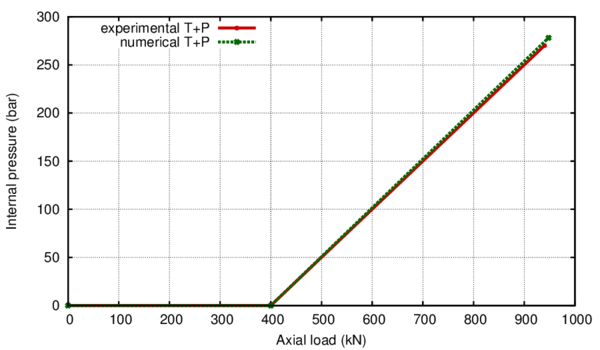Figure 46: Comparison between the numerical and experimental results for the SPEC 1 case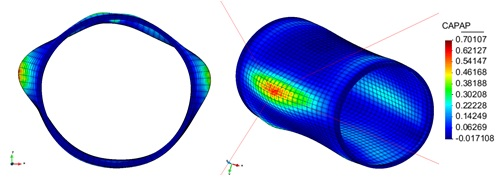Figure 47: View of the deformed shape at the end of the analysis with an indication of the most damaged material point in the geometry

From the deformed shape it can be seen that the pipe failure is oriented following the direction of least resistance represented by the minimum thickness area. This is in agreement with the experimental localization of the failure as can be seen in figure 48.

Also, there is good agreement of the failure mode between the experiment and the numerical simulation with a final burst opening oriented in the longitudinal direction. In the numerical model the burst area is represented by the localized plastic strain accumulation reflected in the distribution of the normalized dissipation parameter.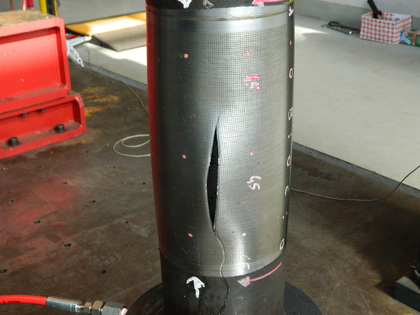Figure 48: View of the pipe burst as recorded by the experiment for the SPEC1 test

Figure 49 shows the evolution of the hardening internal variable ${\textstyle \kappa ^{p}}$ at the most damaged integration point in the material. Its exact location is indicated in figure 47. As it can be seen from figure 49, the simulation converged up until a maximum value of the ${\textstyle \kappa ^{p}}$ internal variable of 0.7. Given that the internal pressure has been applied in constant increments of 2.7 bars per analysis step, it can be seen that the evolution of the ${\textstyle \kappa ^{p}}$ parameter exhibits an exponential curve. Taking into account this tendency and extrapolating on the last converged increment that had a value of 0.7, loss of convergence seems to have occurred when the plastic internal variable reached a value very close to 1, corresponding to an open crack generated at the material points where the entire fracture energy of the material has been spent. However, this value is not visible in figure 49 since convergence was not reached for this increment.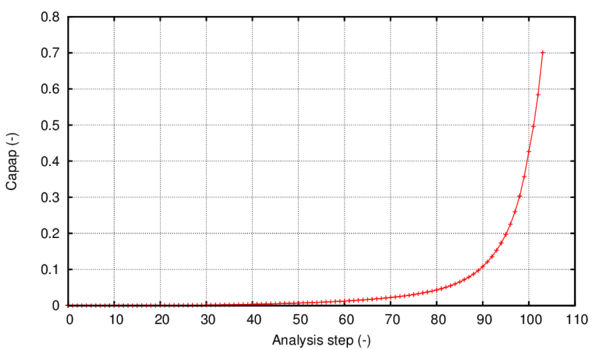Figure 49: Evolution of the $\kappa ^{p}$ variable when applying internal pressure in constant steps of 2.7 bars

## Case no. 2 – Internal pressure followed by tension

In figure 50 the comparison between the experimental and the numerical force-pressure measurements can be seen for this case.

The total reaction recorded by the numerical simulation reached a level of 1167KN in the last converged step, corresponding to a total applied displacement of 1.375m. The experimental failure occurred at a total axial load level of 1284kN.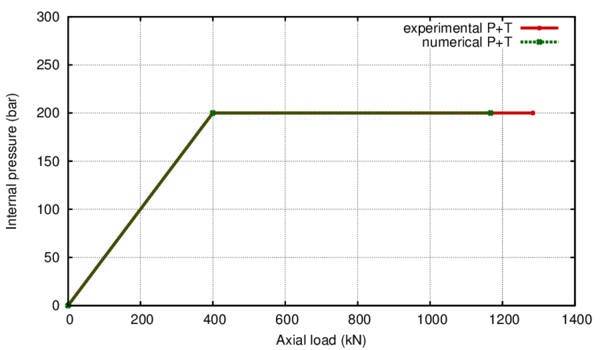Figure 50: Comparison between the numerical and experimental results for the SPEC 2 case

As specified before, during the applied displacement stage the internal pressure was maintained constant at a level of 200 bars. For the last step of the simulation the deformed shape is shown in figure 51, where the distribution of the hardening internal variable ${\textstyle \kappa ^{p}}$ can also be seen.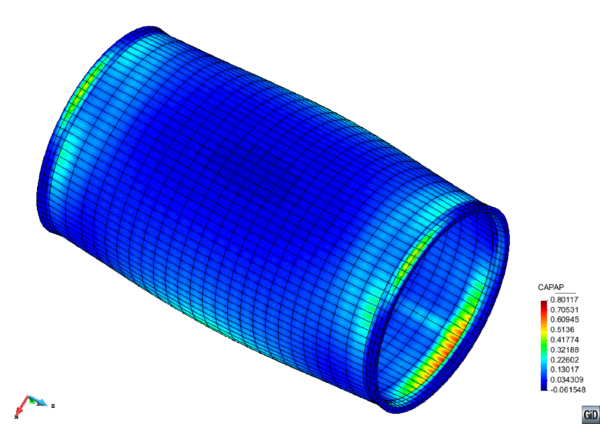Figure 51: View of the deformed shape for the SPEC 2 case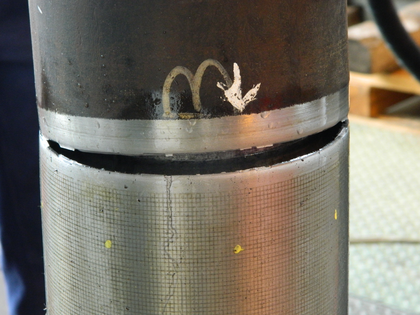Figure 52: View of the pipe burst as recorded by the experiment for the SPEC2 test

The localization of the failure zone corresponds to the behaviour shown in the experiment, presented in figure 52. When considering the pipe cross section where the maximum dissipation is present, the most stressed area is directed towards the smallest thickness in that particular circumference, corresponding with the data recorded by the experimental campaign and taking into account the orientation of the numerical model with respect to that of the experimental setting. The failure mode is a tensile one, in agreement to the applied sequence of loading.

## 3.7 Advantages of the approach proposed

Previous results have shown that the proposed constitutive model is capable of predicting material failure after applying several cycles to the material.The formulation is also capable of predicting the structural failure under monotonic loads. However, this prediction capability do not present a major advantage compared to other approaches such as the Coffin-Manson rule, or any other analytical expression capable of defining the maximum number of cycles that can be applied for a given plastic strain.

The main advantage of the proposed approach is that the prediction of ULCF failure does not depend on the applied plastic strain, but on the energy dissipated during the cyclic process. Therefore, it is possible to vary the plastic strain in the cycles applied to the structure and the constitutive model will be still capable of predicting the material failure.

This is proved in the following example, where an irregular load, in frequency and amplitude, is applied to the SP sample defined in section 3.3 and used to validate the formulation. The load defined is depicted in figure 53.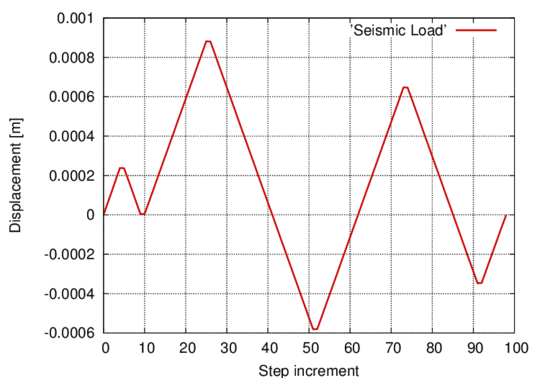Figure 53: Seismic-type load applied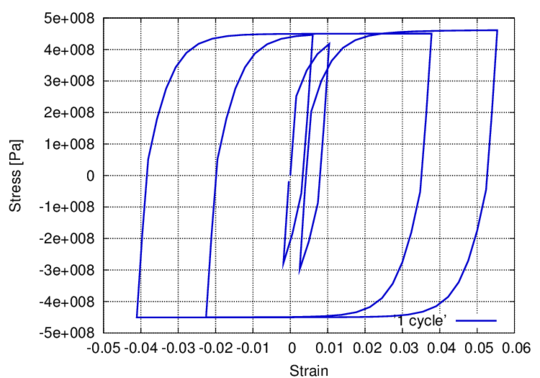Figure 54: Material stress-strain response
This load is applied as a fixed displacement following the same procedure used for the SP sample. The stress strain graph obtained from the numerical model is plotted in figure 54. As it can be seen, the applied load produces several hysteresis loops, each one with a different plastic strain.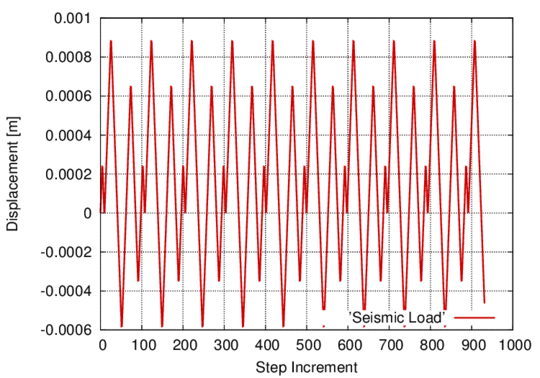Figure 55: Seismic-type load applied: ten seismic-type cycles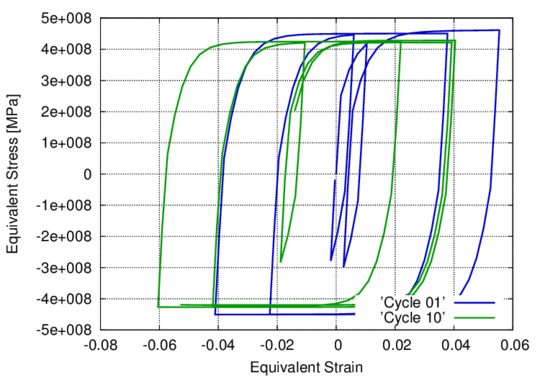Figure 56: Material stress-strain response after ten seismic-type cycles

The model is capable of capturing the energy dissipated in each one of these loops and, therefore, to evaluate the energy available in the material after having applied the load, which is equivalent to the residual strength of the material. It is also possible to repeat several times the irregular load, as shown in figure 53, to study the number of repetitions that are required to reach material failure. Figure 56 shows the stress-strain response of the material after 10 cycles (figure 55). At this point there are some points in the model that have lost most of their fracture strength and specimen failure occurs. As occurred with the SP model, this simulation also shows some lateral displacement on the equivalent strains due to the plastic strains suffered by the whole specimen.

# 4 Constitutive modelling of High Cycle Fatigue

## 4.1 Introduction

A stepwise load-advancing strategy for cyclic loading will be presented in this chapter that yields convergence in reasonable computational time for highly nonlinear behaviour occurring past the S-N curve. The algorithm is also effective when dealing with combinations of cyclical loads. The strategy is coupled to a continuum damage model for mechanical fatigue analysis. An overview of the constitutive model is also presented. The capabilities of the proposed procedure are shown in several numerical examples. The model is validated by comparison to experimental results.

The basis of the HCF constitutive model used was initially developed by Oller et al. . The model establishes a relationship between the residual material strength and the damage threshold evolution, controlled by the material internal variables and by a new state variable of fatigue that incorporates the influence of the cyclic load. A brief overview of the constitutive formulation for the HCF case is provided in order to clarify the material behaviour exhibited in the numerical examples. Several model assumptions are to be made. Defect concentration on the microscale occurs during the whole period of cyclic loading. This is reflected in the model in a continuous reduction of the material strength, occurring even in the elastic stage. Stiffness degradation occurs only in the post critical stage, once the ${\textstyle S-N}$ curve has been passed and, therefore, only in the final stage before failure. The damage parameter has a phenomenological significance indicating the irreversibility of the fatigue process.

Depending on the size of the domain chosen for a fatigue numerical simulation, computational time for a numerical analysis can vary considerably. Nowadays, running simulations at macroscale level (mechanical part, structural element) continues to be a challenge, especially if the high level of structural complexity attained at the microscale needs to be taken into account to some extent at other scales. This work offers a stepwise load-advancing strategy that allows a saving of computational time and can help push the barrier of what if possible in terms of numerical simulation one step further.

The strategy can be especially effective when dealing with HCF where material lives are in the range of 10${\textstyle {}^{6}}$ – 10${\textstyle {}^{7}}$ cycles. If a single loading cycle is described by n loading steps and the structure fails at 10${\textstyle {}^{7}}$ cycles, then the number of loading steps required to complete a HCF analysis would be in the order of 10${\textstyle {}^{7}}$ x n. Furthermore, if the mechanical piece has a complex geometry and a high level of discretization is required at finite element level, then at each of the 10${\textstyle {}^{7}}$ x n load steps a large number of constitutive operations need to be computed for each integration point. The above serve as a clear example of why load-advancing strategies are of the utmost importance in HCF simulations.

Furthermore, increasingly more attention has been given to material behaviour in the very high cycle regime from an experimental point of view. The general belief that steel experiences no alteration in its properties after reaching its fatigue limit at 10${\textstyle {}^{7}}$ cycles has been invalidated , , . In this context, this work provides a tool for rapid automatized time-advance that allows taking numerical simulations beyond the limit of 10${\textstyle {}^{7}}$ cycles in reasonable computational time with the added benefit of being able to predict fatigue failure when dealing with combinations of different cyclical loads and also of being able to evaluate the residual strength of the material once a cyclical load has finished.

## 4.2 HCF damage model

A description of the constitutive model is offered in this section. The fundamentals of a fatigue continuum damage model are presented with a clear emphasis on the dependence of the model on the S-N curves. An exhaustive description of the formulation used can be found in Oller et al. , where the complete thermo-mechanical constitutive model for the prediction of fatigue effects in structures is formulated. The model is capable of taking into account the effect of the mean stress. The treatment of the highly complex processes generated by fatigue is made from a phenomenological point of view.

### 4.2.1 Mechanical damage formulation

The free Helmholtz energy is formulated in the reference configuration for elastic Green strains, ${\textstyle E_{ij}=E_{ij}^{e}}$, as :

 $\Psi =\Psi (E_{ij},d)=(1-d){\frac {1}{2m^{0}}}(E_{ij}C_{ijkl}^{0}E_{kl})$
(33)

where ${\textstyle m^{0}}$ is the material density, ${\textstyle E_{ij}=E_{ij}^{e}}$ is the total strain tensor, ${\textstyle 0\leq d\leq 1}$ is the internal damage variable taking values between its initial value 0 and its maximum value 1 and ${\textstyle C_{ijkl}^{0}}$ is the initial constitutive tensor.

Considering the second thermodynamic law (Clausius-Duhem inequality –   ), the mechanical dissipation can be obtained as 

 $\Xi =-{\frac {\partial \Psi }{\partial d}}{\dot {d}}\geq 0$
(34)

The accomplishment of this dissipation condition (Equation 34) demands that the expression of the stress should be defined as (Coleman method; see )

 $S_{ij}=m^{0}{\frac {\partial \Psi }{\partial E_{ij}}}=(1-d)C_{ijkl}^{0}E_{kl}$
(35)

Also, from the last expressions, the secant constitutive tensor ${\textstyle C_{ijkl}^{s}}$ can be obtained as:

 $C_{ijkl}^{s}(d)={\frac {\partial S_{ij}}{\partial E_{ij}}}=m^{0}{\frac {\partial ^{2}\Psi }{\partial E_{ij}\partial E_{kl}}}=(1-d)C_{ijkl}^{0}$
(36)

where ${\textstyle S_{ij}^{}}$ is the stress tensor for a single material point.

### 4.2.2 Threshold damage function oriented to fatigue analysis. Phenomenological approach

The effects caused by applying an increasing number of loading cycles are taken into account by means of a proposed ${\textstyle f_{red}(N,S_{\max },R)}$ function. This function is introduced in the above formulation in the expression of the damage threshold surface, ${\textstyle F^{D}(S_{ij},d)}$, proposed by ,  and . The number of cycles N can then be incorporated as a new variable. This enables the classical constitutive damage formulation to account for fatigue phenomena by translating the accumulation of number of cycles into a readjustment of the damage threshold function.

The non-linear behaviour caused by fatigue is introduced in this procedure implicitly, by incorporating a fatigue state variable, ${\textstyle f_{red}(N,S_{\max },R)}$, that is irreversible and depends on the number of cycles, the maximum value of the equivalent stress in the material, ${\textstyle S_{\max }}$, and on the factor of reversion of the equivalent stress, ${\textstyle R=S_{\min }/S_{\max }}$. This new variable affects the residual strength of the material by modifying the damage threshold, ${\textstyle F^{D}(S_{ij},d,N)}$, either on the equivalent stress function ${\textstyle f^{D}(S_{ij})}$ (equation 37), or on the damage strength threshold ${\textstyle {\bar {K}}^{D}(S_{ij},d)}$(equation 38) .

 $F^{D'}(S_{ij},d,N)=\underbrace {\frac {f^{D}(S_{ij})}{f_{red}(N,S_{\max },R)}} _{f^{D'}(S_{ij},N,R)}-{\bar {K}}^{D}(S_{ij},d)\leq 0$
(37)

 $F^{D''}(S_{ij},d,N)=f^{D}(S_{ij})-\underbrace {{\bar {K}}^{D}(S_{ij},d)\cdot f_{red}(N,S_{\max },R)} _{K^{D'}(S_{ij},d,N)}\leq 0$
(38)

In the above, ${\textstyle f^{D'}=f^{D}/f_{red}(N,S_{\max },R)}$, is the reduced equivalent stress function in the undamaged space, ${\textstyle K^{D'}(S_{ij},d,N)}$ is the fatigue damage strength threshold, and ${\textstyle d=\int _{0}^{t}{\dot {d}}dt}$ the damage internal variable. In the following, the form in equation 37 has been used for the damage threshold criterion.

The evolution of the damage variable is defined as:

 ${\dot {d}}={\dot {\mu }}{\frac {\partial F^{D}}{\partial f^{D}}}$
(39)

being ${\textstyle \mu }$ the consistency damage factor, which is equivalent to the consistency plastic factor defined in . Consequently, for the isotropic damage case,

 ${\dot {d}}={\frac {\dot {\mu }}{f_{red}}}$
(40)

### 4.2.3 Particularization of the damage threshold function for exponential softening

The type of softening to be defined in the general damage criterion depends on the problem to be solved. The scalar function defining the evolution of the damage threshold must be monotonous and with a value ranging from 0 to 1. In various publications about the scalar damage problem, the stress behaviour with softening is represented in a variety of forms. Particularly, in Oliver et al.  the following function is proposed,

 $G\left[{\bar {K}}^{D}(d)\right]=1-{\frac {{\bar {K}}^{D\max }}{{\bar {K}}^{D}(d)}}e^{A\left(1-{\frac {{\bar {K}}^{D}(d)}{{\bar {K}}^{D\max }}}\right)}{\;with\;\;\;\;}0\leq {\bar {K}}^{D\max }\leq {\bar {K}}^{D}(d)$
(41)

This function can also be expressed as,

 $G\left[f^{D'}(S_{0}^{},N)\right]=1-{\frac {f_{0}^{D'}(S_{0}^{},N)}{f^{D'}(S_{0}^{},N)}}e^{A\left(1-{\frac {f^{D'}(S_{0}^{},N)}{f_{0}^{D'}(S_{0}^{},N)}}\right)}{\;with\;\;\;\;}f_{0}^{D'}(S_{0}^{},N)={\bar {K}}^{D\max }$
(42)

where ${\textstyle A}$ is a parameter that depends on the fracture energy of the material and ${\textstyle f_{}^{D'}(S_{0}^{},N)=f^{D}(S_{0}^{})/f_{red}(S_{\max },N,R)}$. The value of ${\textstyle f_{0}^{D'}(S_{0}^{},N)={\bar {K}}^{D\max }}$ is obtained from the fulfilment of the damage criterion for the first threshold of degradation. This complies with ${\textstyle G\left[f_{0}^{D'}(S_{0}^{},N)\right]-G\left[{\bar {K}}^{D\max }\right]^{}=0}$ , and ${\textstyle G\left[f_{0}^{D'}(S_{0}^{},N)\right]=G\left[{\bar {K}}^{D\max }\right]^{}\equiv 0}$.

The A parameter is calculated from the dissipation expression shown in equation ${\textstyle \Xi =\Psi _{0}\,{\dot {d}}\geq 0}$, particularized for a uniaxial process subjected to a growing monotonic load. The parameter deduction can be obtained from  and has the following expression for exponential softening:

 $A={\frac {1}{{\frac {C_{0}\,g_{f}}{\left(\tau ^{0}\right)^{2}}}-{\frac {1}{2}}}}$
(43)

### 4.2.4 Function of residual strength reduction for fatigue – Wöhler curve definition

Wöhler or Stress-Number of cycles (S-N) curves (figure 57) are experimentally obtained by subjecting identical smooth specimens to cyclic harmonic stresses and establishing their life span measured in number of cycles. The curves depend on the level of the maximum applied stress and the ratio between the lowest and the highest stresses (R=S${}_{min}$/S${}_{max}$). In figure 57b S${}_{lim}$ is the endurance limit for a reversion factor of -1 and ${\textstyle S_{f}^{0}}$ is the material elastic limit. In figure 57a the instantaneous stress level is depicted, while in figure 57b the cyclic stress is represented only by the maximum value it reaches in every cycle. Usually, S-N curves are obtained for fully reversed stress (R=S${}_{min}$/S${}_{max}$=-1) by rotating bending fatigue tests.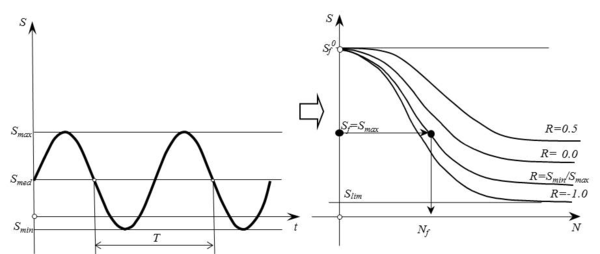Figure 57: a: Stress evolution at a single point; b: $S-N$ (Wöhler's) Curves

$S-N$ curves are, therefore, fatigue life estimators for a material point with a fixed maximum stress and a given ratio R. If, after a number of cycles lower than the cycles to failure, the cyclic load stops, a change in the elastic threshold of the material is expected due to accumulation of fatigue cycles. Furthermore, if the number of cycles exceeds Nf, being Nf the fatigue life as resulting from figure 58, the material will fail with the consequent reduction of strength and stiffness. The change in strength is quantified by the strength reduction function, ${\textstyle f_{red}(N,S_{\max },R)}$, while the change in stiffness is taken into account by means of the damage parameter. In figure 58, S${}_{th}$ is the endurance limit for any given reversion factor and S${}_{u}$ is the elastic threshold limit.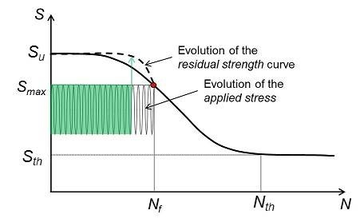Figure 58: Schematic representation of the evolution of the residual strength with the applied load and number of cycles

In the case of a cyclic load with constant S${}_{max}$ and R throughout the entire life of a material, the ${\textstyle S-N}$ curve is sufficient for determining fatigue life. However, when dealing with different load interactions the main focus resides on the residual strength curve. The curve quantifies the loss of strength in the material as the number of cycles accumulates and as load characteristics change.

All fatigue numerical simulations are based on the Wöhler curves obtained experimentally. These curves are described in an analytical form with the help of material parameters. Their expression, as well as the analytical definition of the strength reduction function, is connected to the experimental curve and, therefore, subjected to change if the material changes. Different analytical definitions can be found in ,  and , as well as in .

Here the analytical fomulation presented in  is shown.

Based on the actual value of the R ratio and a basic value of the endurance stress ${\textstyle {S}_{e}}$ (for ${\textstyle {R}=-1}$) the proposed model postulates a threshold stress ${\textstyle {S}_{th}}$. The meaning of ${\textstyle {S}_{th}}$ is that of an endurance stress limit for a given value of R=S${}_{min}$/S${}_{max}$.

 ${\begin{array}{l}|R|\leq 1\\{{S}_{th}(R)={S}_{e}+({S}_{u}-{S}_{e})*{(0.5+0.5*R)}^{STHR1}}\\{else}\\{{S}_{th}(R)={S}_{e}+({S}_{u}-{S}_{e})*{(0.5+0.5/R)}^{STHR2}}\\{end}\end{array}}$
(44)

If the actual value of R is ${\textstyle {R}=-1}$ then, ${\textstyle {S}_{th}={S}_{e}}$. The effect of the number of load cycles ${\textstyle {N}_{c}}$ on the ultimate stress ${\textstyle {S}_{u}}$ for a given value of R is taken into account by an exponential function,

 $S(R,{N}_{c})={S}_{th}(R)+({S}_{u}-{S}_{th}(R))*e^{-ALFAT(R)*{\log _{10}{{N}_{c}}}^{BETAF}}$
(45)

The value of ${\textstyle ALFAT(R)}$ is given by the function,

 ${\begin{array}{l}|R|\leq 1\\{ALFAT(R)=ALFAF+(0.5+0.5*R)*AUXR1}\\{else}\\{ALFAT(R)=ALFAF-(0.5+0.5/R)*AUXR2}\\{end}\end{array}}$
(46)

where ${\textstyle ALFAF,BETAF,STHR1,STHR2,AUXR1}$ and ${\textstyle AUXR2}$ are material parameters that need to be adjusted according to experimental tests. Figure 59 shows an example of application of these functions. Parameters were chosen as follows: ${\textstyle {S}_{e}=0.5*{S}_{u}}$, ${\textstyle ALFAF=0.0068}$, ${\textstyle BETAF=3.35}$, ${\textstyle STHR1=0.7}$, ${\textstyle STHR2=0.5}$, ${\textstyle AUXR1=0.0133}$ and ${\textstyle AUXR2=0.0068}$.

 [[ Figure 59: Proposed $S-N$ curves for different values of $R=({S}_{min}/{S}_{max})$. 

The proposed ${\textstyle S-N}$ curves, equations 44 - 46, are fatigue life estimators for a material point with a fixed maximum stress and a given ratio ${\textstyle R}$. If, after a number of cycles lower than the cycles to failure, the constant amplitude cyclic load with a maximum stress ${\textstyle {S}_{max}}$ (and ratio ${\textstyle R}$) is removed, a change in ${\textstyle {S}_{u}}$ is expected due to the accumulation of fatigue cycles. In order to describe that variation of ${\textstyle {S}_{u}}$ the following function is proposed:

 ${f}_{red}(R,{N}_{c})=e^{-B0*(\log _{10}{{N}_{c}})^{BETAF*BETAF}}$
(47)

where ${\textstyle BETAF}$ is one of the parameters of equation 45 and ${\textstyle B0}$ is obtained as a function of the ratio ${\textstyle {S}_{max}/{S}_{u}}$ and the number of cycles to failure ${\textstyle {N}_{f}}$, by:

 $B0=-{\dfrac {\ln \left({S}_{max}/{S}_{u}\right)}{\left(\log _{10}{{N}_{f}}\right)^{BETAF*BETAF}}}$
(48)

The value of ${\textstyle {N}_{f}}$ can be obtained from equation 45:

 ${N}_{f}=10^{-{\dfrac {1}{BETAF}}\,{\dfrac {1}{ALFAT(R)}}\,\ln {\left({\dfrac {{S}_{max}-{S}_{th}(R)}{{S}_{u}-{S}_{th}(R)}}\right)}}$
(49)

Some important observations have to be made regarding the fatigue strength reduction function. Habitually, fatigue models are limited to correctly describing the ${\textstyle S-N}$ curves and, based on the level of stress applied, estimate the fatigue life. The ${\textstyle S-N}$ curve is generally interpreted as a life prediction tool, meaning that when, for a certain level of stress, the ${\textstyle {N}_{c}}$ corresponding to the Wöhler curve has been reached the respective Gauss point has suffered complete degradation. However, the present model utilizes the respective ${\textstyle {N}_{c}={N}_{f}}$ point as the starting point for damage accumulation and the nonlinear zone, for that particular Gauss point. This event mirrors the initiation point for a microcrack. Posterior damage accumulation is an indicator of a rearrangement of the internal structure of the material followed by micro-fisuration and a completely degraded Gauss point (${\textstyle {d}_{GP}=1}$) represents the formation of a macro-crack in the volume associated to the integration point. At macroscale level, a tracking of the damage propagation throughout the continuum is an indicator of a crack propagation and total structural rupture is considered to have occurred when the crack has propagated thru the entire cross-sectional area.

The analytical formulation mentioned above has been coupled with a signal processing routine so that random cyclical loading can be decomposed into different load sections, each described by its characteristics: amplitude, reversion factor, number of cycles, period. The residual strength curve quantifies the reduction in material strength due to the application of each loading section in a particular order, as will be shown in the numerical examples presented later on in this chapter.

### 4.3.1 Introduction

The stepwise load-advancing strategy proposed in this chapter uses the formulation described previously and consists of two different phases. The first one is defined by load-advance being conducted by small time increments, with the consequent load variation following a cyclic path. The second phase is characterized by load-advance being done with large increments of number of cycles.

Even though Oller et al.  described the possibility of advancing thru large increments of number of cycles, the process was not automatic. This implied that it could not be used in real life simulations where an in-depth tracking of the material's progressive degradation was desired, or where the loading history was comprised of several different cyclical loads.

This work proposes an algorithm that automatically switches from one phase to the other, going repeatedly back and forth between both in accordance with the loading input and the damage increase rate.

This phase is characterized by the load being applied in small increments. The purpose is to determine and save the characteristics of the cyclical load. The tags Ai referenced in the following can be seen in the flow chart for this phase, on the left side of figure 60.

Each load cycle will be divided into m small steps, a value that is user defined. At the beginning of each increment being conducted in this phase, both the load factor and the number of cycles will be updated (A1). Based on the multiaxial stress state, the equivalent stress will be computed according to the damage criterion chosen (von Mises, Mohr-Coulomb, Tresca, Drucker-Prager). After that, the difference between the equivalent stress ${\textstyle f^{D}(S_{ij})}$ of current increment k and previous increment k-1 will be compared to the difference between the equivalent stress in k-1 with respect to k-2 (A2)(See figure 60). When the sign of these two quantities is different, either a maximum or a minimum has been recorded in increment k-1(A3). After having detected both the maximum and the minimum equivalent stress for each integration point, the reversion factor is computed, R=S${}_{min}$/S${}_{max}$. After each new cycle i+1 is described, the reversion factor is compared to its value in the previous cycle i. The normalized variation of the reversion factor is evaluated for each integration point GP and the sum of all the variations detected is computed into a stabilization norm ${\textstyle \eta }$ as shown in equation 50 (A4).

 $\eta _{}=\sum _{GP}\left|{\frac {R_{GP}^{i+1}-R_{GP}^{i}}{R_{GP}^{i+1}}}\right|\,\,\,\,\leq toler.$
(50)

A new value for ${\textstyle f_{red}(N,S_{\max },R)}$ is computed and the equivalent stress is then affected by it and compared to the damage strength threshold in the current increment (A5). The check for global convergence is made and, if this is achieved, then the stabilization norm is compared to a user defined tolerance. When this norm is below a given tolerance, it can be said that the reversion factor has a stable value throughout the solid (A6). A flag is then activated indicating that in the next increment the large phase algorithm should be followed. If the value of ${\textstyle \eta }$ is not below the tolerance, several more cycles are then analysed applying small increments.

This phase is necessary at the beginning of each different cyclical load in order to determine the parameters that define the cyclic behaviour at each Gauss point of the structure (R and S${}_{max}$). Therefore, in case of modifying the cyclic load, a new activation is necessary.

### 4.3.3 Large increments phase

After the stress parameters, R and S${}_{max}$, stabilize throughout the solid from one cycle to the other, there is no need to keep applying small increments as there will be no change in the stress state unless either the elastic threshold is reached or the applied cyclical load changes. Therefore, the load level can be maintained at its maximum value and large increments of number of cycles can be applied. The tags Bi referenced in the following can be seen in the flow chart for this phase, on the right side of figure 60.

In this phase the variable is not the level of the load, kept constant at its maximum value, but the number of cycles, which, in each increment, is updated with a new large Nc step (B1). After obtaining the equivalent stress, a new value for ${\textstyle f_{red}(N,S_{\max },R)}$ is computed directly with the current number of cycles and the previously stored values for R and S${}_{max}$ (B2).The equivalent stress affected by ${\textstyle f_{red}(N,S_{\max },R)}$ is then compared with the current damage strength threshold. If nonlinear behaviour occurs at at least one integration point a flag is activated (B3). When global convergence of the problem has been obtained in the current increment and the flag has been activated inside the constitutive loop, the next increment will be conducted with the load-tracking algorithm (B4).If convergence has been reached but the flag was not activated, in the next increment another large step will be applied.

### 4.3.4 Automatic load-tracking phase activation

The stepwise advancing strategy has the following implications:

When applying a single cyclical load, load advance will be done by passing initially thru the load-tracking phase until R stabilizes and ${\textstyle \eta }$ is lower than the defined tolerance. Afterwards, the advancing scheme will be by number of cycles until reaching the elastic threshold. This happens when the material has been subjected to the number of cycles indicated by the S-N curve. At this stage, the internal forces of the structure are modified in order to reach a new equilibrium configuration. This situation leads to a variation of the reversion factor and, therefore, of the stress state at integration point level. The load-tracking phase is automatically activated. Furthermore, it will be activated at each step where damage increases (${\textstyle {\dot {d}}>0}$) due to the change in internal forces.

The algorithm can be optimized if, after evaluating the Wöhler's Nf (marked dot in figure 58) corresponding to each equivalent stress level at the beginning of the analysis, a search is made to find the minimum fatigue life throughout the solid. The resulting number of cycles can be used as the first step of the large increments phase ensuring that the entire span of number of cycles before the damage process initiates is done in one step (B1)(See figure 60). The nonlinear processes occurring past the point damage initiates in the first Gauss point will be simulated with a user-defined N${}_{c}$ step in the case of displacement controlled simulations where the material can continue bearing the cyclical load after having reached the fatigue life given by the S-N curve. This is possible due to a progressive loss of stiffness that ensures that, for the same applied cyclical displacement and having reached the S-N curve, stress in the material progressively relaxes as it suffers damage until total rupture.

In the case of applying different cyclic loads, damage can appear either due to fatigue or due to a new load being applied that leads to stress values that surpass the elastic threshold. In both cases the model will jump automatically from the large increments phase to the load-tracking phase. Even if the different cyclic loads applied induce stress levels below the elastic threshold, when passing from one cyclic load to another one of different characteristics an activation of the load-tracking is required. This is necessary regardless of the elastic regime due to the fact that, by applying a new load, the maximum equivalent stress induced and/or the reversion factor has changed and, consequently, the fatigue parameters calculated for the first load are no longer valid.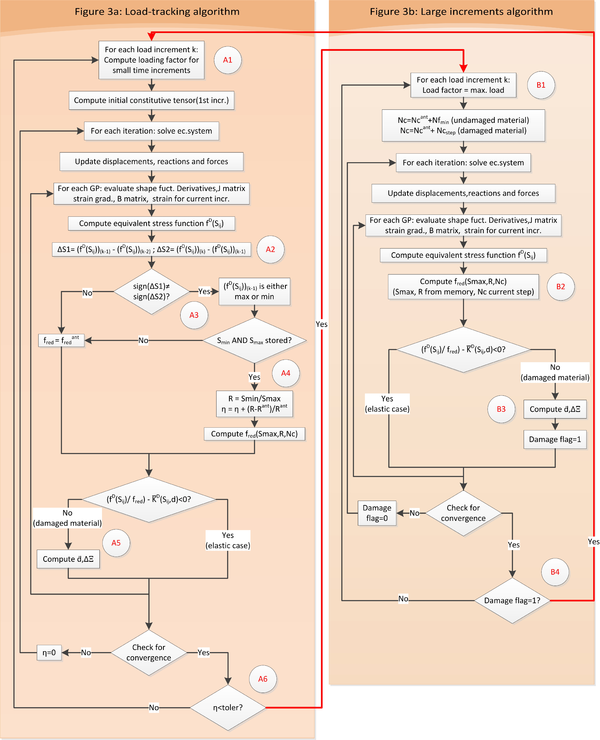Figure 60: Flow chart for the stepwise advancing algorithm

The flow chart presented in figure 60 shows the operations conducted in both phases, as well as the conditions required to jump from load-tracking to large increments. These jumps are indicated with hyphenated arrows.

The algorithm is user controlled by means of two parameters. The first one is the tolerance at which the reversion factor norm (defined in equation 50) is considered to have converged. If, for instance, a numerical tolerance of 10${\textstyle {}^{-10}}$ is used, time advance runs the risk of being continually conducted in the load-tracking phase. This would lead to a dramatic increase in computational time. On the other hand, if the tolerance is set too high the model may no longer capture changes in the cyclical load applied, leading to an incorrect life prediction. The tolerance used for the calculation of the numerical examples presented in section 4 was 10${\textstyle {}^{-4}}$ and the author recommends this value for future use of the strategy.

The second parameter that allows the user to control the developed stepwise load advancing algorithm is the number of cycles chosen as time step for the large increments phase. The influence it has is in accordance with the level of nonlinearity of the problem. While a too low number of cycles used as step implies an increase in computational time, a too big one can lead to loss of solution convergence. A calibration analysis consisting of a study of the solution convergence depending on the number of cycles used as a step is highly recommended. The analysis should begin by using large steps. Then the N${}_{c}$ used as a step should be progressively lowered until convergence is reached, as this would ensure the lowest computational time.

## 4.4 Numerical examples

The capabilities of the stepwise load-advancing strategy, together with the fatigue constitutive model presented, will be shown with several simulations made over a hexahedral finite element subjected to cyclic loads. These examples will illustrate the fatigue behaviour under a single cyclical load and under load interaction of two different cyclic loads. Also, the material behaviour is shown when combining a cyclical load with a monotonic one. After that, the model is validated comparing the results obtained from the numerical simulation with the results provided by the experiment presented in Marines et al. .

All the examples presented in this section have been calculated with the PLCd finite element code , where the formulation presented here has been implemented.

In order to understand the capabilities of the stepwise load advancing strategy, a test case of one linear hexahedral element with 8 integration points will be presented. A single element was chosen for the simulation due to its reduced computational cost and due to the fact that it suffices for accurately showing material behaviour as described by the formulation presented.

### 4.4.1 Problem definition. Geometry, material and boundary conditions.

The element has one of its faces subjected to a cyclical displacement while the opposite face has boundary conditions that fix its longitudinal displacement, allowing transversal expansion and contraction.

Geometry dimensions are ${\textstyle 10*10*10mm}$. The material used has the following characteristics: Young modulus = 2.01 x 10${\textstyle {}^{5}}$ MPa ; Poisson ratio = 0.3 ; Static elastic threshold is S${\textstyle {}_{u}}$ = 838.9MPa and the material fracture energy has a value of G${\textstyle {}_{t}}$ = G${\textstyle {}_{c}}$ = 20 kN*m/m${\textstyle {}^{2}}$ . The damage model used has exponential softening and a Von Mises failure surface. The ${\textstyle S-N}$ curve for this material was obtained using the analytical formulation described in . The parameters required in order to correctly describe the curve are S${}_{u}$, S${}_{max}$ and R.

One of the model's particularities is the progressive loss of resistance leading to the initiation of damage, represented in the strength reduction curve. In order for it to be clearly differentiated from the Wöhler curve, a direct jump to the point where damage initiates was not done. Rather, an approximation of the damage initiation point was made by choosing a suitable number of cycles as the time step.

The first two numerical simulations presented exhibit a load history composed of a single cyclical load, while the last two simulations consist of applying both cyclical loads in different order.

The characteristics of the cyclical loads taken into account are: for the first one, ${\textstyle {S}_{1}}$, a reversion factor of 0.3, a minimum displacement of 0.0114mm and a maximum displacement of 0.038mm; in the second case, ${\textstyle {S}_{2}}$, the first load applied has a null reversion factor, a maximum displacement of 0.035mm and a null minimum displacement. The number of cycles adopted as a step for the large increments phase in the both cases is 10${\textstyle {}^{6}}$ cycles.

For the combination cases the load applied is the first one, ${\textstyle {S}_{1}}$, and, after 10${\textstyle {}^{6}}$ cycles, this load is replaced by ${\textstyle {S}_{2}}$. The number of cycles used as a step for the large increments phase in this case is 10${\textstyle {}^{5}}$ cycles. In the second combination case the order of loads applied has been changed. First, load 2 is applied 10${\textstyle {}^{6}}$ cycles and afterwards the material continues to be loaded with load 1 until total failure. The number of cycles adopted as a step for the large increments phase in this case is also 10${\textstyle {}^{5}}$ cycles.

Table 10 shows the stresses obtained at each integration point by the imposed displacement presented in figure 61, as well as the fatigue life provided by the FEM model.

 (normalized with threshold limit) Case code Reversion factor Max. Stress PG Min. Stress PG Med. Stress PG Nc when damage initiates ${\textstyle {S}_{1}}$ 0,3 0,91 0,273 0,59 4,90E+06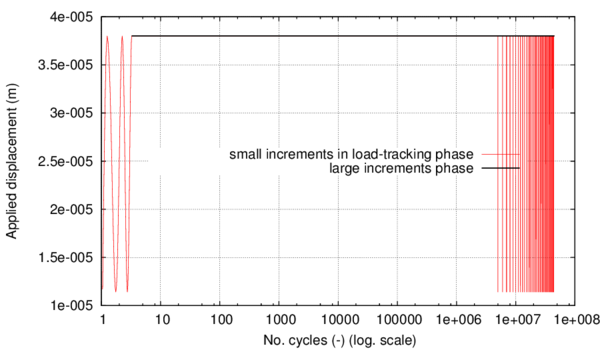Figure 61: Applied displacement for load case ${S}_{1}$

The stresses induced by the cyclic displacement applied lead to a fatigue life, according to the material Wöhler curve, of 4,9 x 10${\textstyle {}^{6}}$ cycles. This number of cycles marks the beginning of the nonlinear process and, therefore, of the energy dissipation.

In figure 62, the stress in the specimen, the material Wöhler curve, the residual strength variable and the material damage value are presented. It can be seen that, while the residual strength curve is above the Wohler fatigue life curve, there is no stress alteration or damage accumulation.

The stress level shown refers to the instantaneous stress divided by the initial elastic threshold. It can be seen that at the beginning an initial load-tracking stage is necessary for recording the characteristics of the stress evolution: reversion factor, maximum stress value and stress amplitude in every cycle. When the cyclic stress characteristics do not change from one loading cycle to the other (at every Gauss point) large increments start to be applied, where the load is maintained at the maximum value reached in previous cycles and only the number of cycles variable increases. At this point the material is considered to be in an elastic state but the evolution of the S-N and of the residual strength curves continues to be monitored.

Once the number of cycles applied reaches the fatigue life defined by the Wöhler curve for that level of maximum stress, event marked by the stress curve intersecting the Wöhler curve, it also intersects the residual strength curve. After this point, the stress in the material is higher than the residual strength and a softening process begins with the consequent increase of the damage variable. As soon as this event occurs at the first Gauss point a load-tracking stage is automatically activated for the entire finite element model. This is necessary as the onset of the degradation process leads to a change in the internal forces and a new equilibrium configuration is reached. When this equilibrium configuration has stabilized under small increments, the stress characteristics from one cycle to the other remain constant. This enables the application of a large increment, maintaining the load at the maximum level reached in previous stable cycles. However, when applying this large increment the equilibrium is lost again as the structure is pushed further in the nonlinear zone with a consequent damage accumulation and stiffness loss. This effect can be seen in the stress relaxation occurring at the end of each large scale increment (figure 62 and figure 63).

From that point forward, after each large increment where ${\textstyle {\dot {d}}>0}$, the load-tracking phase is automatically activated so that damage evolution can be monitored from cycle to cycle. If, after describing several cycles with small increments, the stress state throughout the solid has stabilized, a new large increment can be applied. This process is repeated automatically until the material reaches a state of complete degradation.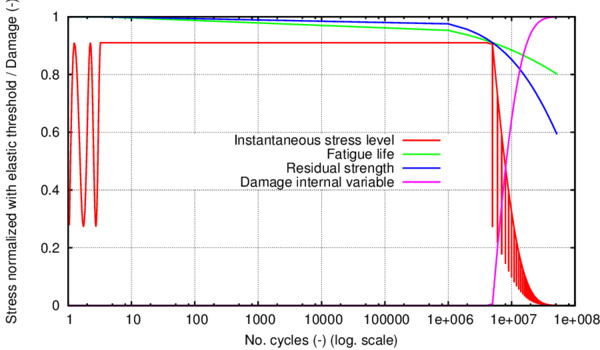Figure 62: Parameters of interest for the fatigue analysis under load ${S}_{1}$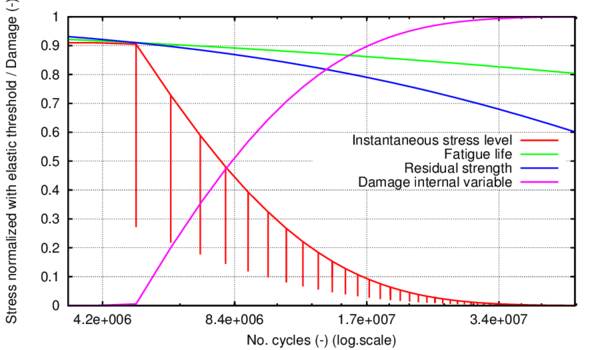Figure 63: Parameters of interest for the fatigue analysis under load ${S}_{1}$ in the nonlinear zone

Figure 63 zooms the end part of figure 62 to show more clearly the material performance described. The load-tracking phase is represented by the vertical lines. The large increments phase is represented by the in between segments. Both figure 62 and figure 63 have a logarithmical scale along the horizontal axis.

In figure 64, the effects of material degradation are shown in the stress-strain curve. The vertical axis refers to the instantaneous stress level divided by the material elastic limit. The large increments phase occurs only when the displacement is maintained at its maximum value and ${\textstyle \varepsilon =\varepsilon _{\max }}$. Therefore, it is represented in this figure by the vertical lines descending from the point of maximum stress. The stress interval represented by each jump in the stress level quantifies the stress softening caused by a single, large number of cycles, interval. Each of these stress-softening intervals is followed by a few unloading (until minimum displacement) - loading cycles. These mark the load-tracking phase where a clear change in material stiffness is visible.

It can be seen that, as the material progressively suffers loss of stiffness, for the same large step there is less stress softening.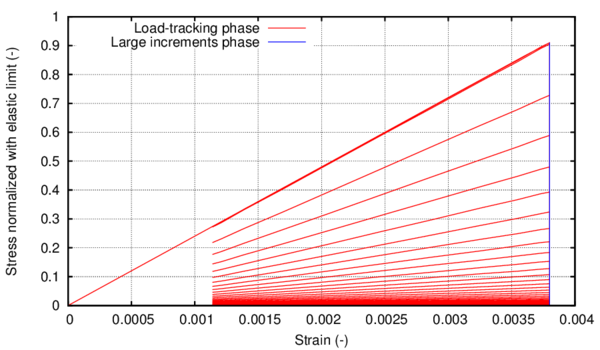Figure 64: Stress-Strain at integration point for load ${S}_{1}$

Furthermore, in order to demonstrate the good accuracy of the method, this simulation has been done with four different sizes for the large increments phase. Figure 65 presents the evolution of the damage internal variable (as resulting from equation 40) with the number of cycles in all four cases. It can be seen that, even though the simulation is done by using different steps in the large increments phase: 50.000, 100.000, 1 x 10${\textstyle {}^{6}}$ and 5 x 10${\textstyle {}^{6}}$ cycles, the damage variable has the same evolution.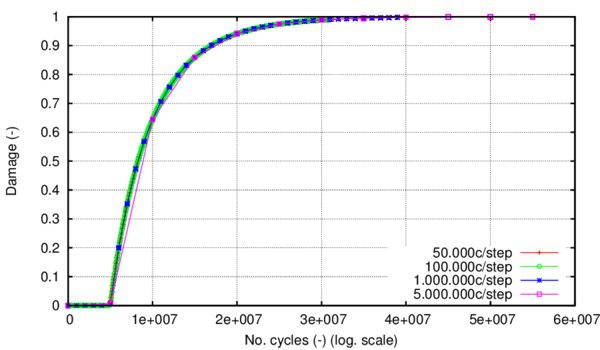Figure 65: Evolution of the damage internal variable with the number of cycles

As indicated in Section 4.3.4 the number of cycles used as a step in the large increments phase is a key parameter for the load-advancing algorithm. In table 11 the computational time obtained for the different N${}_{c}$ steps is presented. It can be seen that, even though the simulation is made on a single linear element, computational time can vary considerably depending on the number of cycles chosen as time step.

 No. of cycles considered as step 50.000 100.000 1 x 10${\textstyle {}^{6}}$ 5 x 10${\textstyle {}^{6}}$ CPU time 0h: 8':42 0h: 4': 8 0h: 0':30 0h: 0':10

A comparison was made between the computational time required when using the proposed load-advancing strategy and the computational time needed if in the nonlinear stage only load-tracking were to be used. As it can be seen from table 11, the CPU time needed in the case of a step of 1 x 10${\textstyle {}^{6}}$ cycles was 30 seconds for a total analysis of 53.5 x 10${\textstyle {}^{6}}$ cycles, out of which 4.9 x 10${\textstyle {}^{6}}$ cycles consisted of elastic behaviour. When advancing only by load-tracking after the 4.9 x 10${\textstyle {}^{6}}$ elastic cycles, in 10 hours of computational time only 84.000 cycles were computed, with a correspondant damage level at the end of the 84.000 cycles of 0.018. Taking into account this rate of advance, if the entire analysis of 53.5 x 10${\textstyle {}^{6}}$ cycles were to be conducted under load-tracking, a computational time of 265 days would be needed. This study was carried out on only one linear element. In real-life simulations, the dimensions of the problem are much larger and load history is more complex and this demonstrates that it is of the upmost importance having a load-saving advancing strategy.

An important feature of the constititutive model coupled with the load-advancing strategy is the ability to account for the order in which different loads are applied within a determined load history. In order to demonstrate this, a different regular cyclic load will be shown and, in the next section, the combination of the two loads, ${\textstyle {S}_{1}}$ and ${\textstyle {S}_{2}}$ will be analysed.

The characteristics of the tensional state induced by the load ${\textstyle {S}_{2}}$ are shown in table 12. In figure 66, the stress evolution at the integration point, the material Wöhler curve, the residual strength variable and the material damage value are presented, and in figure 67 the effects of material degradation are shown in the stress-strain curve.

 (normalized with threshold limit) Case code Reversion factor Max. Stress PG Min. Stress PG Med. Stress PG Nc when damage initiates ${\textstyle {S}_{2}}$ 0 0,839 0 0,42 3,46E+06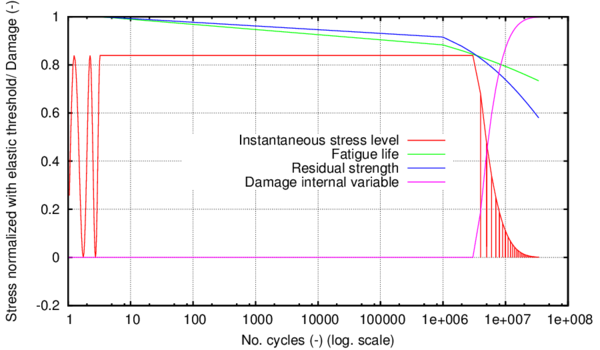Figure 66: Parameters of interest for the fatigue analysis under load ${S}_{2}$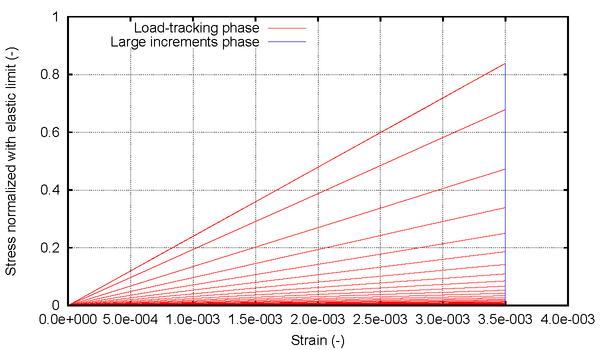Figure 67: Stress-Strain at integration point for load ${S}_{2}$

In table 13 the stresses generated at integration point level by the imposed maximum and minimum displacements are included. These are displayed for the two cyclical loads applied, ${\textstyle {S}_{1}}$ and ${\textstyle {S}_{2}}$, as well as the fatigue life resulting from the FEM model if only one of the loads, be it ${\textstyle {S}_{1}}$ or ${\textstyle {S}_{2}}$, were to be applied until fracture. Table 13 also includes the number of cycles at which damage starts when applying both ${\textstyle {S}_{1}}$ and ${\textstyle {S}_{2}}$.

 (normalized with threshold limit) Case code Reversion factor Max. Stress PG Min. Stress PG Med. Stress PG Nc when damage initiates ${\textstyle {S}_{1}}$ 0,3 0,91 0,273 0,59 4,90E+06 ${\textstyle {S}_{2}}$ 0 0,839 0 0,42 3,46E+06 ${\textstyle {S}_{1}}$+${\textstyle {S}_{2}}$ 3,62E+06 ${\textstyle {S}_{2}}$+${\textstyle {S}_{1}}$ 1,22E+06

The simulation of the load combination was made by first imposing the ${\textstyle {S}_{1}}$ load during 10${\textstyle {}^{6}}$cycles, followed by load ${\textstyle {S}_{2}}$ being applied from 10${\textstyle {}^{6}}$ cycles to 10${\textstyle {}^{8}}$ cycles. By applying first load ${\textstyle {S}_{1}}$, followed by load ${\textstyle {S}_{2}}$, the resulting life for the material is 3,62 x 10${\textstyle {}^{6}}$ cycles, less than if only load ${\textstyle {S}_{1}}$ were applied, but more than if only ${\textstyle {S}_{2}}$ were applied. This shows that the formulation is capable of taking into account the effect of a cyclical load even if it is applied for a number of cycles that does not lead to failure. This is achieved by quantifying the strength reduction caused by each event in the load history and by dynamically describing the ${\textstyle S-N}$ curve according to changes in load characteristics.

In figure 68 it can be seen how the slope of both curves, residual strength and ${\textstyle S-N}$ curve, changes as a different load starts being applied. This is possible due to the load-tracking phase being automatically activated once a change in the load is detected, as can be seen from the vertical line dividing the two maximum stress levels exhibited. The intermediate load-tracking phase appears as a straight line due to the fact that a logarithmical scale has been used for the horizontal axis. The characteristics of the new load are consequently determined, maximum stress level and reversion factor, and with these parameters the ${\textstyle S-N}$ and reduction curve readjust themselves.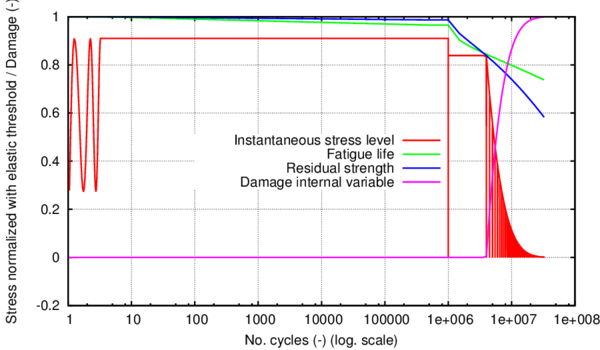Figure 68: Parameters of interest for the fatigue analysis under loads ${S}_{1}$+${S}_{2}$

Figure 69 shows the stress – strain curve where the stiffness reduction can be seen at each automatic unloading. The vertical axis refers to the instantaneous stress divided by the elastic limit. The trigger for the load-tracking stage is ${\textstyle {\dot {d}}>0}$ in an increment i. When this happens, the load factor for increment i+1 will be correspondent to the load-tracking phase and unloading will have begun. Each cycle will be described by small increments until the stress state is stable again from one cycle to the other. The curve is in accordance with figure 68, showing that the material is in the elastic state while load ${\textstyle {S}_{1}}$ is applied and the equivalent stress is taken to its maximum value: 0.91 of the elastic limit. Load ${\textstyle {S}_{2}}$ is then applied and takes the material to its fatigue life as indicated by the dynamically updated Wöhler curve. All the stiffness reduction occurs after this point. As seen in the previous case, a higher stiffness reduction can be seen in the first stages of the nonlinear process. Each one of the vertical segments depicting stress relaxation in figure 69 corresponds to a large increments phase that occurs only when ${\textstyle \varepsilon =\varepsilon _{\max }^{{S}_{2}}=0.0035}$ . The inclined lines mark the posterior load-tracking phase with material loading-unloading.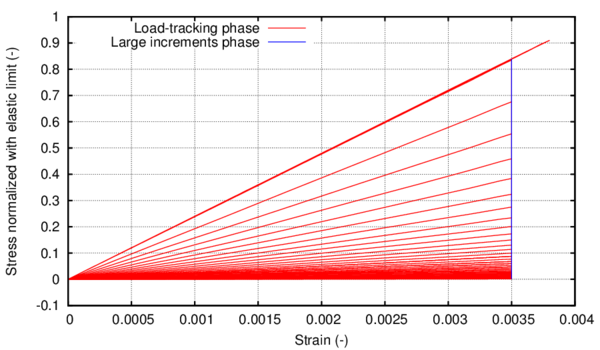Figure 69: Stress-Strain at integration point for loads ${S}_{1}$+${S}_{2}$

Another case is shown in figures 70 and 71 where the order of the loads is inverted. First, 10${\textstyle {}^{6}}$ cycles of load ${\textstyle {S}_{2}}$ are applied, with its consequent strength reduction. After that, the load changes to ${\textstyle {S}_{1}}$ and, in the first cycle it is applied, the maximum stress induced in the material is higher than the updated fatigue life curve. However, the updated material strength is above this value and the damage threshold in the constitutive model will not be crossed yet. When the stress state stabilizes under load ${\textstyle {S}_{1}}$ a large increment is applied, and, due to this change in ${\textstyle {N}_{c}}$, the strength threshold is reduced below the maximum stress level material and the damage accumulation process starts. The switch is made back and forth between load-tracking and large increments until all the fracture energy of the material is dissipated.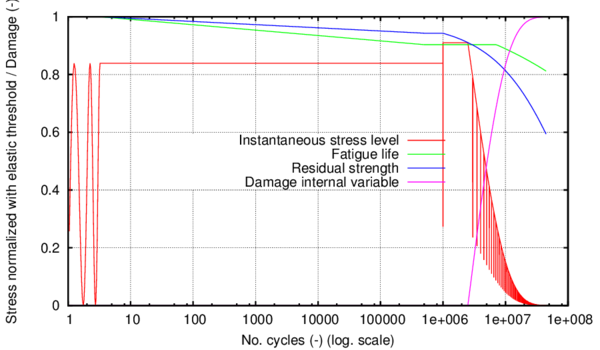Figure 70: Parameters of interest for the fatigue analysis under loads ${S}_{2}$+${S}_{1}$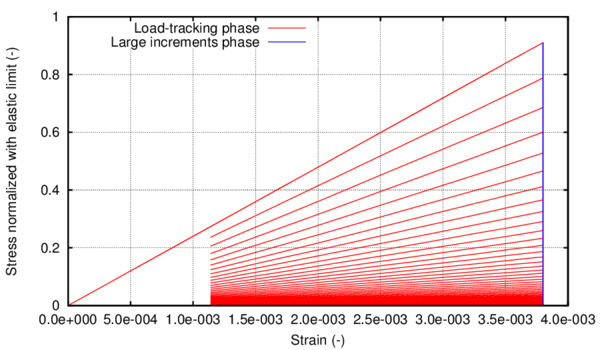Figure 71: Stress-Strain at integration point for loads ${S}_{2}$+${S}_{1}$

Following, several loading scenarios have been analysed that include a cyclical load and a monotonic one. A comparison is made between the material behaviour when failure occurs due to monotonic loading exclusively, due to cyclic loading exclusively or due to combinations of the two. Specifically, in the first case analysed the cyclic loading ${\textstyle {S}_{2}}$ is applied 10${\textstyle {}^{6}}$ cycles and then the material is taken to complete failure by monotonic loading. In the second case the same cyclic load is maintained 6,42 x 10${\textstyle {}^{6}}$ cycles and a monotonic load is applied after that, until total failure.

The constitutive model quantifies the energy dissipation only after the ${\textstyle S-N}$ curve has been crossed and the updated damage threshold surpassed. A question is raised then on whether dissipation occurs also before this point, in the span of ${\textstyle {N}_{c}}$ when the initial elastic limit starts to be reduced, but the damage threshold has not been crossed yet.

This reduction in strength corresponds, from a physical point of view, to a rearrangement of the internal structure of the material, slip of metal grains and potential nucleation of voids. The dissipative cost of this process can be quantified indirectly by comparing the behaviour of the material taken to failure by a monotonic load, by a cyclical load and by different combinations of the two.

As said before, four different cases have been analysed, as shown in table 14. A different visual representation of the results has been chosen for these combinations in order to better differentiate cyclic from monotonic effects in a more graphical way.

 Case no. Type of load ${N}_{c}$applied 1 cyclic + monotonic 10${\textstyle {}^{6}}$ 2 cyclic + monotonic 6.42 x 10${\textstyle {}^{6}}$ 3 cyclic 34 x 10${\textstyle {}^{6}}$ 4 monotonic 0

Figures 72, 73 and 74 show the parameters of interest for the fatigue damage model in a three-dimensional space. In the following, the X axis refers to the number of cycles axis where a logarithmical scale is used. On the Y axis the damage internal variable is portrayed, and in the Z axis the stress is quantified, scaled with the value of the initial elastic limit. While damage accumulation has not started, the curves of interest are contained in the XZ plane, same as the plots shown in previous cases. However, when the damage threshold is crossed the stress curve is taken outside of this plane by representing its evolution with the help of the Y axis where the damage variable is quantified.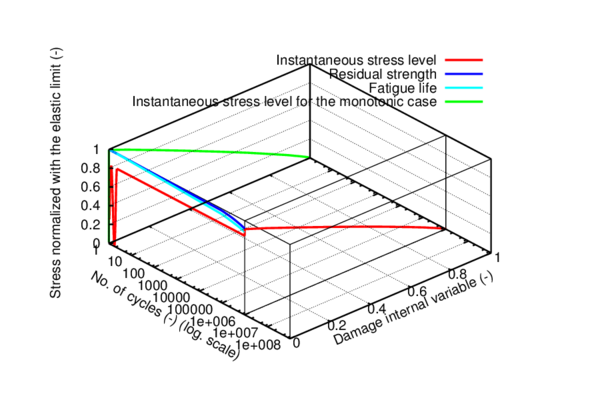Figure 72: Parameters of interest for the fatigue analysis under load combination 1 in 3d representation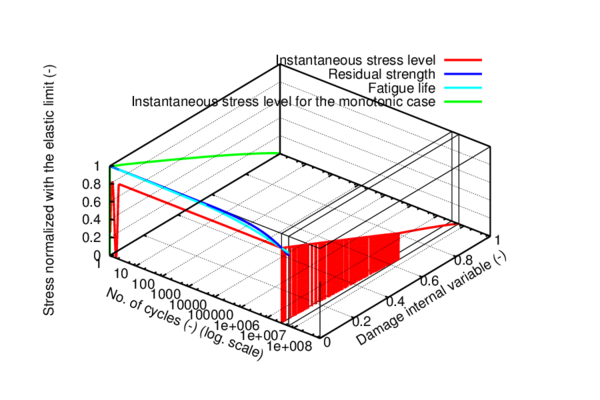Figure 73: Parameters of interest for the fatigue analysis under load combination 2 in 3d representation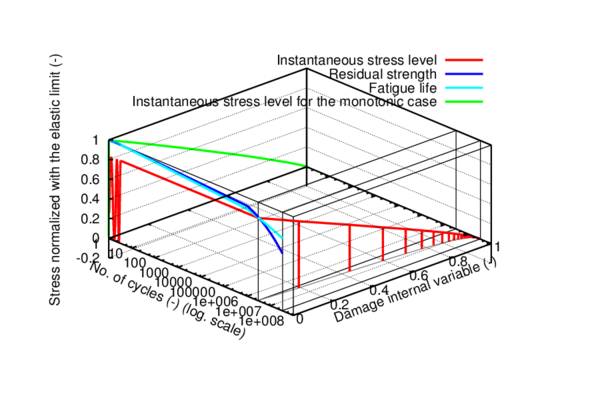Figure 74: Parameters of interest for the fatigue analysis under load ${S}_{2}$ in 3d representation

Put in different words, all the YZ planes perpendicular to the fatigue curves in plane XZ show the dissipation for a monotonic loading applied at the ${\textstyle {N}_{c}}$ where the plane intersects the X axis.

For instance in figure 72 the curves of interest are shown for case 1 in table 14. While the cyclic load is being applied the variables of interest are found in the XZ plane, where the strength reduction and the fatigue life are depicted. After 10${\textstyle {}^{6}}$ cycles the cyclic load is stopped and is then increased monotonically until failure (${\textstyle d=1}$). When the load starts to be applied monotonically the material is still in the elastic state, but its elastic limit has been reduced. Therefore, damage starts earlier than if the cyclic load would not have been applied. The entire evolution of the stress curve after damage initiates in the model is contained in the plane perpendicular to the ${\textstyle {N}_{c}}$ axis. On the same chart, the evolution of the stress is plotted for the monotonic case (Case 4 in table 14). In order to be able to represent both curves on the same chart the monotonic stress curve has been plotted in the plane with an abscissa of ${\textstyle 1}$, even though the load was exclusively monotonic.

The same variables are shown in figure 73 for case 2 in table 14 and in figure 74 for case 3 in table 14. All the figures include the evolution of the monotonic stress curve for reference. It can be seen that as damage starts to accumulate due to cyclic loading the stress curve is no longer contained in a YZ plane but rather it describes a curvilinear trajectory in space, as can be seen clearly for case 3 in figure 74. The intersection of this curvilinear trajectory with a given YZ plane marks the beginning of the dissipation if the load were monotonic after that given ${\textstyle {N}_{c}}$. An example of the above is figure 73 for case 2 in table 14 where after 6.42 x 10${\textstyle {}^{6}}$ cycles the load is increased monotonically until failure and the remaining energy is dissipated in the corresponding YZ plane (with an abscissa of 6.42 x 10${\textstyle {}^{6}}$ cycles).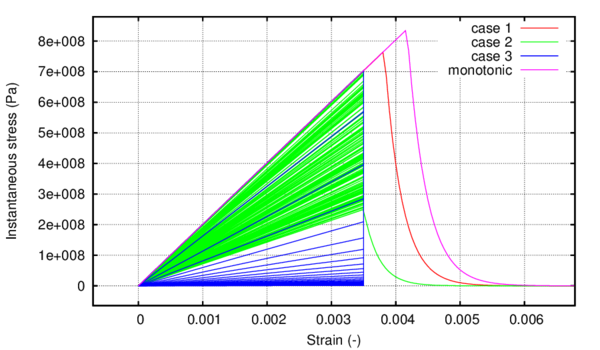Figure 75: Comparison between the stress-strain curves at the integration point for different load combinations

In figure 75 the dissipative cost of the strength reduction occurring in the elastic zone can be seen by comparing the stress-strain curve for the monotonic case and for the other cases. The maximum energy dissipation cost (maximum area difference with respect to the monotonic curve) is visible for the case with only cyclic loading applied (case 3 in table 14).

For a final overview on the different stress histories used in this analysis, figure 76 can be consulted. The stress due to monotonic loads has been marked for each case analysed.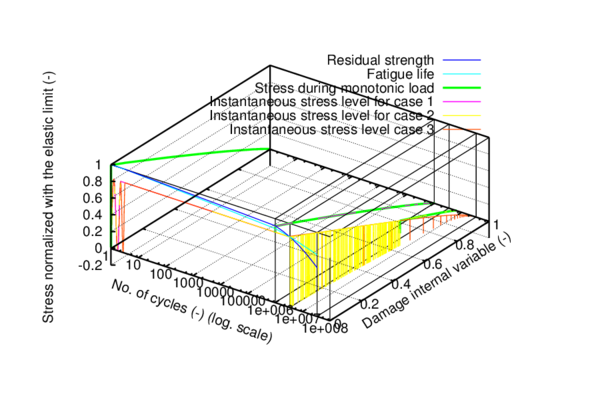Figure 76: Comparison between the stress evolution at the integration point for different load combinations in 3d representation

## 4.5 Validation of the formulation proposed

### 4.5.1 Problem definition. Geometry and material.

The experiment undertaken by Marines et al.  has been chosen to validate the stepwise load advancing strategy presented in this work, together with the continuum damage model for fatigue described in section 4.2. In table 15, the material characteristics given by the aforementioned authors are presented along with the shape and dimensions of the fatigue specimen adopted in the experiment. The experimental results obtained with a loading frequency of 20 kHz and a reversion factor ${\textstyle R=-1}$ have been taken into account for the comparison with numerical results.

 E${\textstyle {}_{d(10kHz)}}$ (GPa) E${\textstyle {}_{d(20kHz)}}$ (GPa) $\sigma$${}_{y(0.2\%)}$ (MPa) UTS (MPa) A (%) $\rho$ (kg/m${\textstyle {}^{3}}$) 208.3 211.5 608 878 20 7850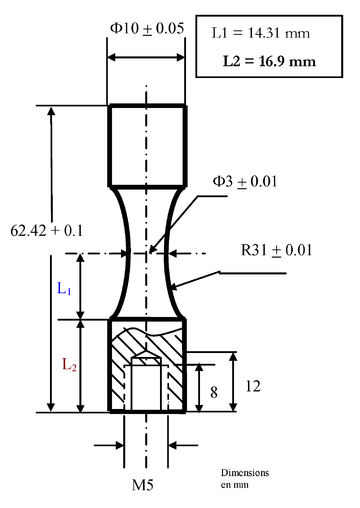] Figure 77: Shape and dimension of ultrasonic fatigue specimen as given by Marines et al.

### 4.5.2 Finite element model

The fatigue specimen presented in figure 77 has been reproduced by means of a finite element model. Due to the symmetrical nature of the geometry, loading and boundary conditions, only half of the specimen has been modelled in order to minimize computational time.

The semi-cylindrical volume has been meshed with linear hexahedral elements as shown in figure 78. The mesh has 2666 nodes and 1920 elements. Each finite element is described with eight integration points.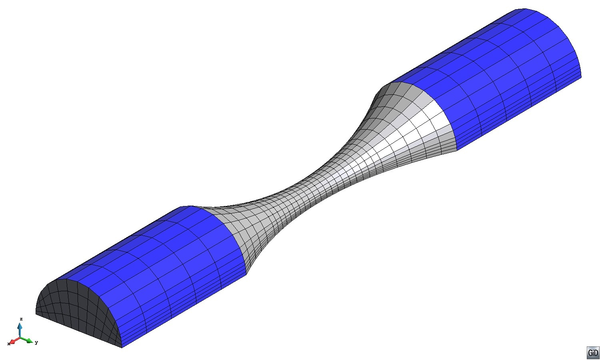Figure 78: Finite element mesh of linear hexahedral elements

The boundary conditions applied are shown in figure 79. The specimen is restrained at one end and subjected to a cyclical displacement at the other end. The entire base is defined with a symmetry condition.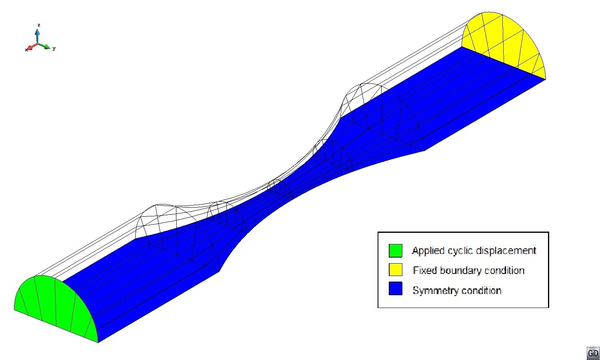Figure 79: Boundary and loading conditions for the analysed geometry

The analytical formulation for the ${\textstyle S-N}$ curve used by the numerical model was the one presented in . The parameters used for the adjustment of the curve to the experimental one proposed by Marines et al.  are S${\textstyle {}_{u}}$= 608Mpa, S${\textstyle {}_{lim}}$=325Mpa, ${\textstyle \alpha }$ = ${\textstyle \beta }$ =1.3${\textstyle ;}$ a correction factor of 1.23 was applied when calculating the auxiliary term ${\textstyle AUX=1/1.26+R/3.3}$.

### 4.5.3 Results and discussion

In figure 80, the results obtained by Marines et al.  are presented. As expected, a scatter of test results can be seen both for 20 kHz resonating material and for the 30 kHz one. Since the material characteristics taken into account for the numerical analysis are that of the 20 kHz one, simulations have been run only with the maximum stress levels exhibited for this particular testing frequency. The experimental results showed that, even though the geometry and loading conditions are axisymmetric, the failure mode is not symmetric .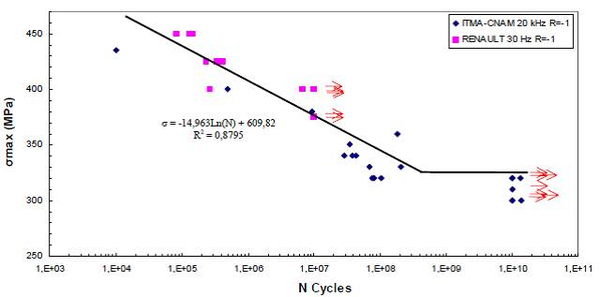] Figure 80: Fatigue S-N curve of HSLA steel D38MSV5S with R = -1, 20 kHz and 30kHz 

#### 4.5.3.1 Validation of the results obtained with the numerical simulation

The expression for the ${\textstyle S-N}$ curve proposed in figure 80 by Marines et al.  has been compared to the numerical results obtained from the described finite element model. The comparison can be seen in figure 81. Since the model has a deterministic nature, no scatter can be seen in numerical results. The numerical data depicted in figure 81 is in accordance with table 16 where the information on the exact characteristics of each simulation case is given.

 Case code Reversion factor Max. Stress GP (MPa) Nc at which damage initiates BA1 -1 435 0,17 * 10${\textstyle {}^{6}}$ BA2 -1 400 1,50 * 10${\textstyle {}^{6}}$ BA3 -1 380 5,30 * 10${\textstyle {}^{6}}$ BA4 -1 360 20,03 * 10${\textstyle {}^{6}}$ BA5 -1 350 39,50 * 10${\textstyle {}^{6}}$ BA6 -1 340 78,50 * 10${\textstyle {}^{6}}$ BA7 -1 330 160,00 * 10${\textstyle {}^{6}}$ BA8 -1 320 1E9 (run-out)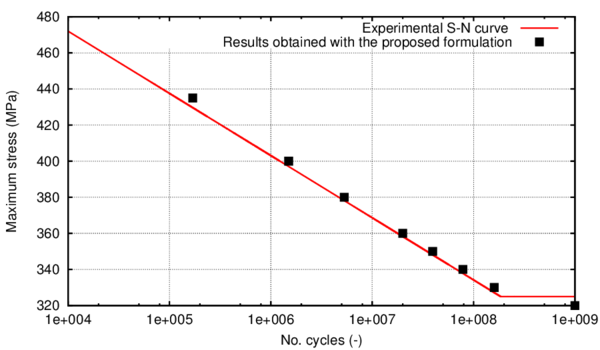Figure 81: $S-N$ curve for HSLA steel D38MSV5S. Experimental vs. numerical.

Some observations are to be made, however, in order to better understand the results presented in figure 81. The points signalled as results obtained with the proposed formulation, mark the start of the stiffness reduction. In a force controlled test, when the force level is taken to the following maximum after the fatigue life depicted in figure 81, abrupt failure occurs. However, as the numerical model has been defined with displacement controlled boundary conditions, when the next maximum displacement is reached after arriving at the fatigue life given in table 16, a reduction of the stress level occurs and the material can continue to be cyclically loaded. If an unloading would be made at this point a stiffness reduction in proportion to the stress relaxation would be observed. For a better understanding of this effect a detailed description of the fatigue behaviour is shown in the next section for one of the cases ran for the validation.

Summarizing, the fatigue life charted in figure 81 represents the number of cycles up until which the maximum stress induced by the applied cyclical displacement remains unaltered. Once this point is surpassed, stress relaxation occurs along with damage accumulation, stiffness reduction and a subsequent change in internal forces. If the test is force controlled, the specimen fractures abruptly. If the test is displacement controlled, the material is taken to complete fracture progressively.

#### 4.5.3.2 Analysis of the performance of the material model. In-depth analysis of stiffness reduction at integration point

In this section an in-depth analysis of the behaviour of the material is made for a maximum induced stress level of 350 MPa. The results are similar for all the other simulations ran.

The experimental tests have shown that fracture initiates on the surface of the specimen. This is due to defects present in the microstructure that lead to void nucleation and microcrack initiation and propagation. Fracture initiation is not symmetric and, furthermore, in the last stages of the nonlinear process, due to microstructural imperfections and defects, fracture propagation leads to non-symmetrical behaviour.

In the numerical simulation, same as in the experiment, fracture initiation is not symmetrical, but a large part of the propagation process occurs under symmetry to the y-y axis. However, in the last stages, before complete rupture (third image in figure 84), due to numerical round off the solution loses symmetry.

A monitoring of model parameters has been made at the first integration point that shows complete degradation. In figure 82 are depicted: the evolution of the residual strength, Wöhler fatigue life, equivalent stress and damage internal variable with a logarithmical scale along the horizontal axis. It can be seen that the stress state suffers no alteration until it intersects the ${\textstyle S-N}$ curve, same as in the numerical examples shown so far.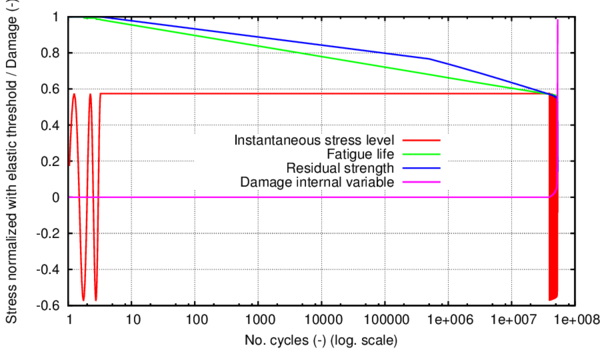Figure 82: Parameters of interest for the fatigue analysis of D38MSV5S specimen at the first integration point that fractures completely

Figure 83 shows a zoom on the evolution of the variables in the non-linear zone (past the intersection with the ${\textstyle S-N}$ curve). Here the succession between the large increments phase and the load-tracking phase can be better seen. Also, it can be observed how 90% of the degradation is concentrated in the last two large steps with nearly 80% in the last one. For this case, 0.5 x 10${\textstyle {}^{6}}$ cycles has been adopted as step for the large increments phase. Therefore, although the specimen is subjected to approximately 53.5 x 10${\textstyle {}^{6}}$ cycles until it fractures completely, 80% of the stiffness reduction occurs in the last 500000 cycles, deeming the fracture a "brittle" type one.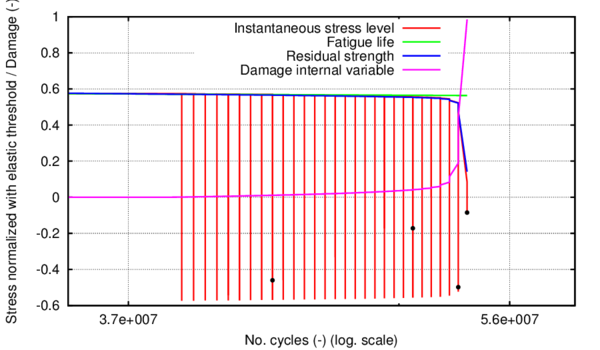Figure 83: Zoom on fatigue parameters of interest in the nonlinear zone (post $S-N$ curve).Dots indicate the analysis steps at which damage evolution is presented in figure 84

Figure 84 shows a view of the specimen from above and a view of the cross section at different number of cycles (analysis steps). Each one of the steps represented is marked in figure 83 chronologically with a black dot.

The images show the damage evolution in the specimen, both on the surface and in depth, as the number of cycles increases. Damage initiates on the surface of the specimen in the area with the smallest cross-sectional diameter, as expected, after 39.5 x 10${\textstyle {}^{6}}$ cycles. Afterwards it propagates symmetrically until approximately 53 x 10${\textstyle {}^{6}}$ cycles (third image in figure 84), when it localizes in one side of the specimen. This is believed to be due to a numerical round off that directs the posterior damage accumulation to the right side of the specimen, as can be seen in figure 84.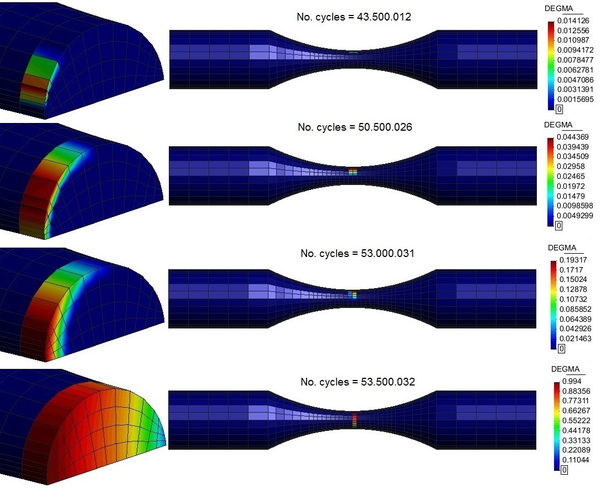Figure 84: Damage evolution for a maximum induced stress of 350MPa and R = -1

The upper image in figure 85 presents the deformed shape of the specimen when the last maximum displacement before total fracture is applied. A necking can be seen in the central region although the specimen is not yet completely fractured. From that point in the analysis, the applied displacement is taken from its maximum value to its minimum one, when the specimen is subjected to compression. That eventually causes the rupture into two parts (lower image in figure 85).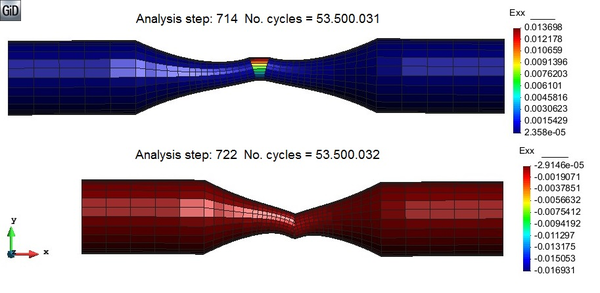Figure 85: Deformed shape (x 200) at the last maximum stress before rupture and at the last minimum stress, when rupture occurs

# 5 Constitutive modelling of Low Cycle Fatigue

This chapter presents a coupled plastic damage constitutive model valid for materials subjected to LCF. The model is applied in the softening regime leading to an improvement in material life prediction as compared to plasticity models. The energy participation factors for damage and plasticity are dependent on the number of cycles of loading the material has been previously subjected to. Numerical examples are presented to illustrate the behaviour and capabilities of the model in softening behaviour under monotonic load, and hardening - softening behaviour under cyclic load incorporating both kinematic and isotropic hardening.

## 5.1 Introduction

Natural hazards, such as earthquakes and landslides, have in most cases a devastating impact on civil infrastructures, as they lead to important material and economic loses. Among the elements most affected are pipelines and pipeline systems (water, oil, gas, etc.), that can be substantially damaged, and whose reparation cost can be extremely large. For example, according to the data reported by Kimishima et al.  , the number of water pipes that broke during the 2007 Niigata-Ken Chuetsu-Oki earthquake exceeded 550.

Under seismic loads pipelines suffer large cyclic deformations that can lead to their failure or that can undermine their strength capacity for their future use. In these cases, material failure is frequently driven by Low and Ultra-Low Cycle Fatigue phenomena (LCF and ULCF, respectively). Thus, in order to minimize the effect of these loads on pipelines and pipeline systems, and to assess the vulnerability of existing infrastructures, it is necessary to have appropriate analysis tools to simulate the failure mode of steel under ULCF and LCF.

Current work presents a new constitutive model especially developed for the prediction of material failure produced by LCF and ULCF. The agreement obtained between experimental and numerical results allow considering the proposed law an excellent tool for the simulation of pipelines under seismic conditions, as it allows predicting the strength capacity of a pipeline under any given seismic load or assessing the residual strength of the pipeline once the seismic load has already been applied.

Material failure due to LCF and ULCF will be simulated using a material non-linear model. This approach will allow the simulation of regular and non-regular cycles, as well as obtaining the post-critical response of the structure when the material has failed.

A material non-linear model is defined by the thermodynamic law that drives the material performance and a yield criterion defining the stress level that triggers the non-linear behaviour (, ). The constitutive law proposed herein is characterized by the combination of plasticity and damage. These two material laws have been already coupled by several authors proposing different models. These models vary in their complexity, versatility and accuracy. Some of them are those of Simo and Ju , Lubliner et al. , Luccioni et al.  and, more recently, Armero and Oller .

The necessity of combining these two laws is based on the following assumption: The plastic phenomenon leads to the distortion of metal voids and their coalescence. This effect is responsible of the permanent deformations obtained after steel yielding, characteristic of plasticity. However, this process does not account for the formation or nucleation of new voids, which may reduce the material stiffness . In order to include this effect in the material behaviour, a new law complementing plasticity is necessary. An increment of the voids in the microstructure of the material leads to a reduction of the effective area, resulting also in a reduction on the material stiffness. This effect will be simulated with a damage law.

The validity of this assumption is proved by a recent experimental campaign conducted in order to characterize the response of several steels for pipes (X52, X60 and X65) under ULCF and LCF loadings . These tests showed that while the material is in the hardening region, its performance is fully defined by plasticity. This is proved by the fact that successive hysteresis loops lay one over the other. However, when the hysteresis loop shrinks due to material softening, the material also loses some stiffness, effect noticed in the change in slope of the stress-strain curve when unloading. This behaviour corresponds to damage.

Therefore, in order to predict accurately the response of pipe steel under ULCF and LCF loads, the model must couple plasticity and damage in different stages. Initially, the material hardening behaviour will be associated with plasticity. Afterwards, the material softening behaviour will be associated to both damage and plasticity. The evolution of plastic strains is taken into account by the plasticity model and at the same time the void formation and their subsequent coalescence is quantified phenomenologically by the damage model. Finally, failure will take place when all the fracture energy of the material has been dissipated.

The initial plastic model used for the hardening region of steel is the one decribed in chapter 3 of the current document and already published in  and . This plasticity model is coupled with an isotropic damage model using the approach proposed in the work of Luccioni et al.  for monotonic loads. The coupling of these two models is made measuring, in every hysteresis cycle, the internal energy required by each model, as well as the available remaining energy of the material .

In the following section the formulation resulting from coupling the plasticity with the damage model is described in detail. Afterwards, section 5.3 presents the procedure defined to simulate LCF with the proposed constitutive law. Finally, section 5.4 compares the numerical results obtained with the proposed formulation with those obtained from the experimental campaign conducted by .

## 5.2 Plastic damage model formulation

### 5.2.1 Elasto-plastic damage model. Mechanical formulation.

The theories of plasticity and/or damage can simulate the material behaviour beyond the elastic range, taking into account the change in the strength of the material through the movement of the yield and/or damage surface (isotropic and kinematic) due to the inelastic behaviour (plasticity and damage) of each point of the solid. However they are not sensitive to cyclic load effects. In this work the standard inelastic theories are modified to introduce the fatigue effect coupled with non-fatigue material behaviour.

It is assumed that each point of the solid follows a damage-elasto-plastic constitutive law (stiffness hardening/softening) (,  and ) with the stress evolution depending on the free strain variable and plastic and damage internal variables. The formulation proposed herein studies the phenomenon of stiffness degradation and irreversible strain accumulation through the combined effect of damage and plasticity.

Since this work is oriented towards mechanical problems with small elastic strains and large inelastic strains, the free energy additively hypothesis is accepted ${\textstyle \Psi =\Psi ^{e}+\Psi ^{p}}$ (). The elastic ${\textstyle \Psi ^{e}}$ and plastic ${\textstyle \Psi ^{p}}$ parts of the free energy are written in the reference configuration for elastic Green strains ${\textstyle E_{ij}^{e}=E_{ij}-E_{ij}^{p}}$; the last variable operates as a free field variable , . The free energy is thus written as

 $\Psi =\;\Psi ^{e}(E_{ij}^{e},d)+\Psi ^{p}(\alpha ^{p})\;=\;(1-d){\frac {1}{2m^{o}}}\;\left[E_{ij}^{e}{C}_{ijkl}^{o}E_{kl}^{e}\right]+\Psi ^{p}(\alpha ^{p})$
(51)

Considering the second thermodynamic law (Clausius-Duhem inequality – ,  and ), the mechanical dissipation can be obtained as :

 $\Xi _{}={\frac {{\;}S_{ij}\,{\dot {E}}_{ij}^{p}}{m^{o}}}-{\frac {\partial \Psi }{\partial \alpha ^{p}}}{\dot {\alpha }}-{\frac {\partial \Psi }{\partial d}}{\dot {d}}\geq 0$
(52)

The fulfilment of this dissipation condition (Equation 52) demands that the expression of the stress should be defined as (Coleman method; see )

 $S_{ij}=m^{o}{\frac {\partial \Psi ^{}}{\partial E_{ij}^{}}}=(1-d)\;\,{C}_{ijkl}^{o}\left(E_{kl}^{}\right)$
(53)

Also, from the last expressions, the secant constitutive tensor can be obtained as:

 ${C}_{ijkl}^{s}\left(d\right)={\frac {\partial S_{ij}^{}}{\partial E_{kl}^{e}}}=m^{o}{\frac {\partial ^{2}\Psi ^{e}}{\partial E_{ij}^{e}\partial E_{kl}^{e}}}=(1-d)\;\,{C}_{ijkl}^{o}$
(54)

where ${\textstyle m^{o}}$ is the material density, ${\textstyle E_{ij}^{e},E_{ij},E_{ij}^{p}}$ are the elastic, total and plastic strain tensors, ${\textstyle d^{ini}\leq d\leq 1}$ is the internal damage variable enclosed between its initial value ${\textstyle d^{ini}}$ and its maximum value 1, ${\textstyle \alpha ^{p}}$ is a plastic internal variable, ${\textstyle {C}_{ijkl}^{0}}$ and ${\textstyle {C}_{ijkl}^{S}}$ are the original and secant constitutive tensors and ${\textstyle S_{ij}}$ is the stress tensor for a single material point.

### 5.2.2 Yield and potential plastic functions

The yield function ${\textstyle F^{P}}$accounts for the residual strength of the material, which depends on the current stress state and the plastic internal variables and, in the formulation proposed herein, it is sensitive to the fatigue phenomenon. This ${\textstyle F^{P}}$ function has the following form, taking into account isotropic and kinematic plastic hardening (Bauschinger effect ):

 $F^{P}(S_{ij},\alpha ^{p})=f^{P}(S_{ij}-\eta _{ij})-K^{P}(S_{ij},\kappa ^{p},N)\leq 0$
(55)

where ${\textstyle f^{P}(S_{ij}-\eta _{ij})}$ is the uniaxial equivalent stress function depending of the current value of the stresses ${\textstyle S_{ij}}$, ${\textstyle \eta _{ij}}$ is the kinematic plastic hardening internal variable, ${\textstyle K_{}^{P}\left(S_{ij}^{},\kappa ^{p},N\right)}$ is the plastic strength threshold and ${\textstyle \kappa ^{p}}$ is the plastic isotropic hardening internal variable (, , and ). ${\textstyle N}$ is the number of cycles of the stress in the point of the solid and ${\textstyle \alpha ^{p}}$ is a symbolic notation for all the plastic variables involved in the process.

Equation 55 incorporates the number of cycles as an internal variable that affects the strength threshold by lowering it as the number of cycles accumulates. This enables the model to also account for strength reduction due to high cycle fatigue effects (,  and ) and this in turn shows the potential of the formulation to adjust by itself to the type of fatigue involved.

The evolution law for the plastic strain is ${\textstyle {\dot {E}}_{ij}^{P}={\dot {\lambda }}{\frac {\partial G^{P}}{\partial S_{ij}}}}$, being ${\textstyle \lambda }$ the consistency plastic factor and ${\textstyle G^{P}}$ the plastic potential.

Kinematic hardening accounts for a translation of the yield function and allows the representation of the Bauschinger effect in the case of cyclic loading. This translation is driven by the kinematic hardening internal variable ${\textstyle \eta _{ij}}$ which, in a general case, varies proportionally to the plastic strain of the material point. One of the laws that define the evolution of this parameter is

 ${\dot {\eta }}_{ij}=c_{k}{\dot {E}}_{ij}^{P},\,\,{\hbox{with}}\,\,c_{k}={\frac {2}{3}}b_{k}\,\,{\hbox{for Von Mises}}$
(56)

where ${\textstyle b_{k}}$ is a material property to be determined by particular tests for the Prager and Melan kinematic hardening . The evolution of isotropic hardening is controlled by the evolution of the plastic hardening function ${\textstyle K^{P}}$, which is often defined by an internal variable ${\textstyle \kappa ^{p}}$. The rate equation for these two functions may be defined, respectively, by

 ${\begin{array}{l}{{\dot {K}}^{p}={\dot {\lambda }}\cdot {H}_{k}^{p}={h}_{k}^{p}\cdot {\dot {\kappa }}^{p}}\\{{\dot {\kappa }}^{p}={\dot {\lambda }}\cdot {H}_{k}^{p}={\dot {\lambda }}\cdot \left[h_{k}^{p}:{\frac {\partial G}{\partial S}}\right]={h}_{k}^{p}\cdot {\dot {E}}^{p}}\end{array}}$
(57)

where ${\textstyle k}$ denotes scalar and ${\textstyle {k}}$ stands for a tensor function. Depending on the functions defined to characterize these two parameters, different solid performances are obtained.

### 5.2.3 Threshold damage function

Onset of damage depends on the current stress state, the internal damage variable and, with the current formulation, it also depends on the number of cycles. The threshold damage function is defined as (see  and )

 ${\begin{array}{l}{F^{D}(S_{ij},d)=f^{D}(S_{ij})-K^{D}(S_{ij},d,N)\leq 0}\\{G^{D}(S_{ij},d)=g^{D}(S_{ij})=cte.}\end{array}}$
(58)

where ${\textstyle f^{D}(S_{ij})}$ is the equivalent stress function in the undamaged space, the damage strength threshold is ${\textstyle K^{D}(S_{ij},d,N)}$, and ${\textstyle d^{}=\int _{0}^{t}{\dot {d}}\,dt}$ the damage internal variable.

The evolution of the damage strength threshold is analogous to that of the plastic strength threshold, depending on the internal degradation variable ${\textstyle \kappa ^{d}}$